# RD Sharma Solutions for Class 10 Maths Chapter 9 Arithmetic Progressions

## RD Sharma Solutions Class 10 Maths Chapter 9 – Free PDF Download

RD Sharma Solutions for Class 10 Maths Chapter 9 – Arithmetic Progressions is provided here for students to study and excel in their board exams. In order to succeed the Class 10 Mathematics examinations, a structured way of understanding the concepts and solving problems is a must. The RD Sharma Solutions is on par with these needs for a student to secure good marks in the qualification exam. Furthermore, the solutions have been created by our expert team at BYJU’S, which is of simple language with appropriate explanations to give clarity on the concepts in the chapter.

Arithmetic Progressions of RD Sharma Class 10 is an exciting chapter where students can score marks easily. But due to some misconceptions, not all student can grab those marks. Thus to help students, the RD Sharma Solutions for Class 10 containing the answers of all six exercises is the apt way. This chapter’s key aspects are:

• Understanding sequences
• Arithmetic progressions
• Describing the sequence by writing the algebraic formula for its terms
• Find the sum of terms of in an A.P.
• Solving various word problems related to arithmetic progressions

## Download the PDF of RD Sharma Solutions For Class 10 Maths Chapter 9 Arithmetic Progressions here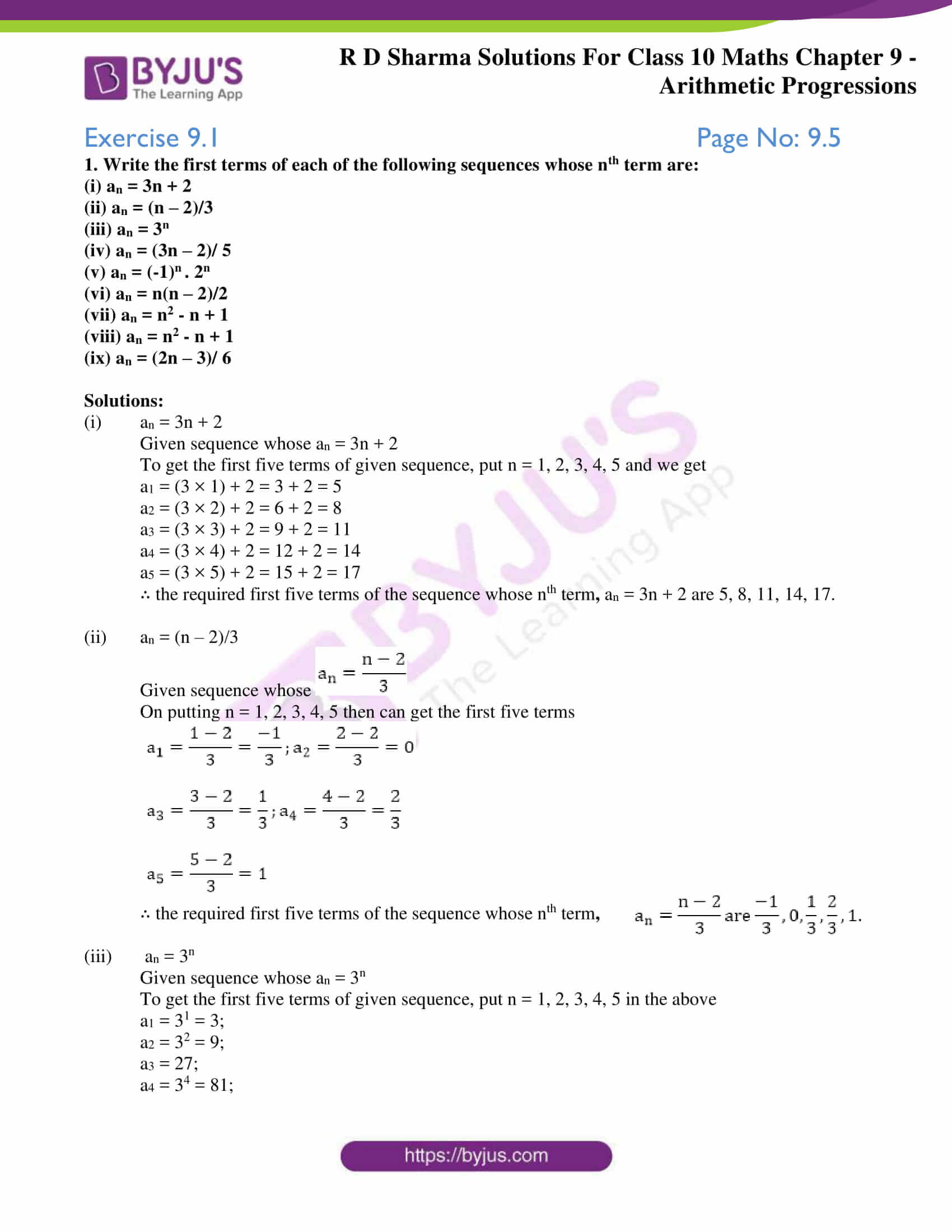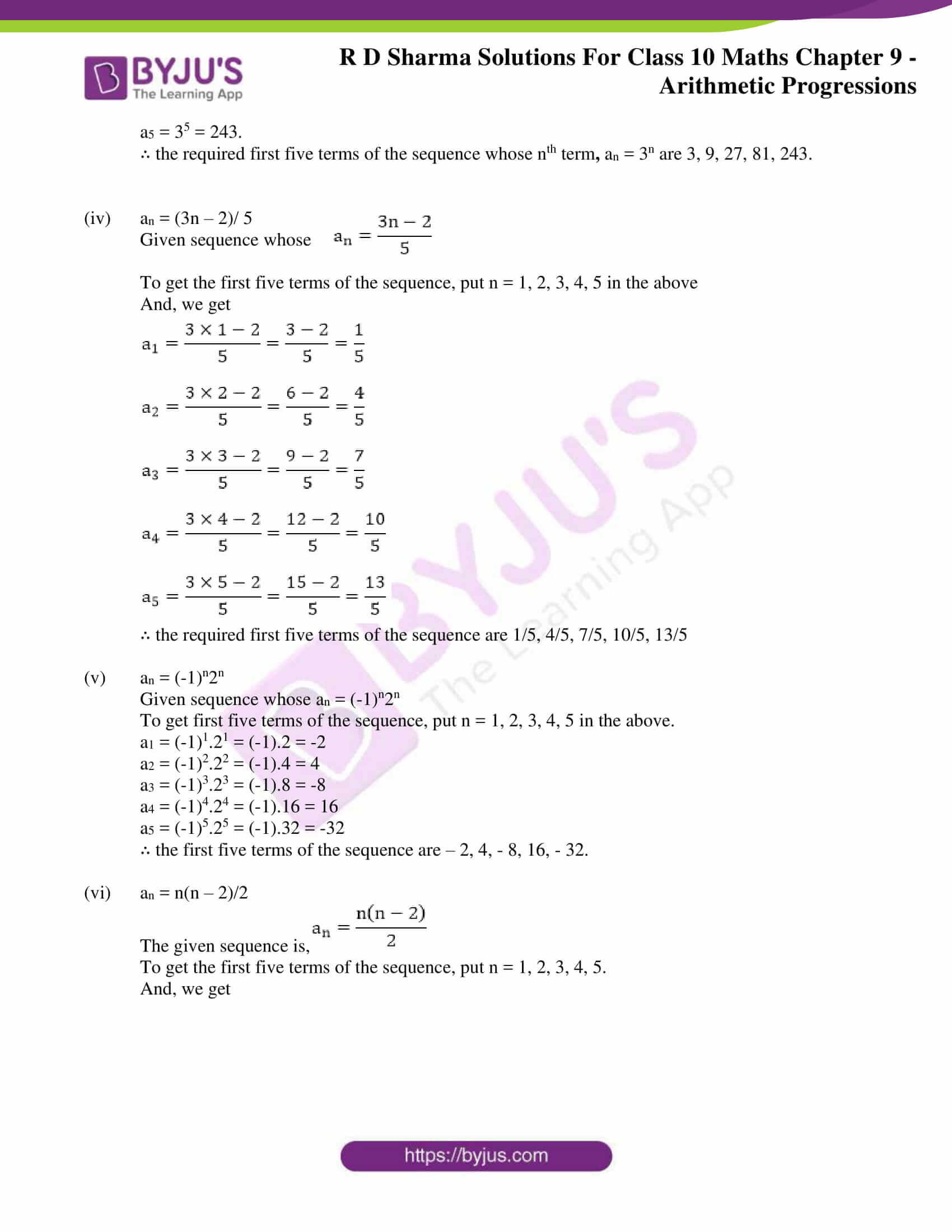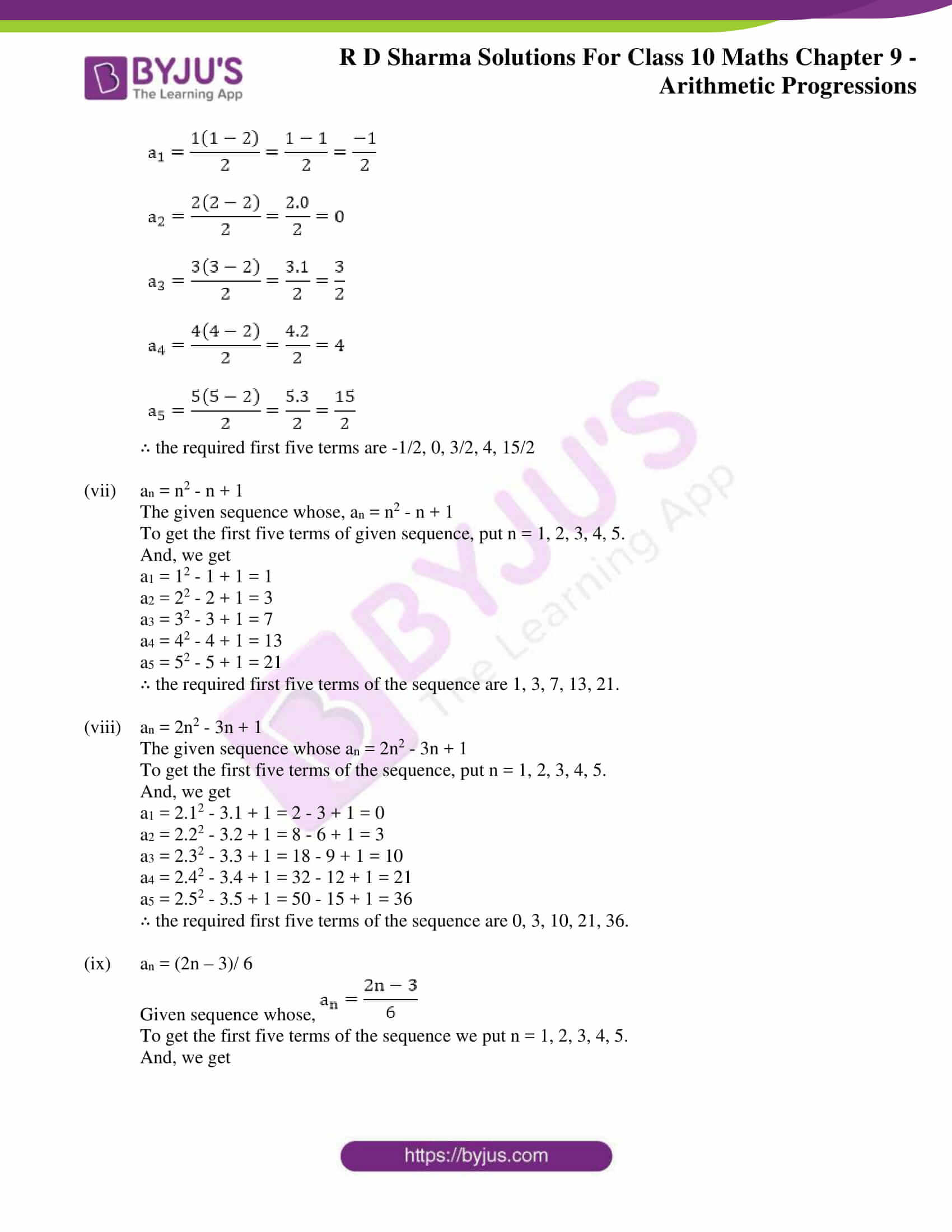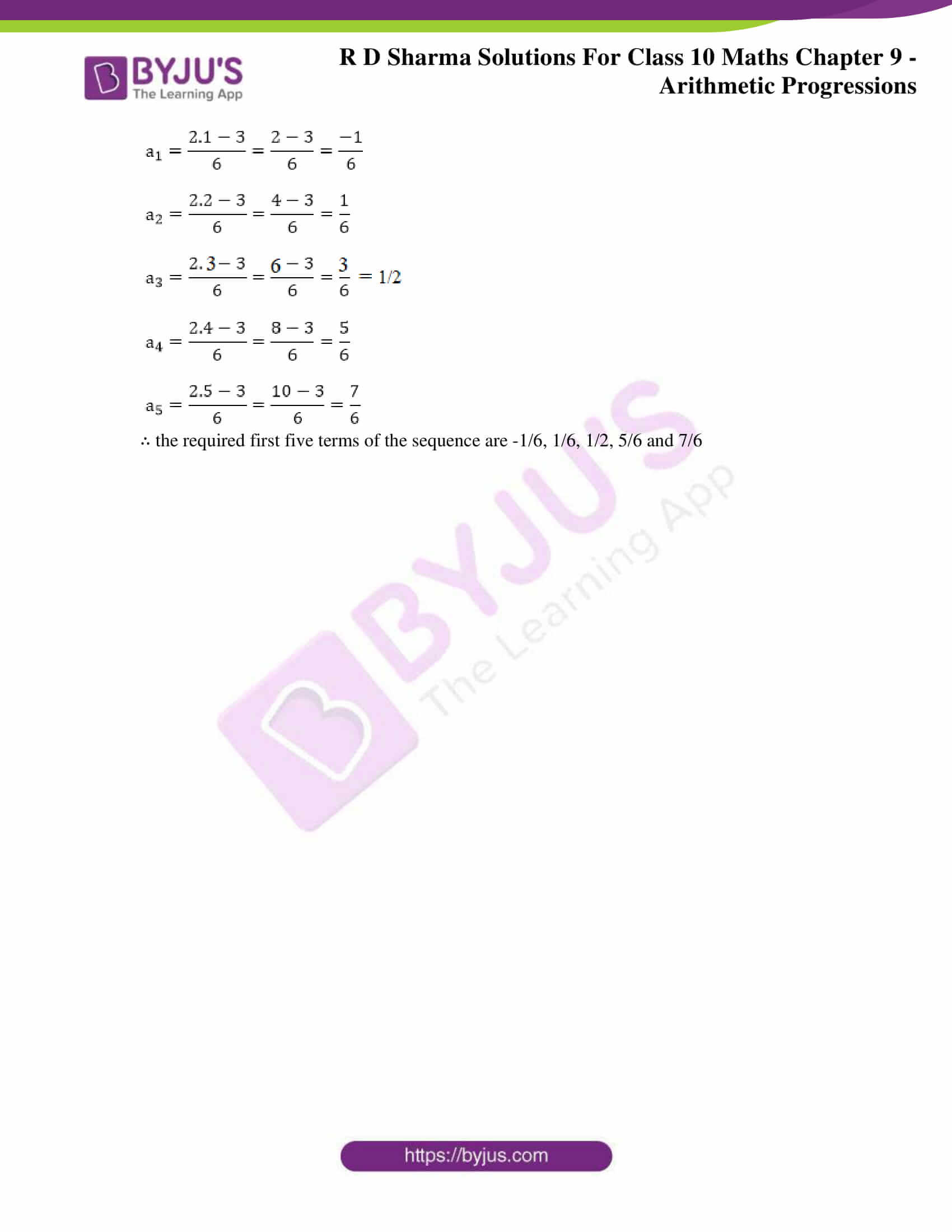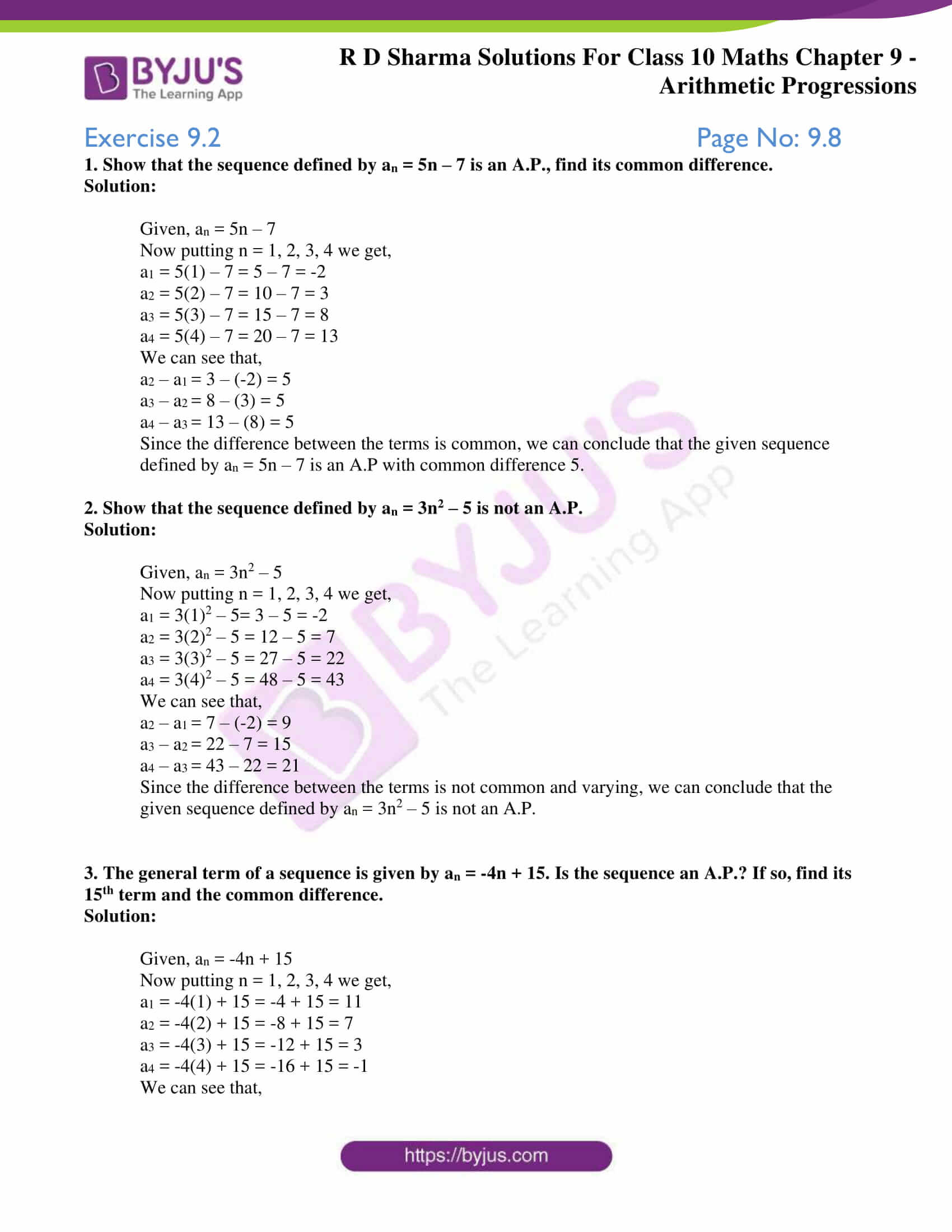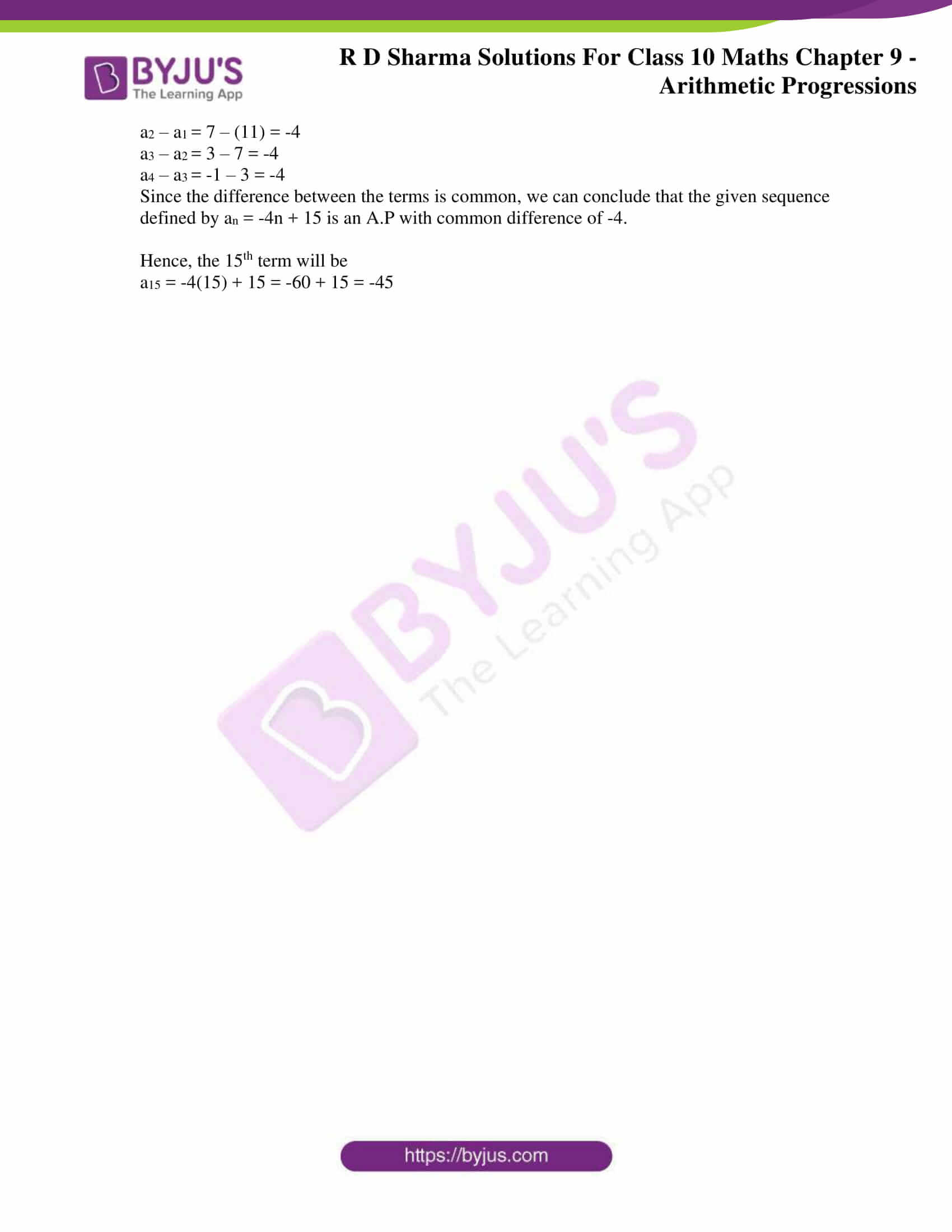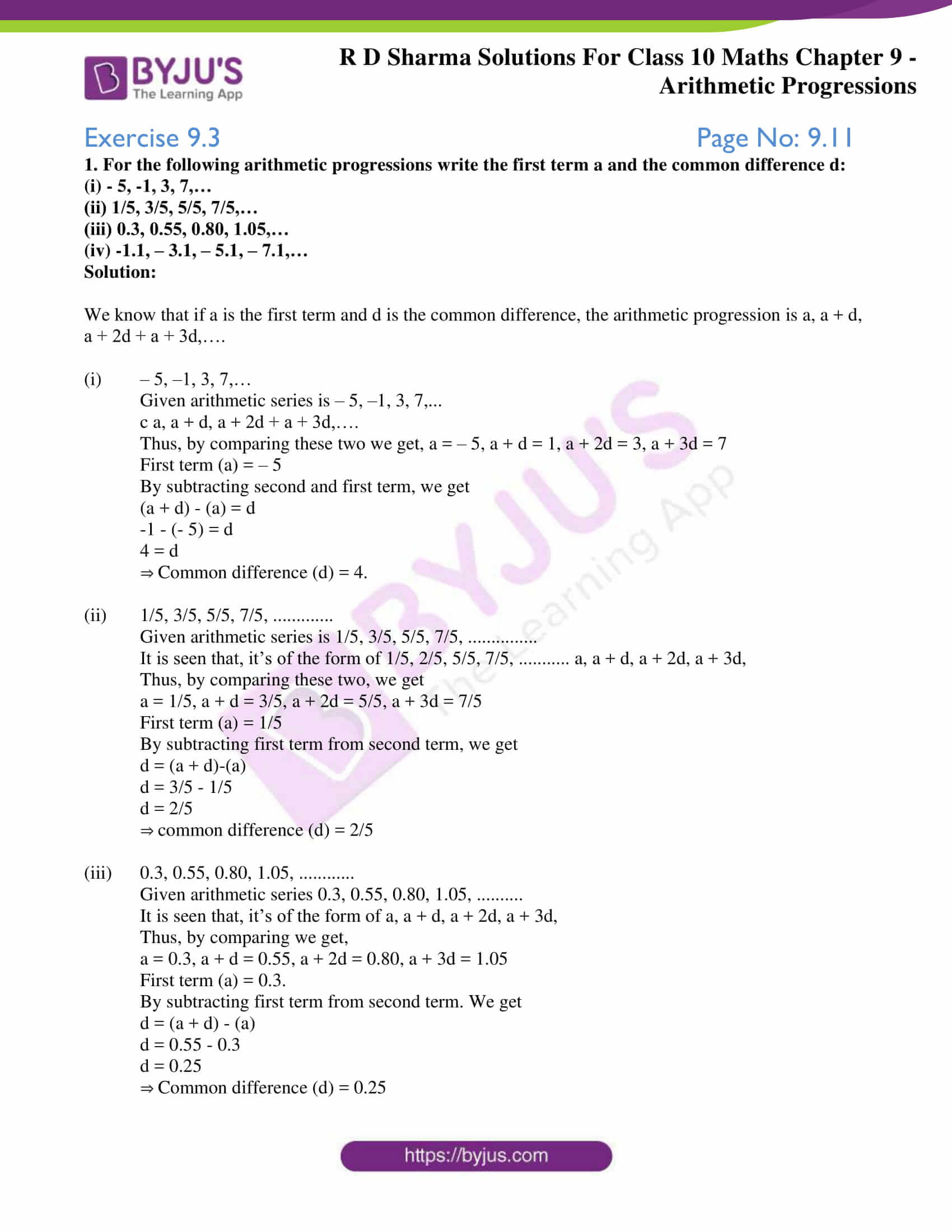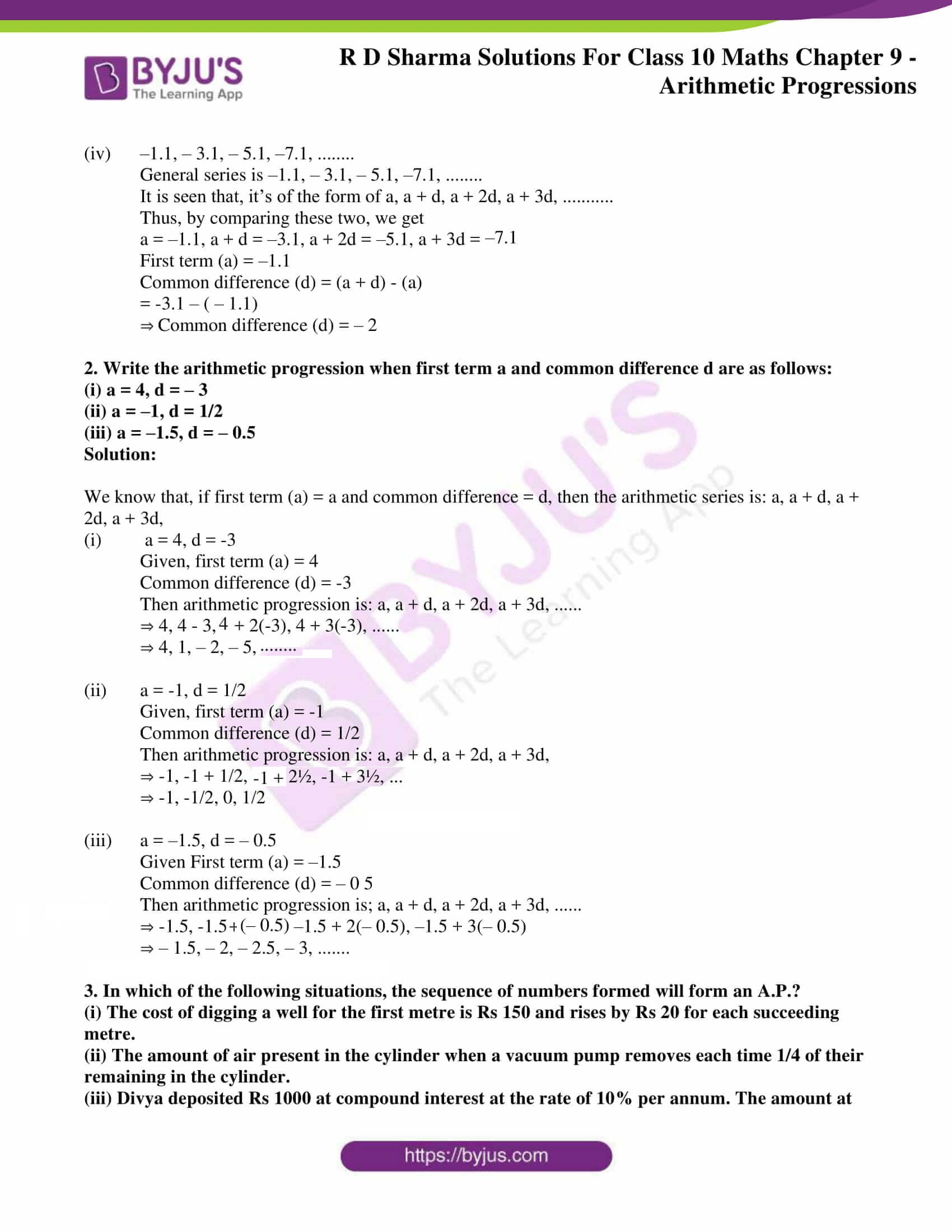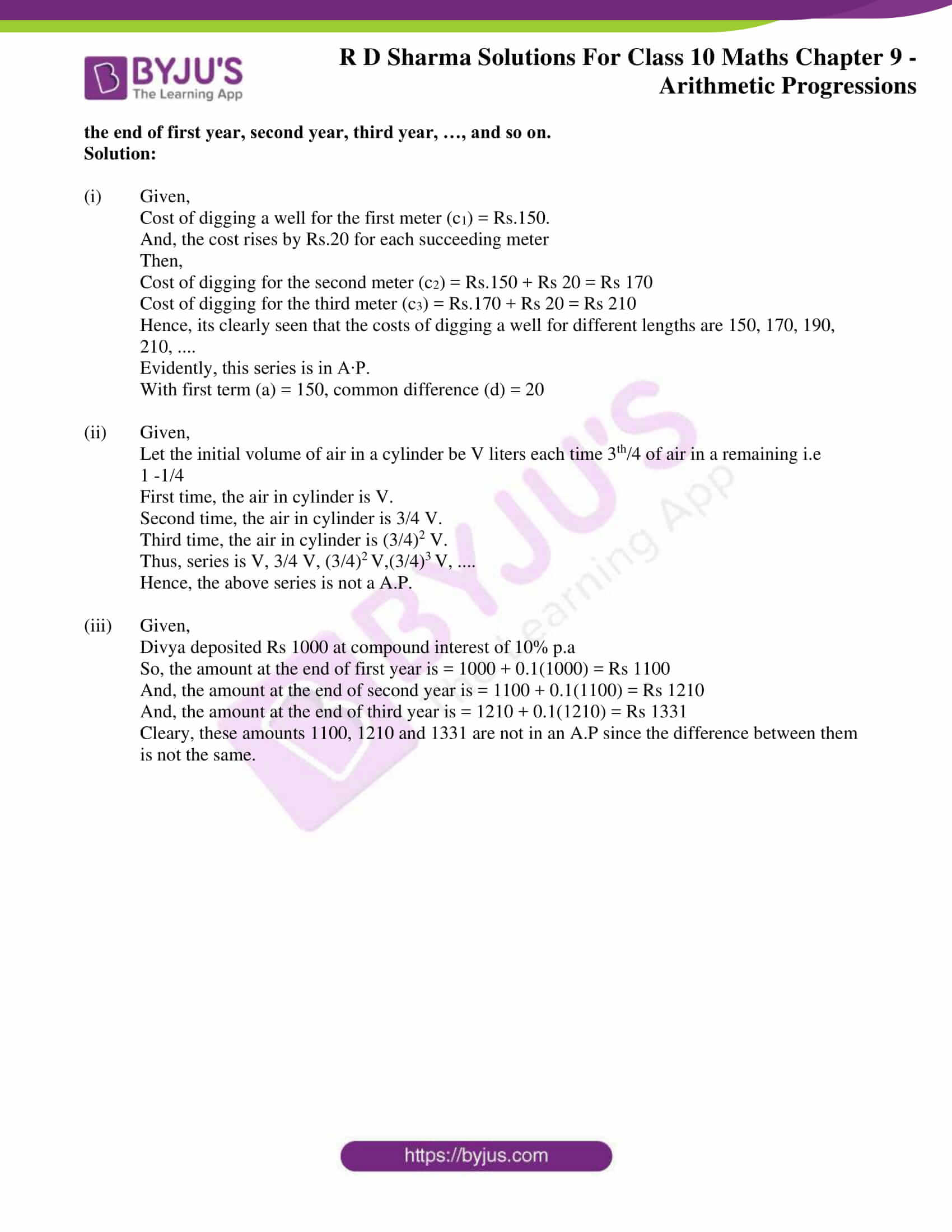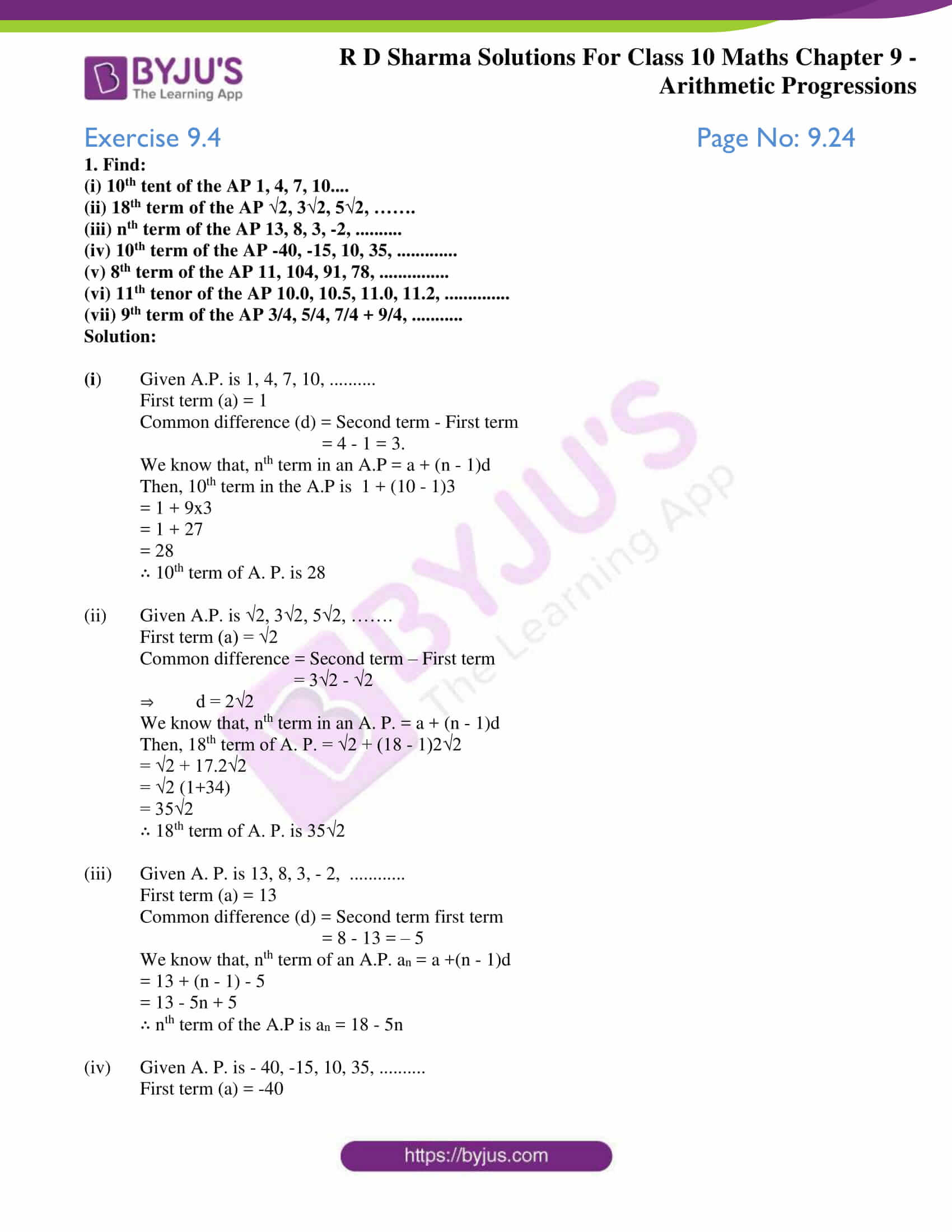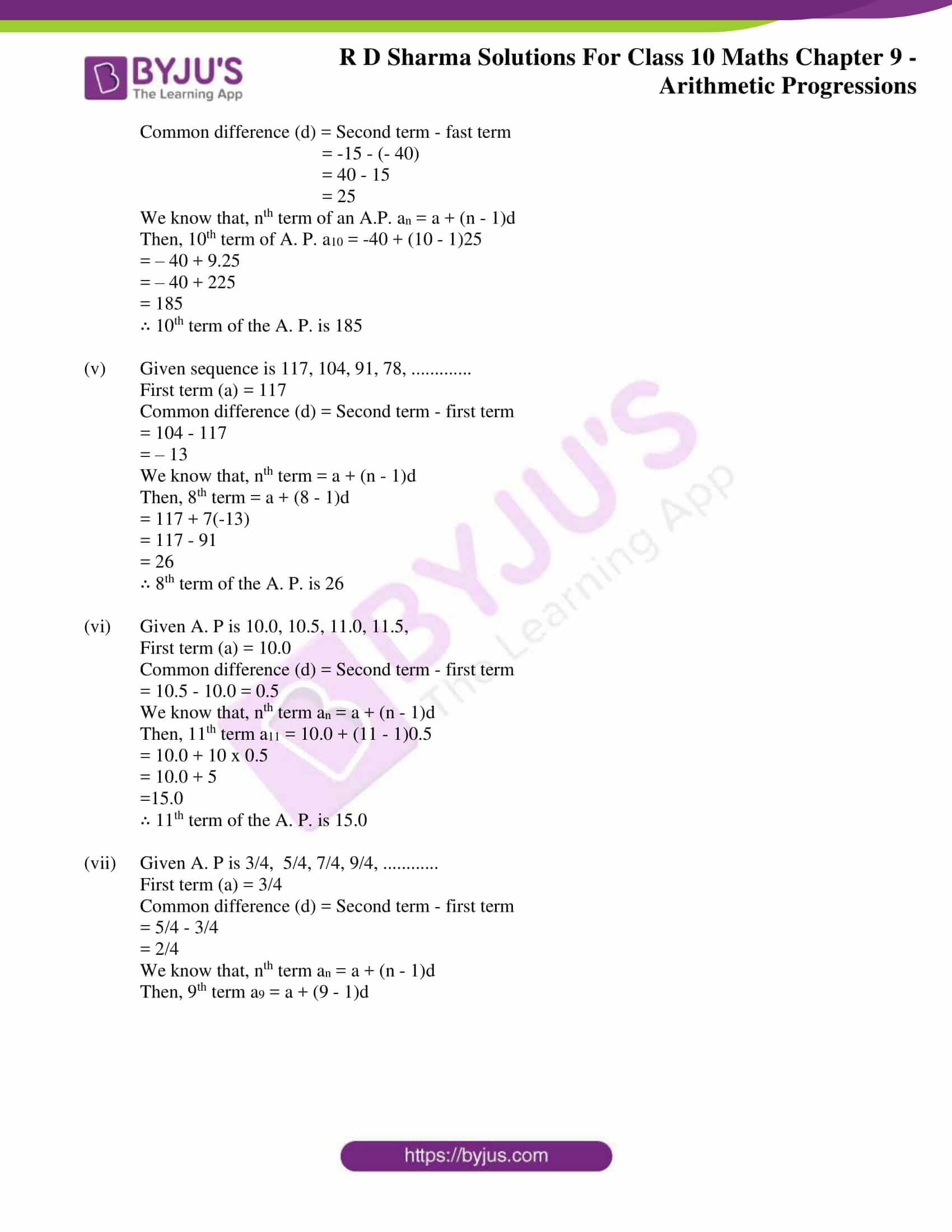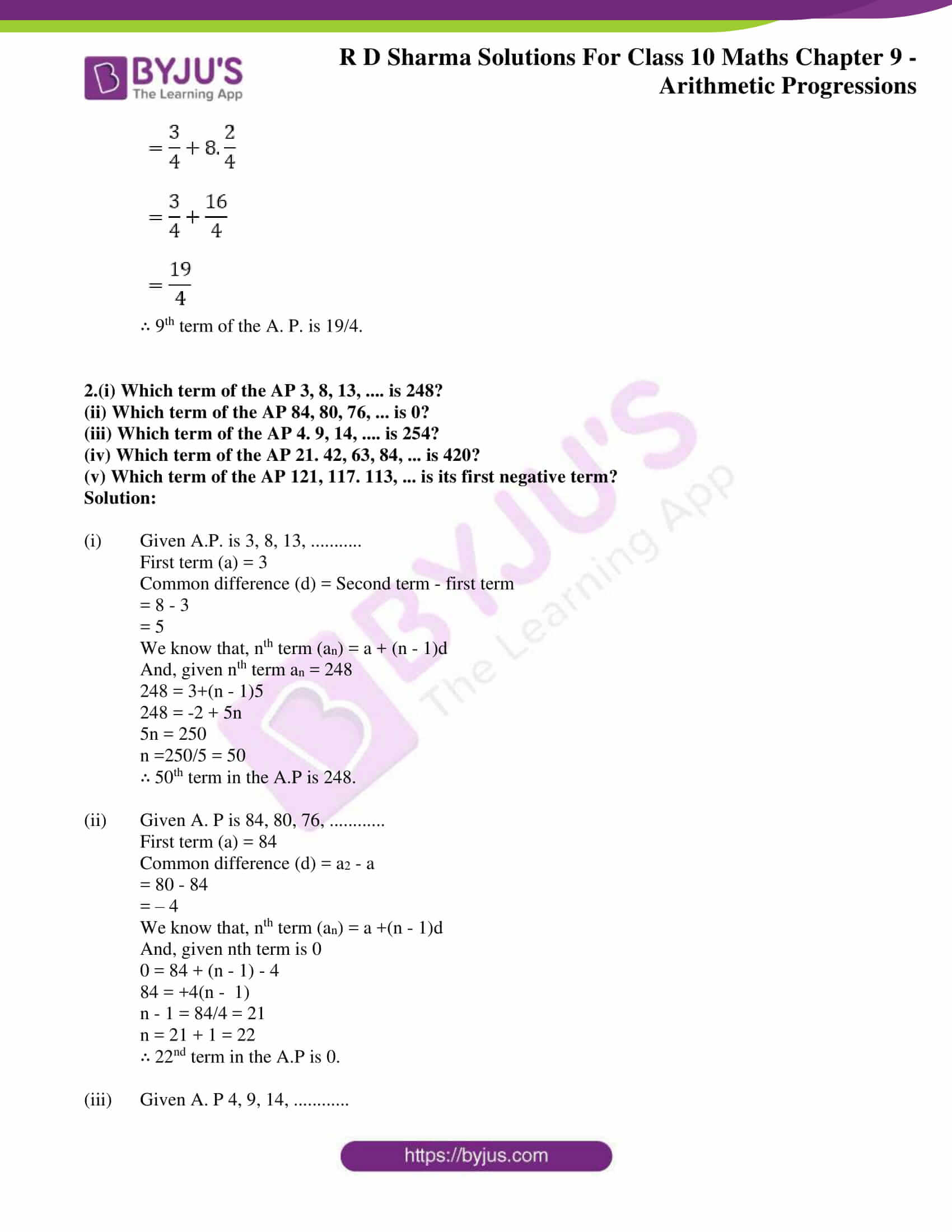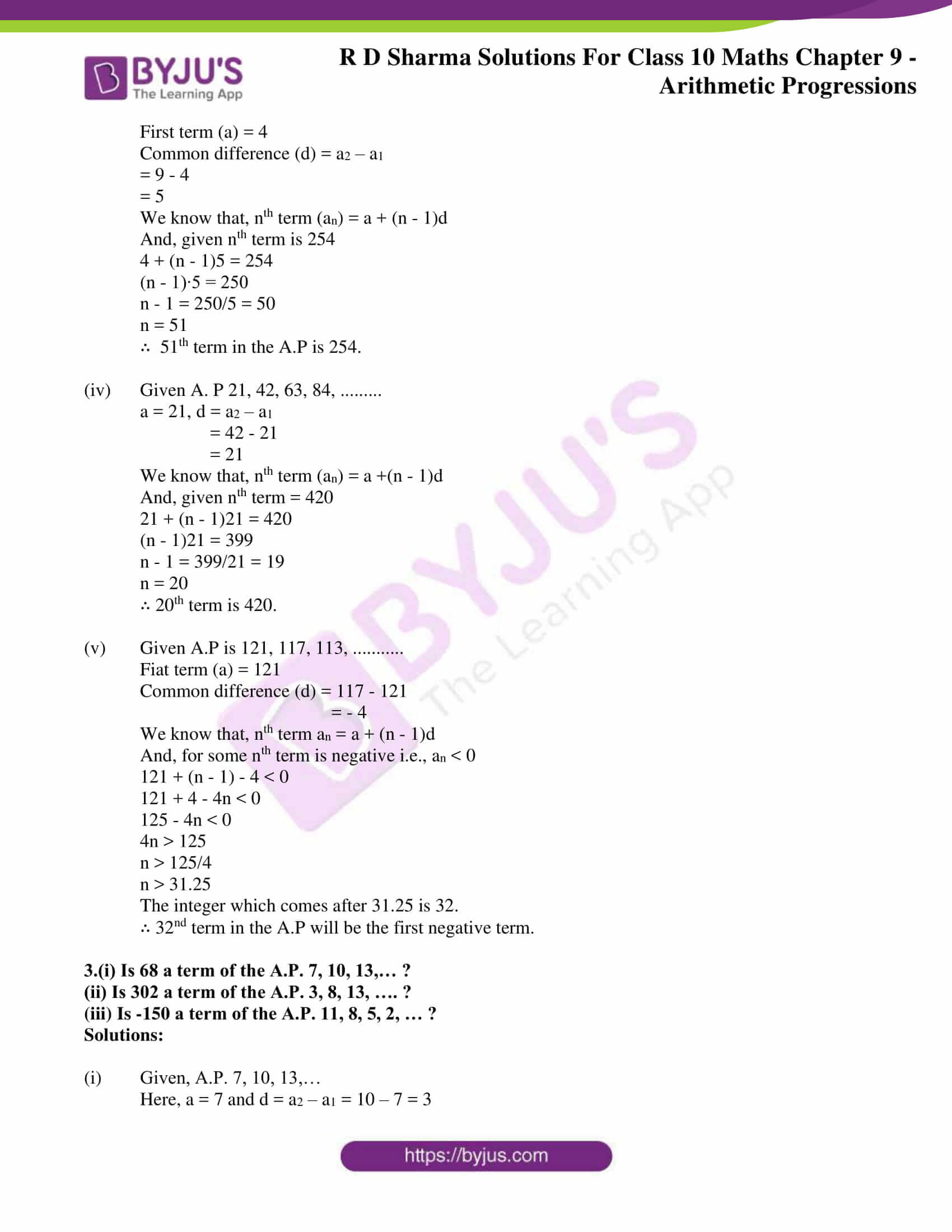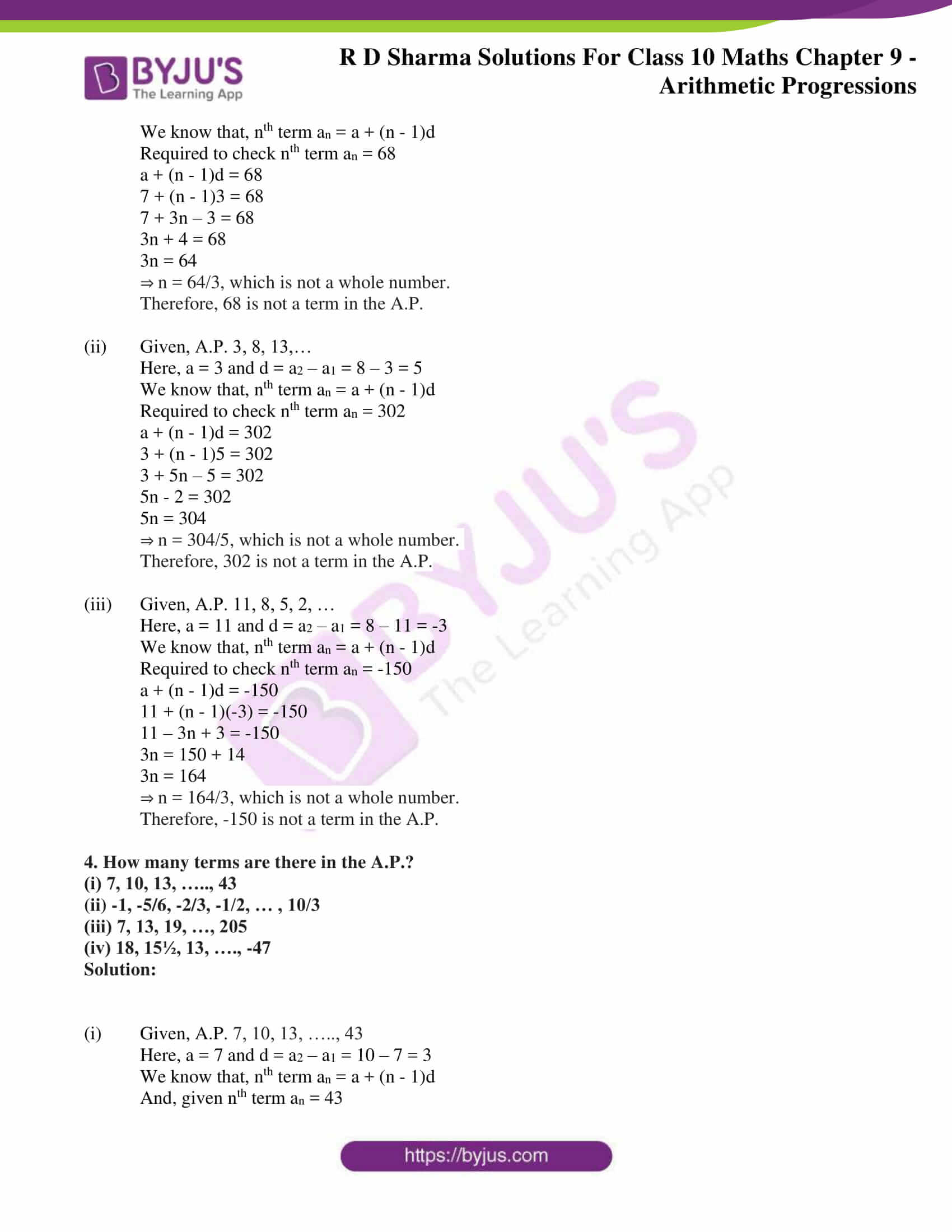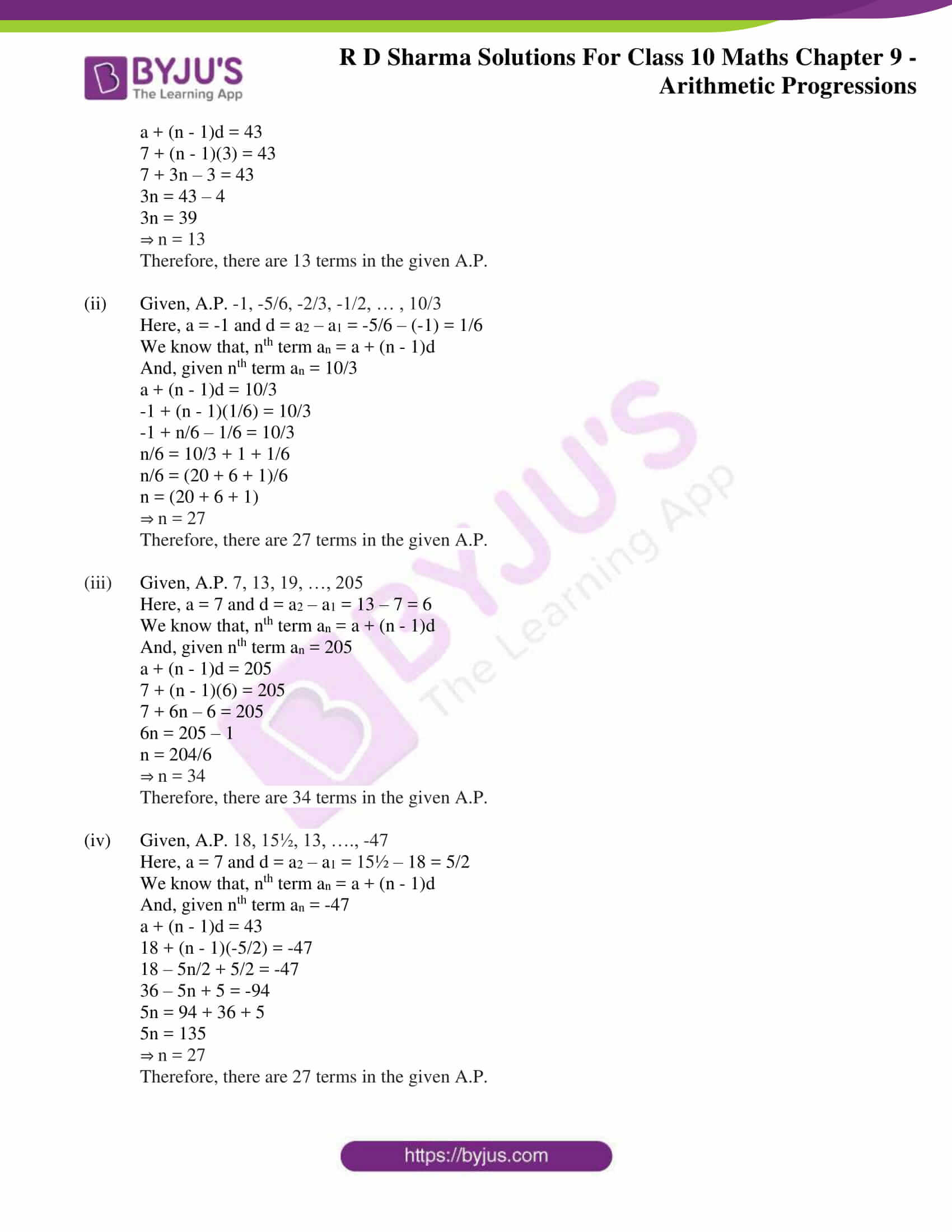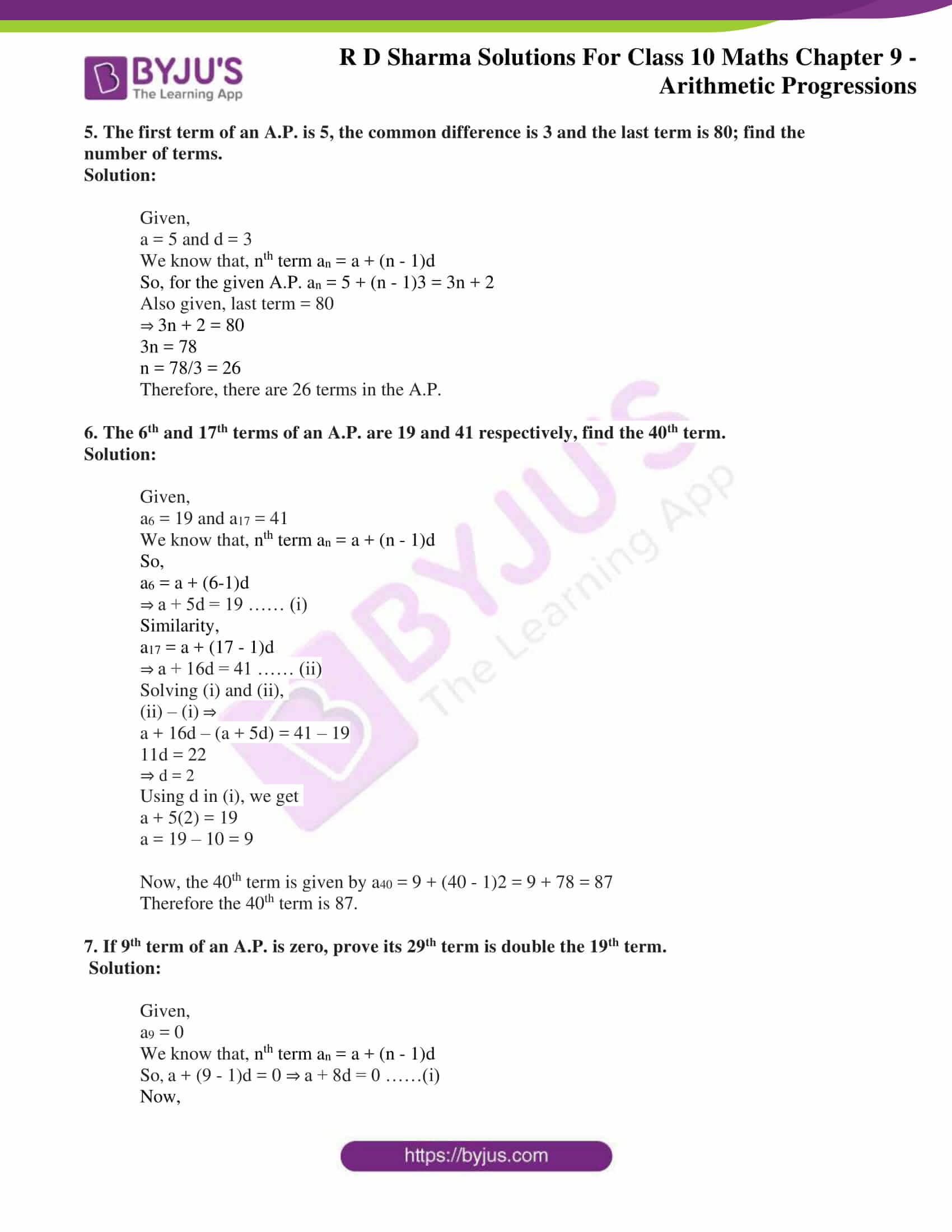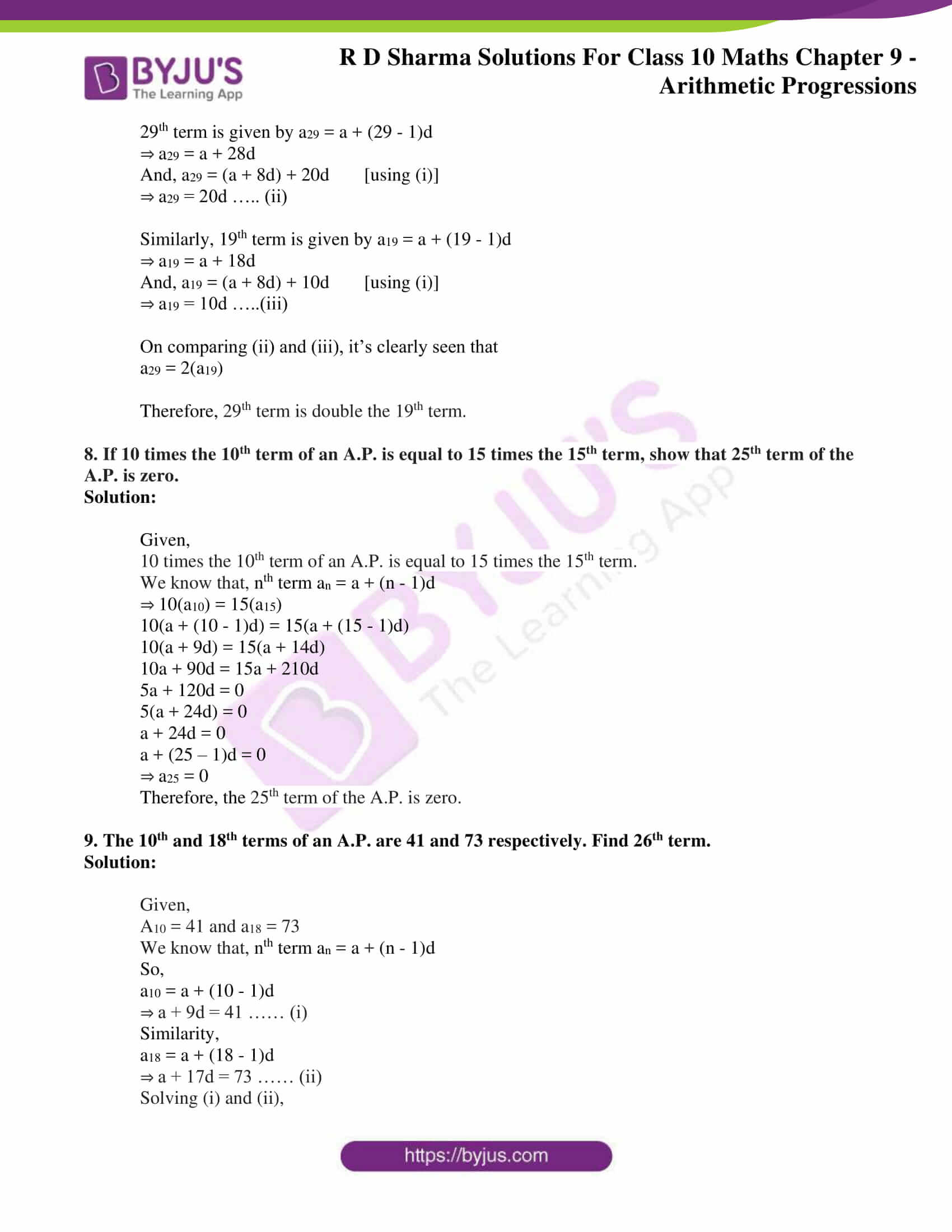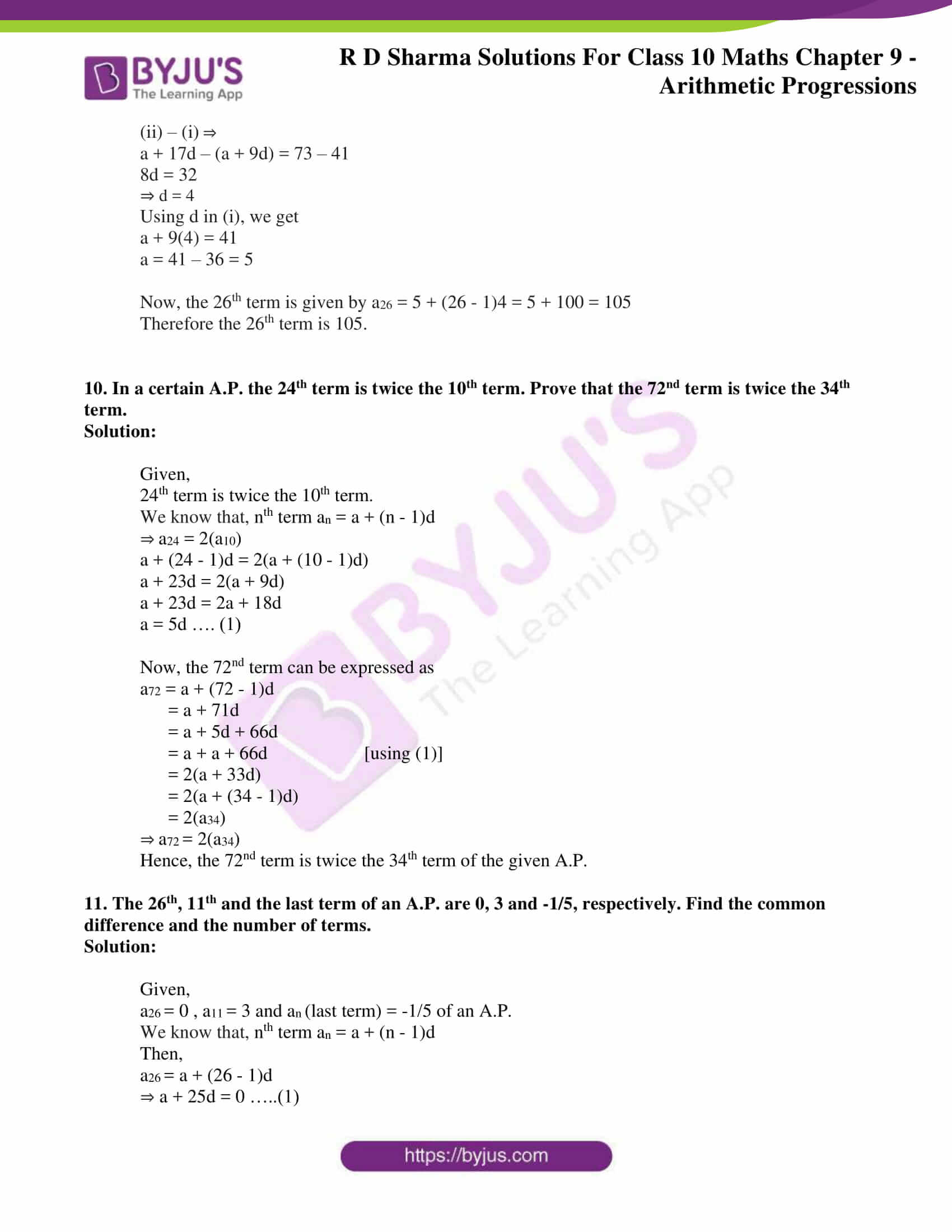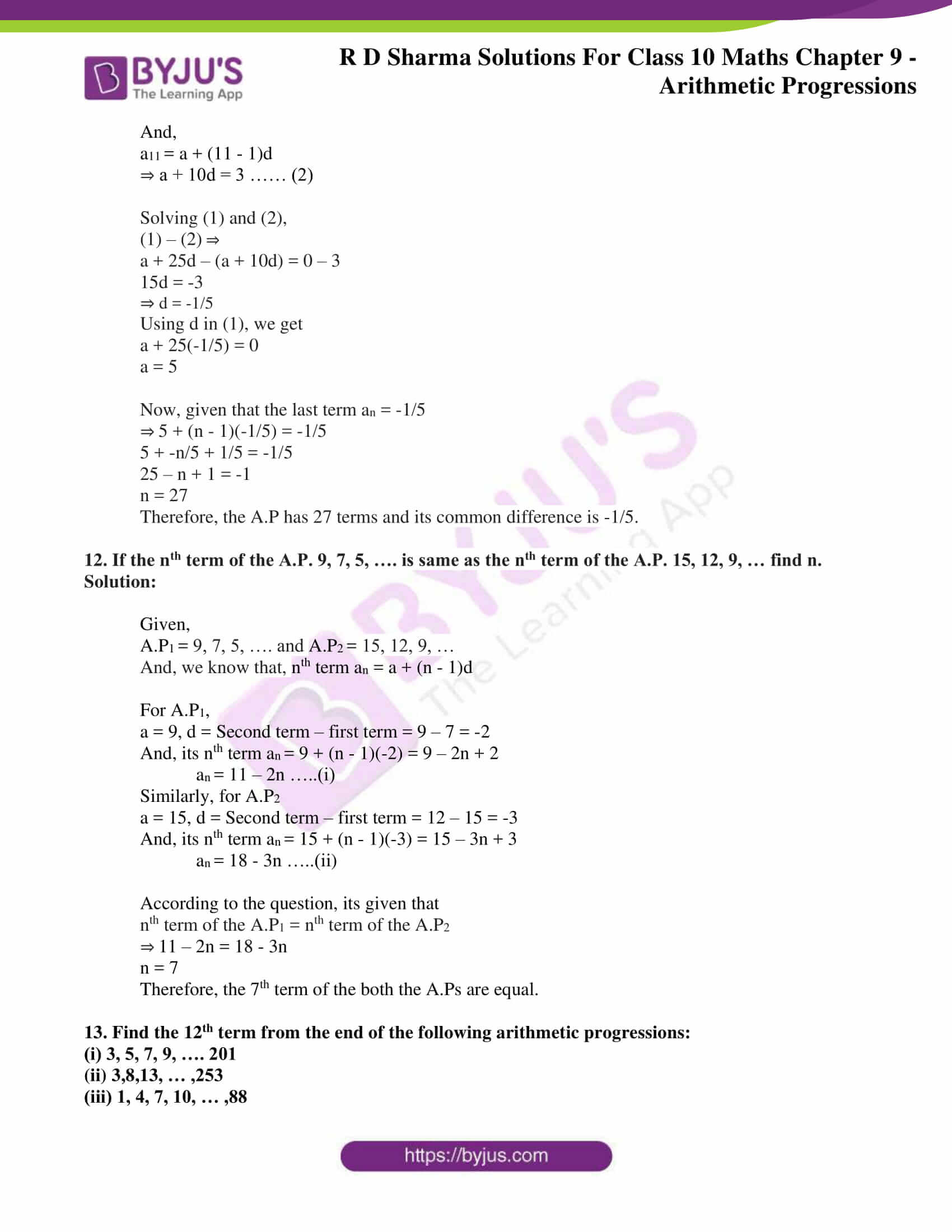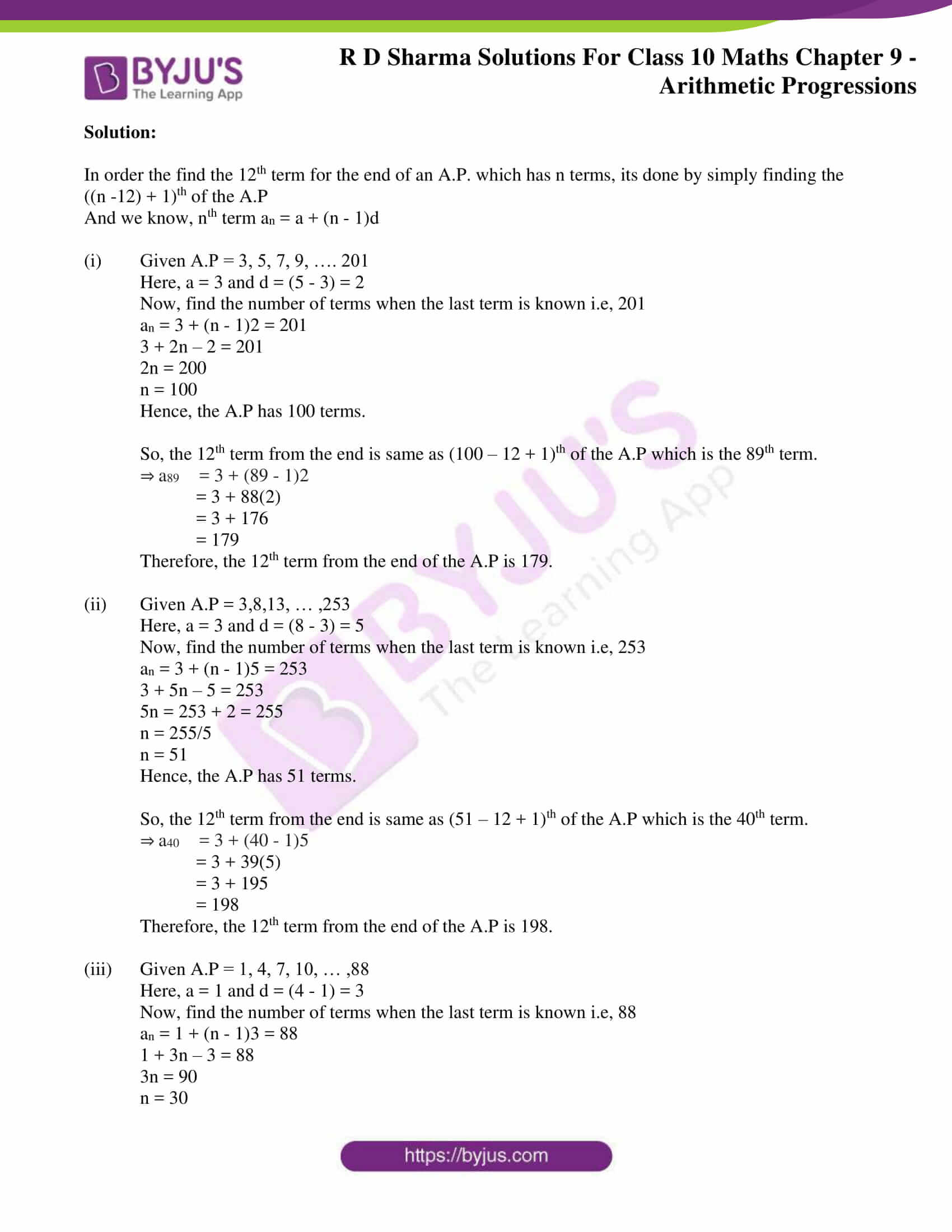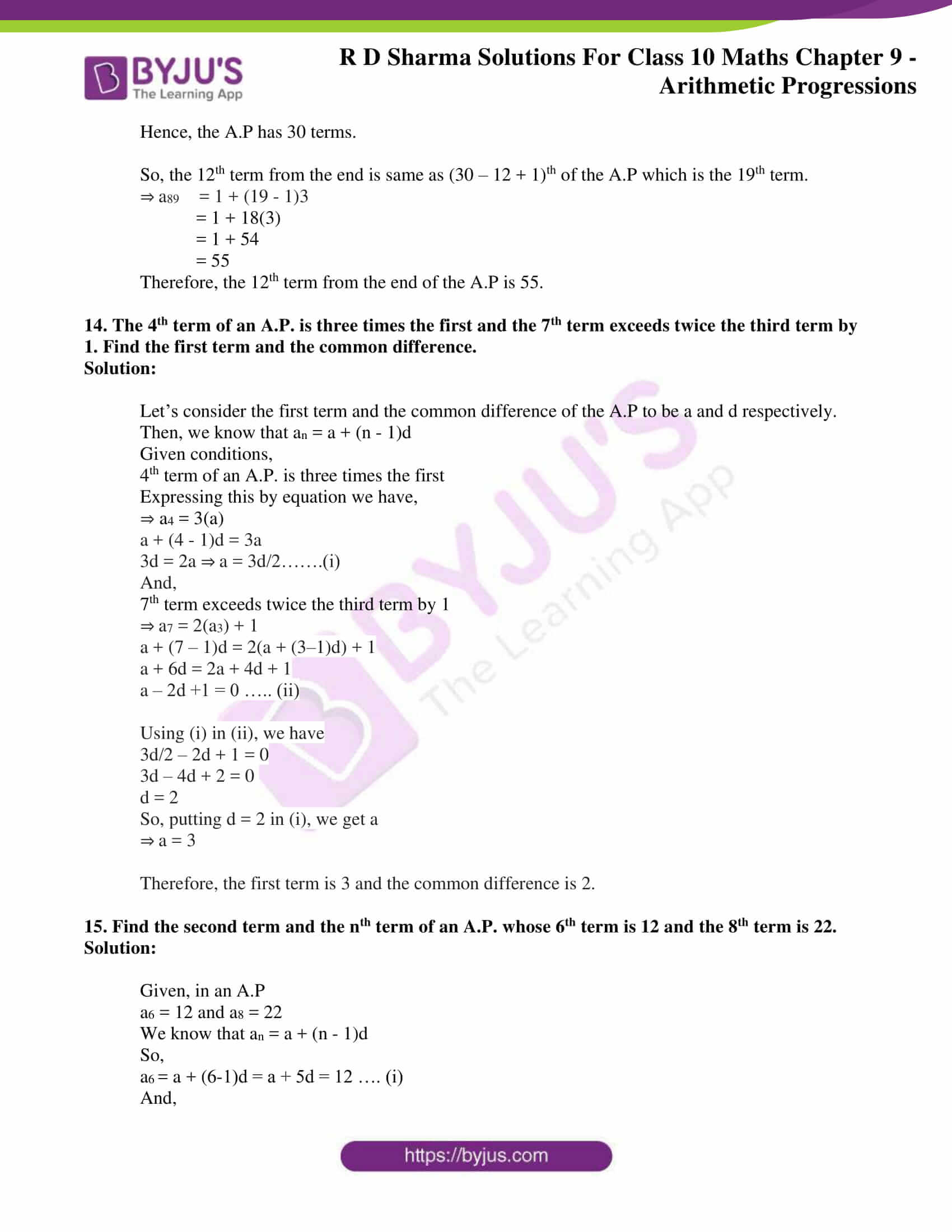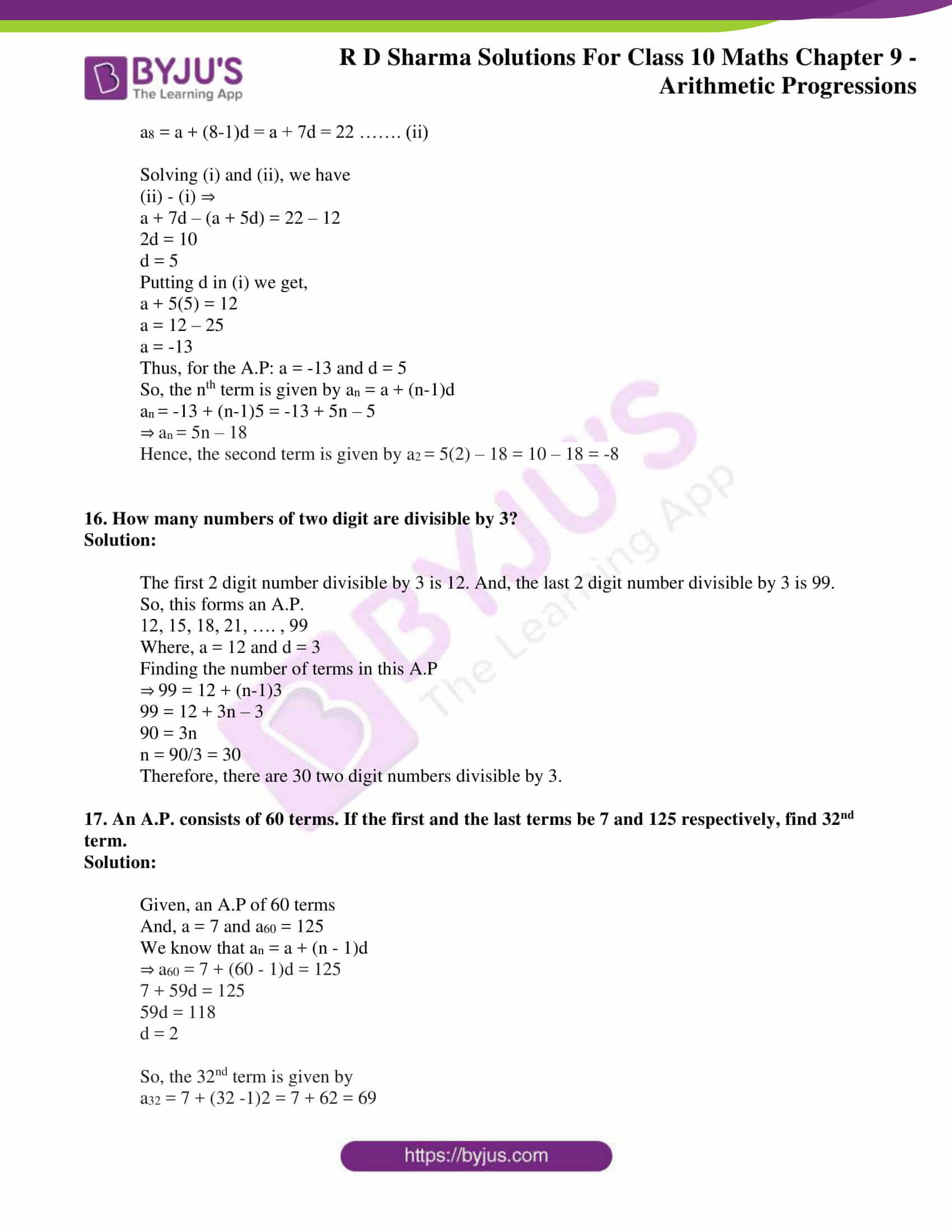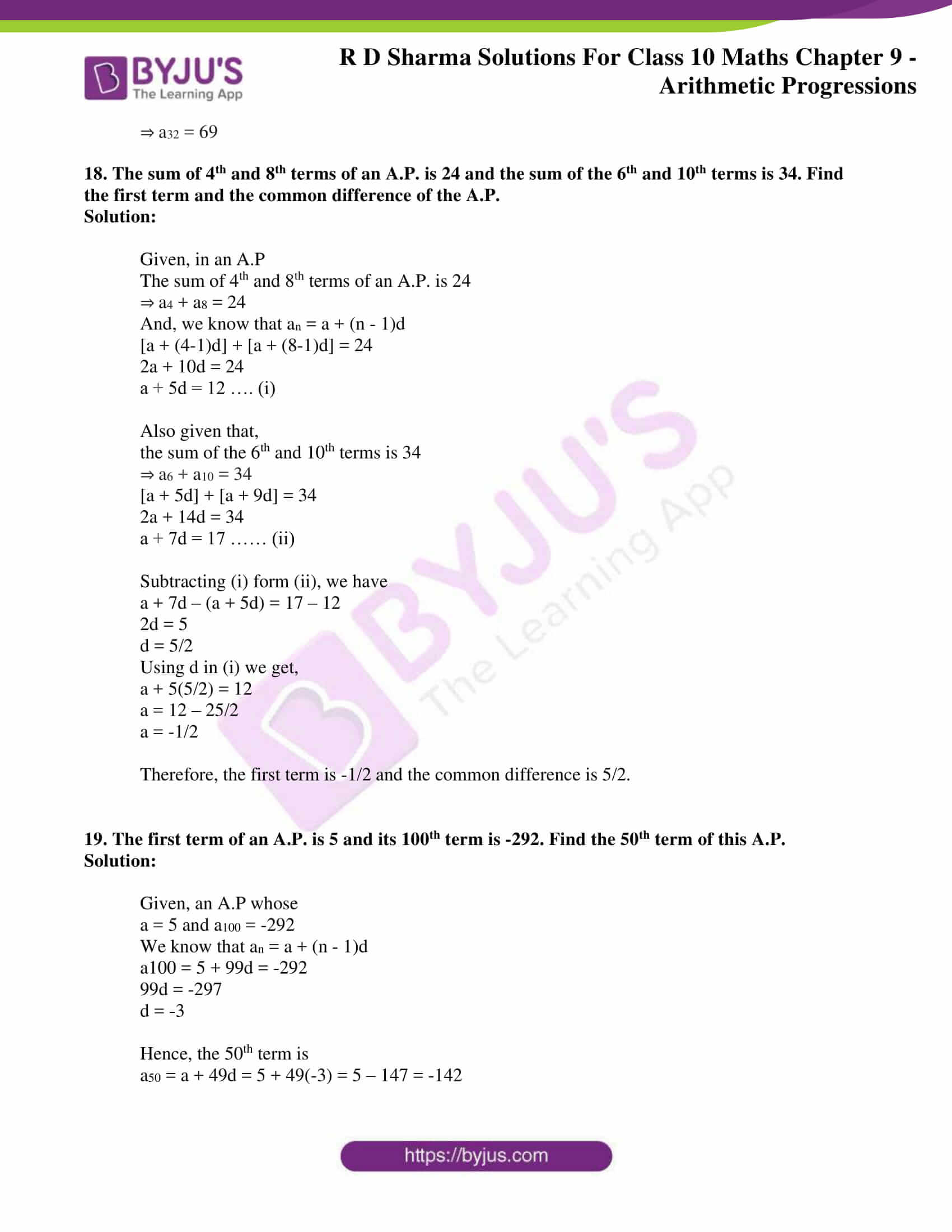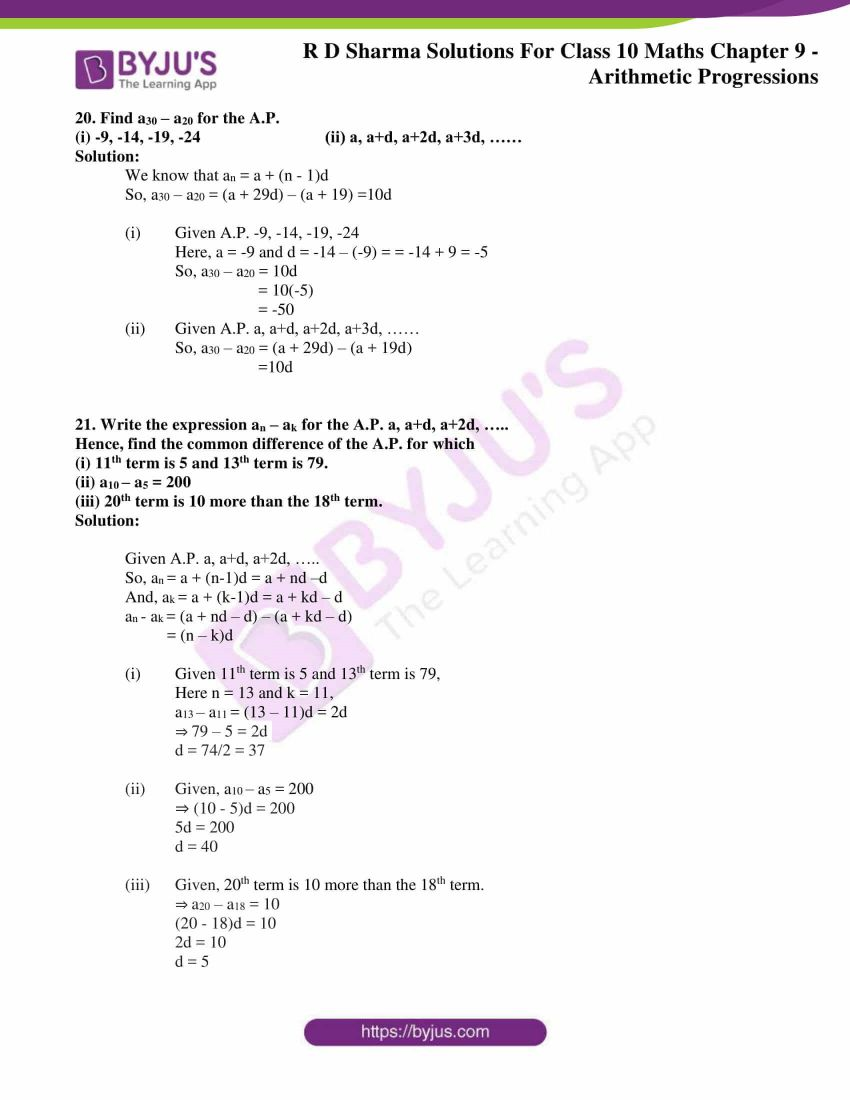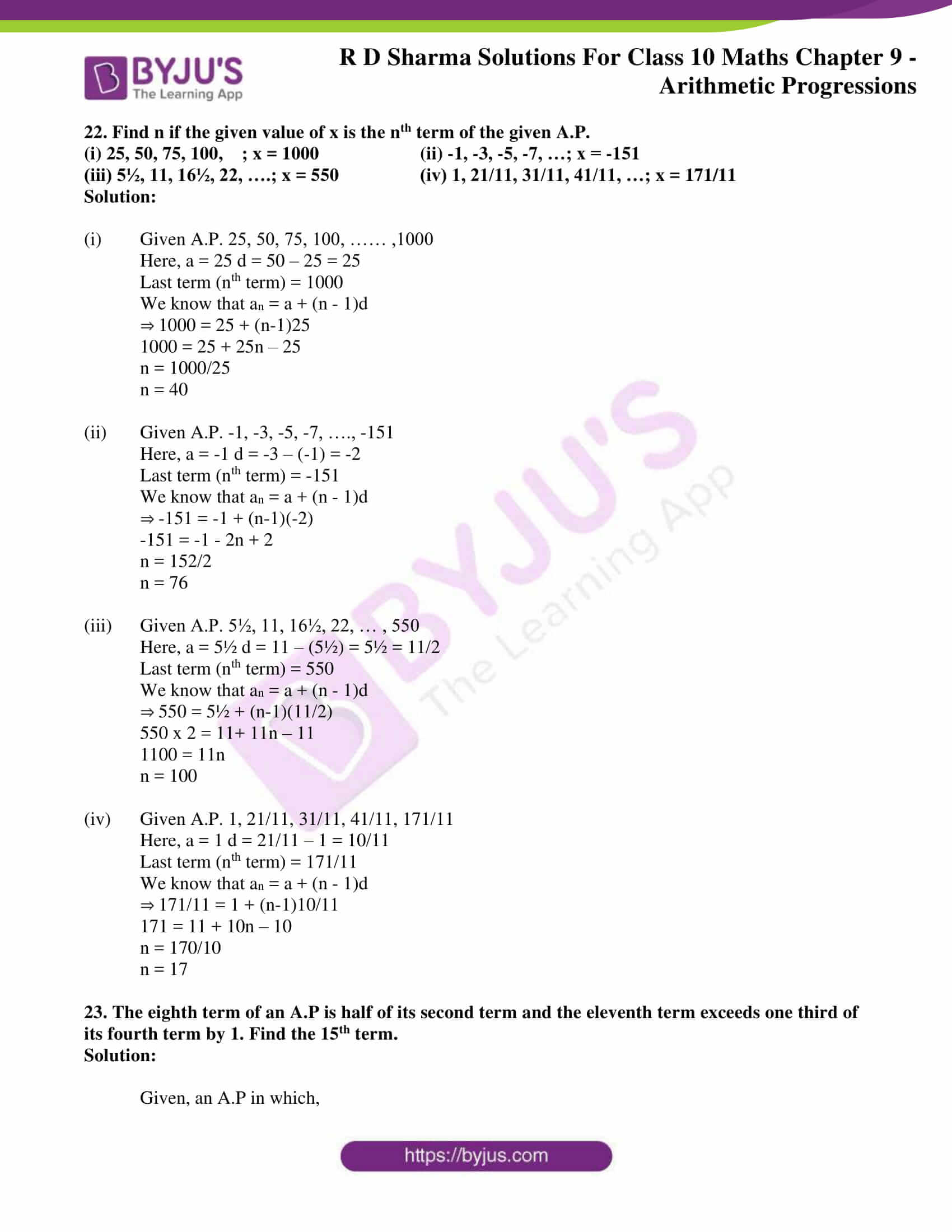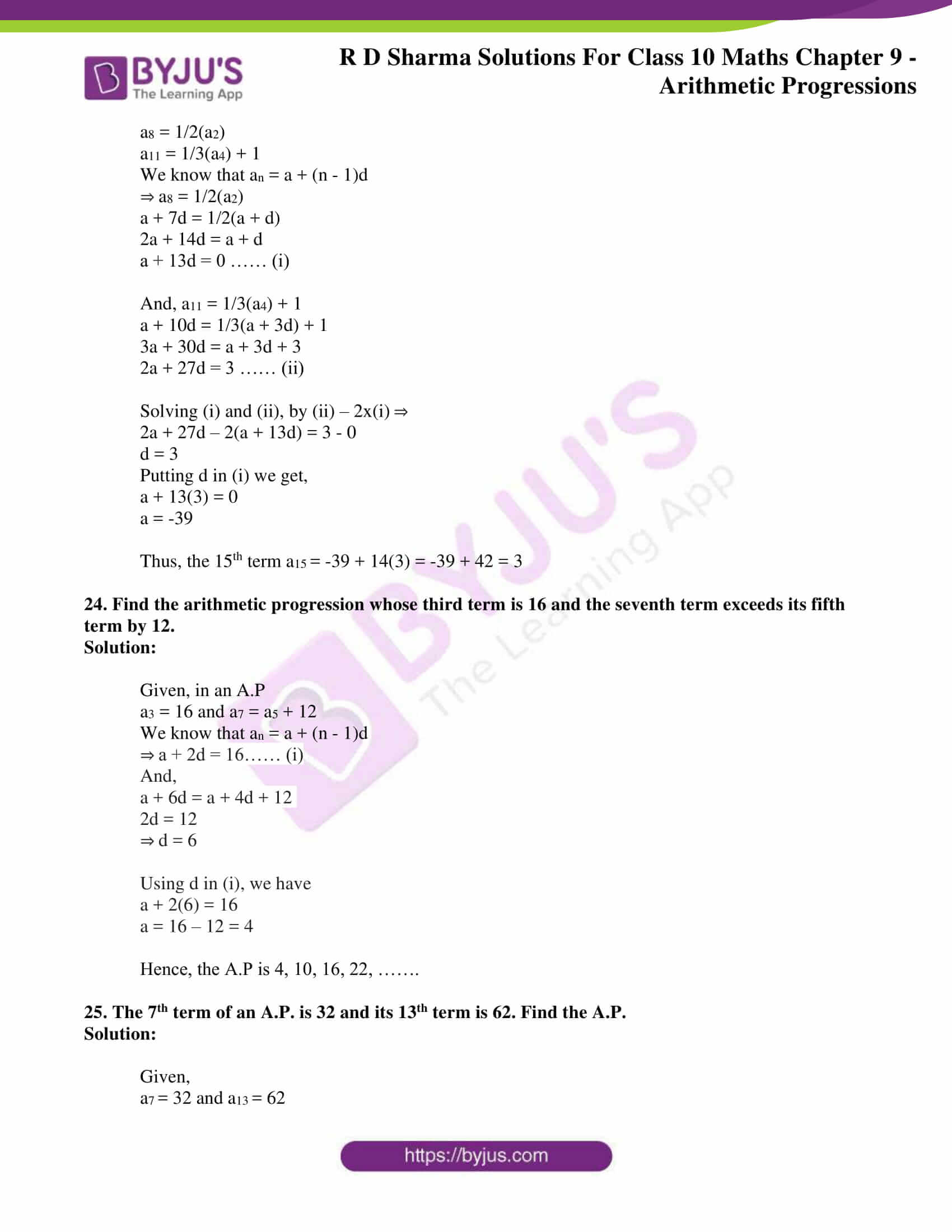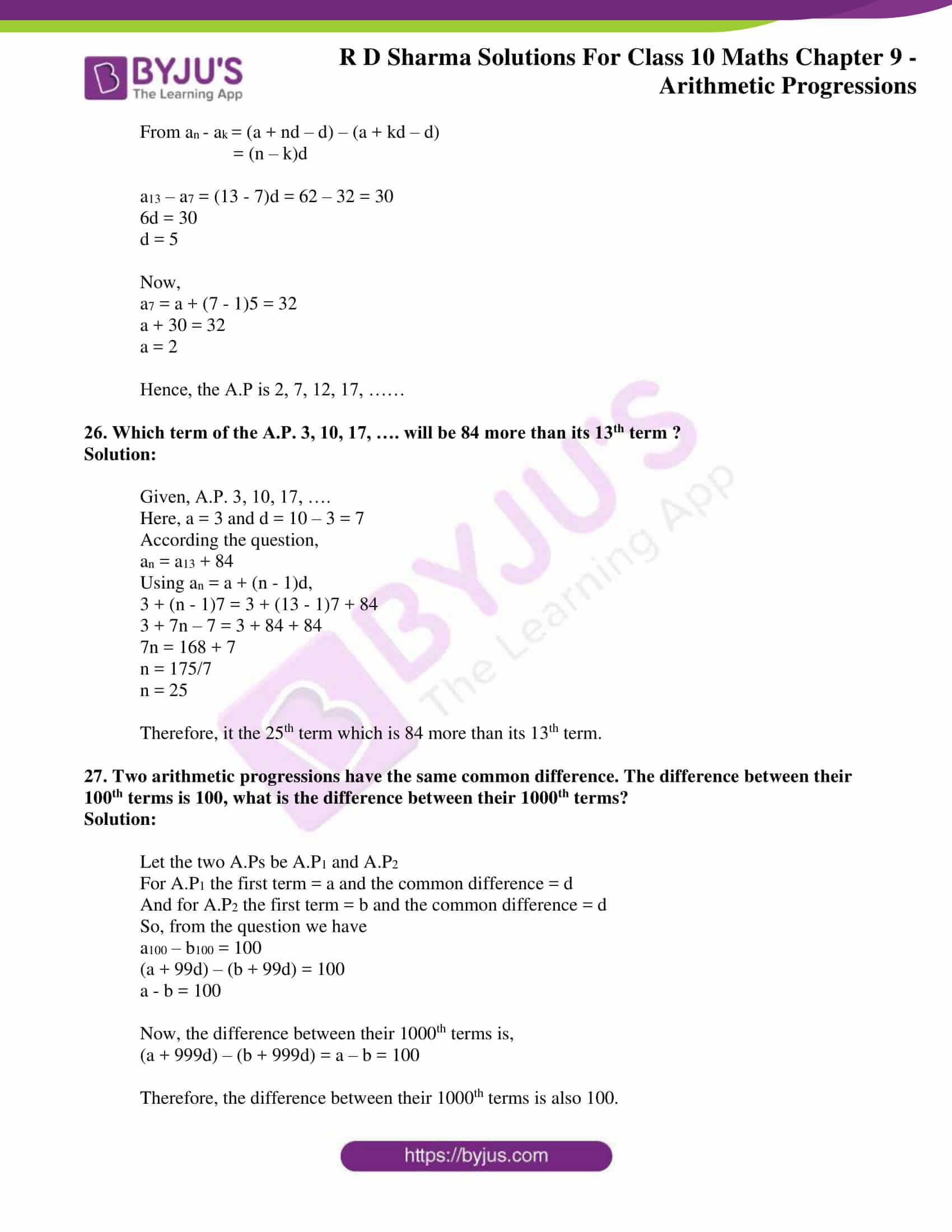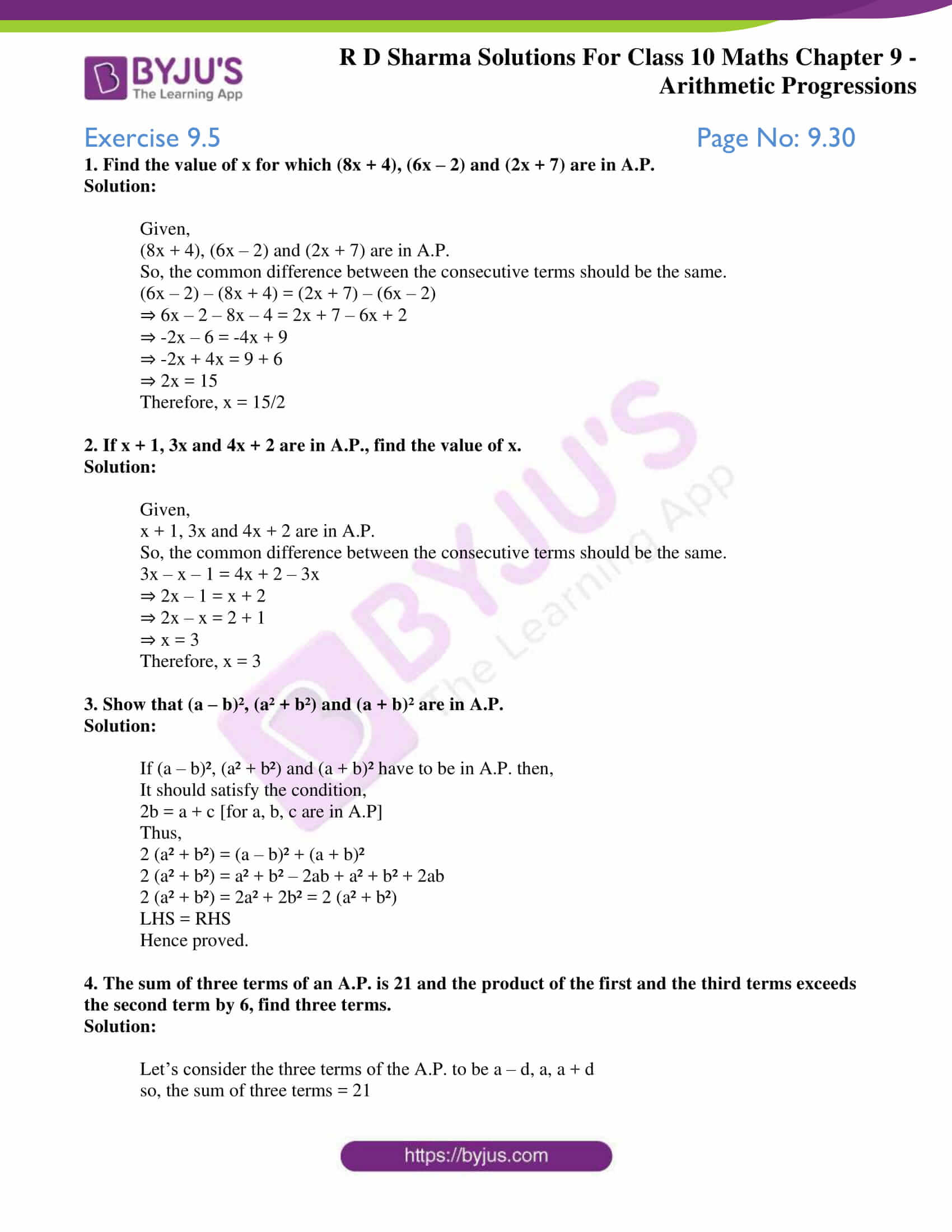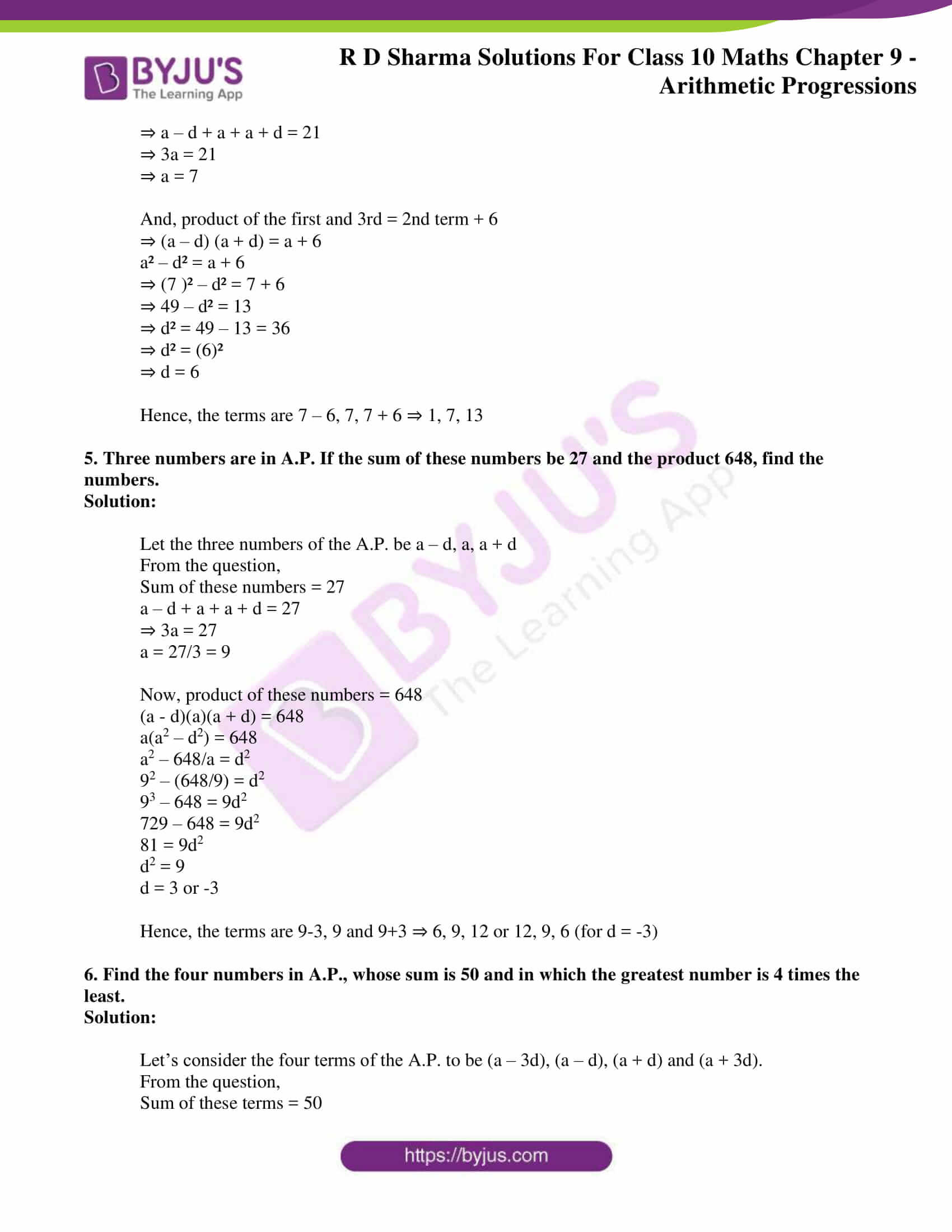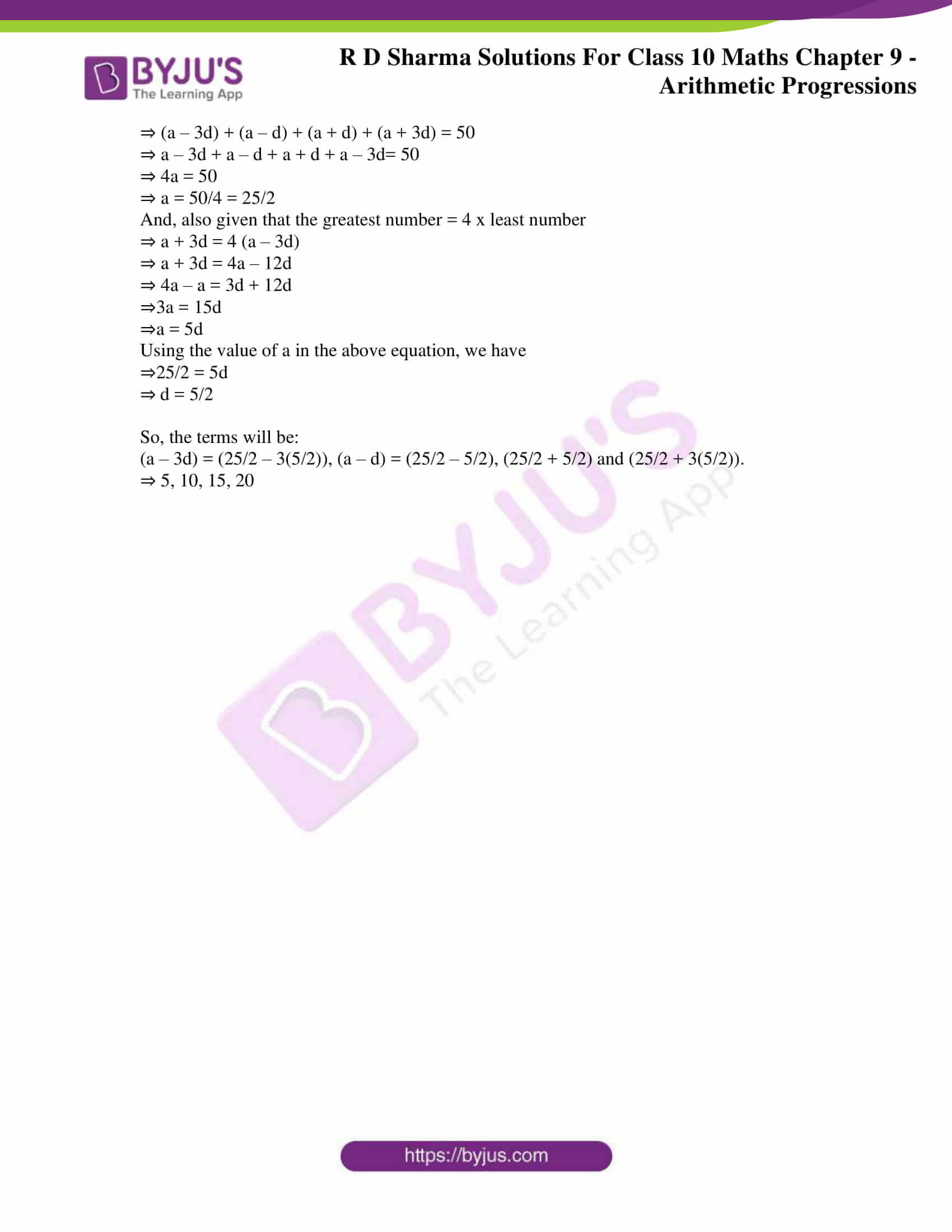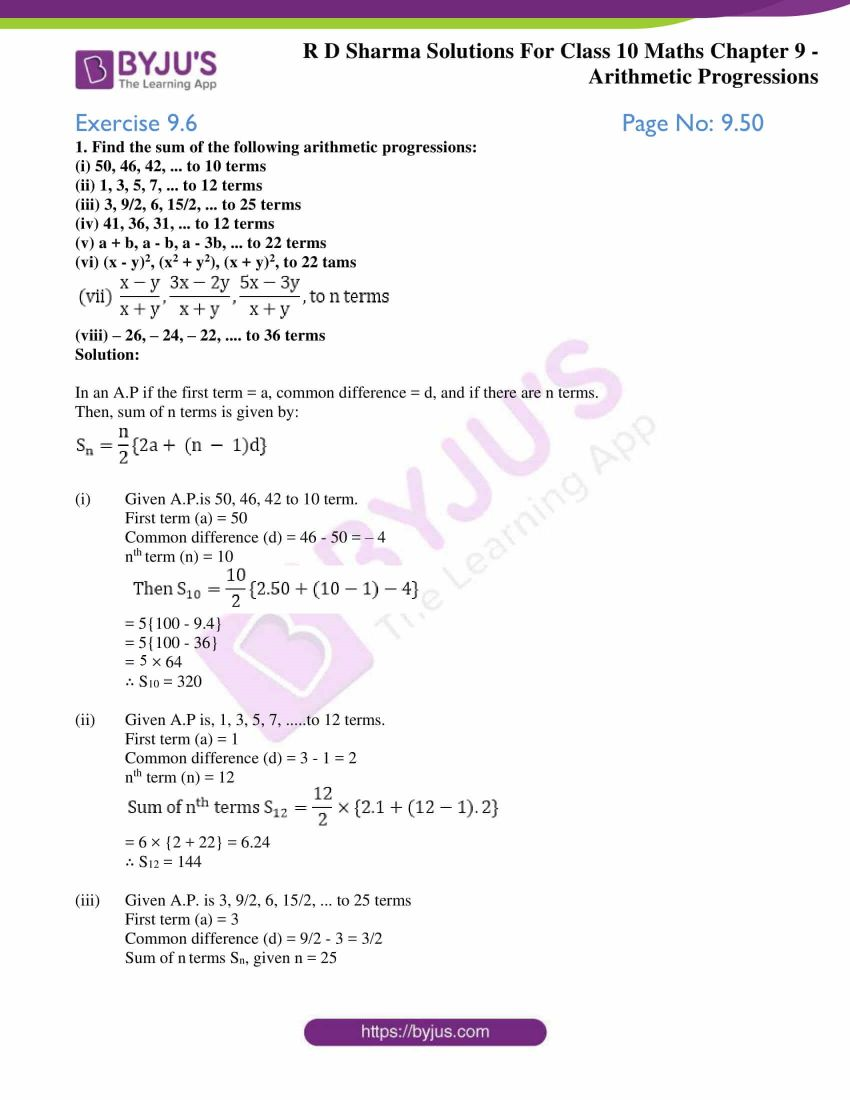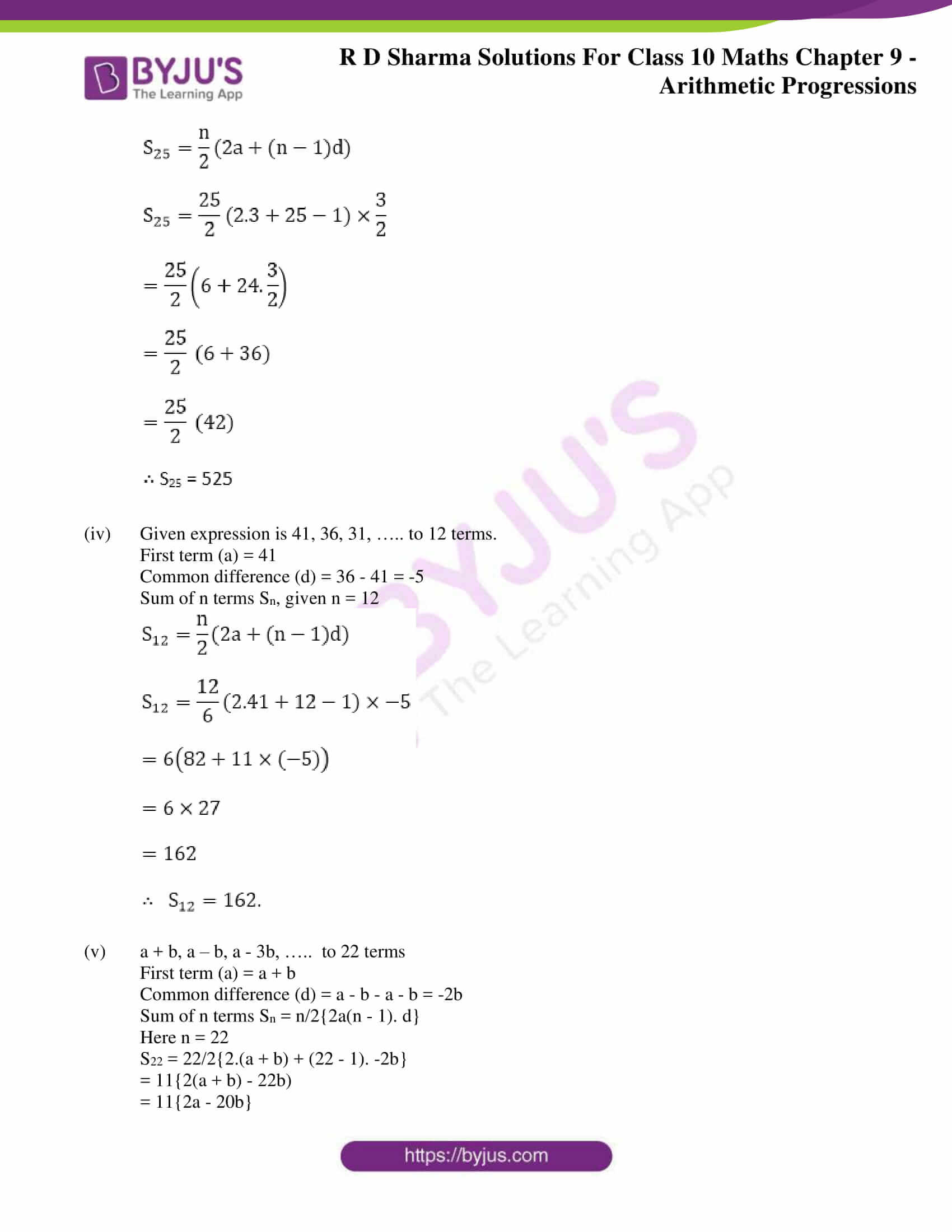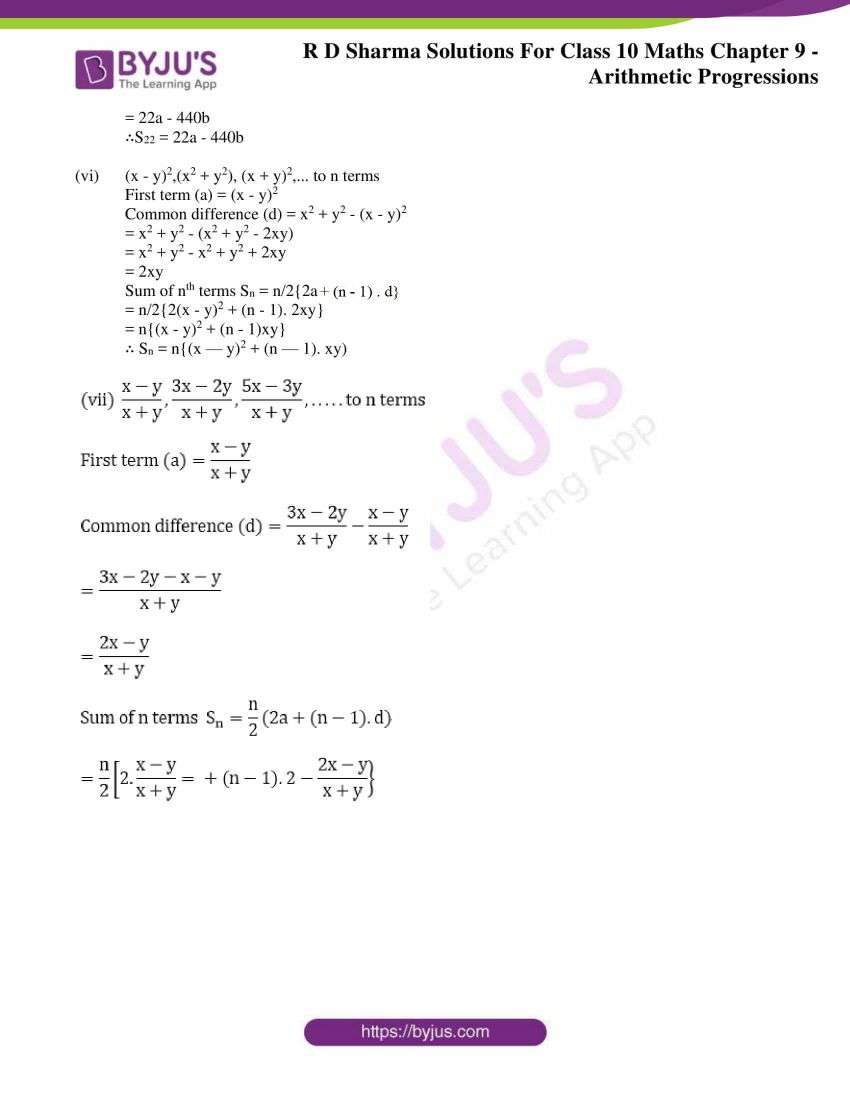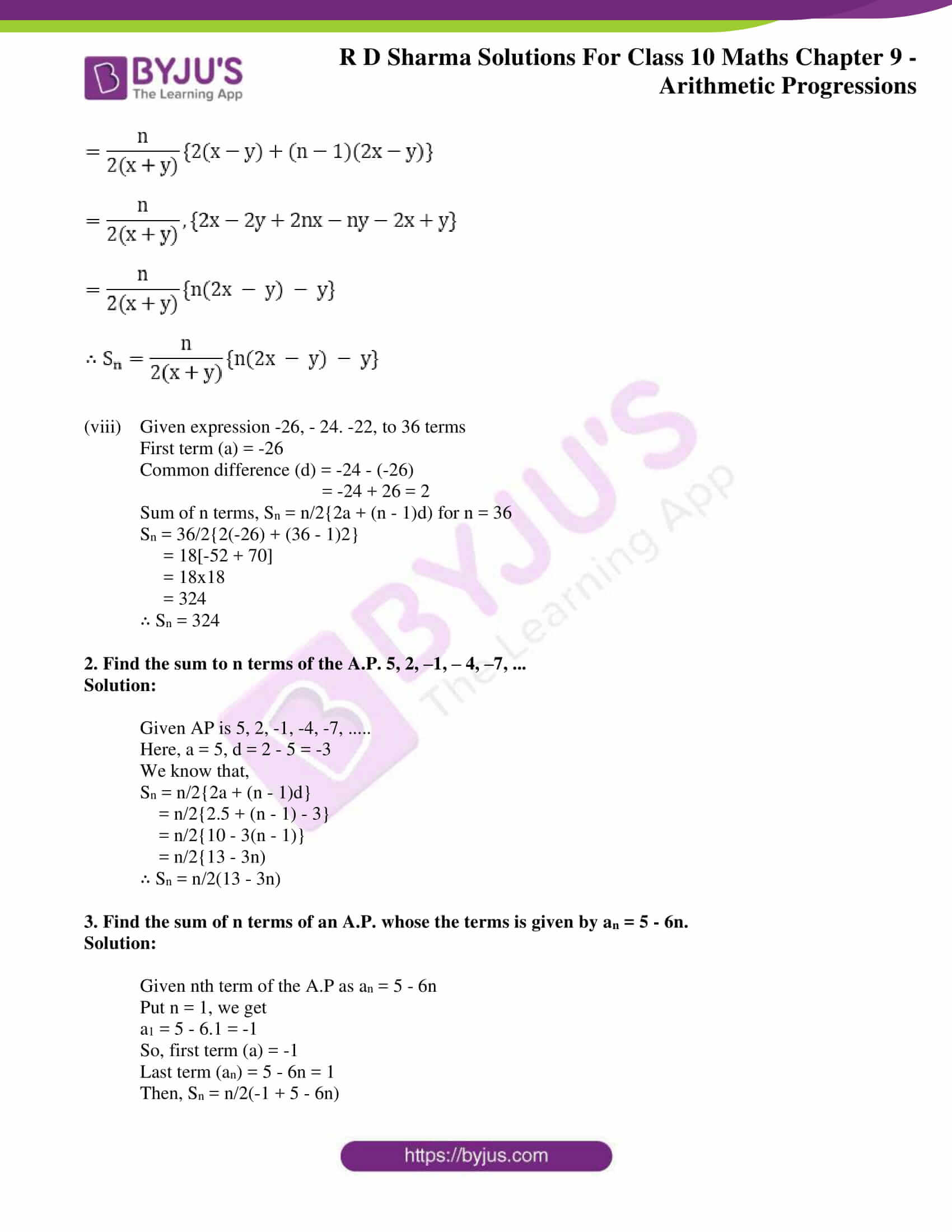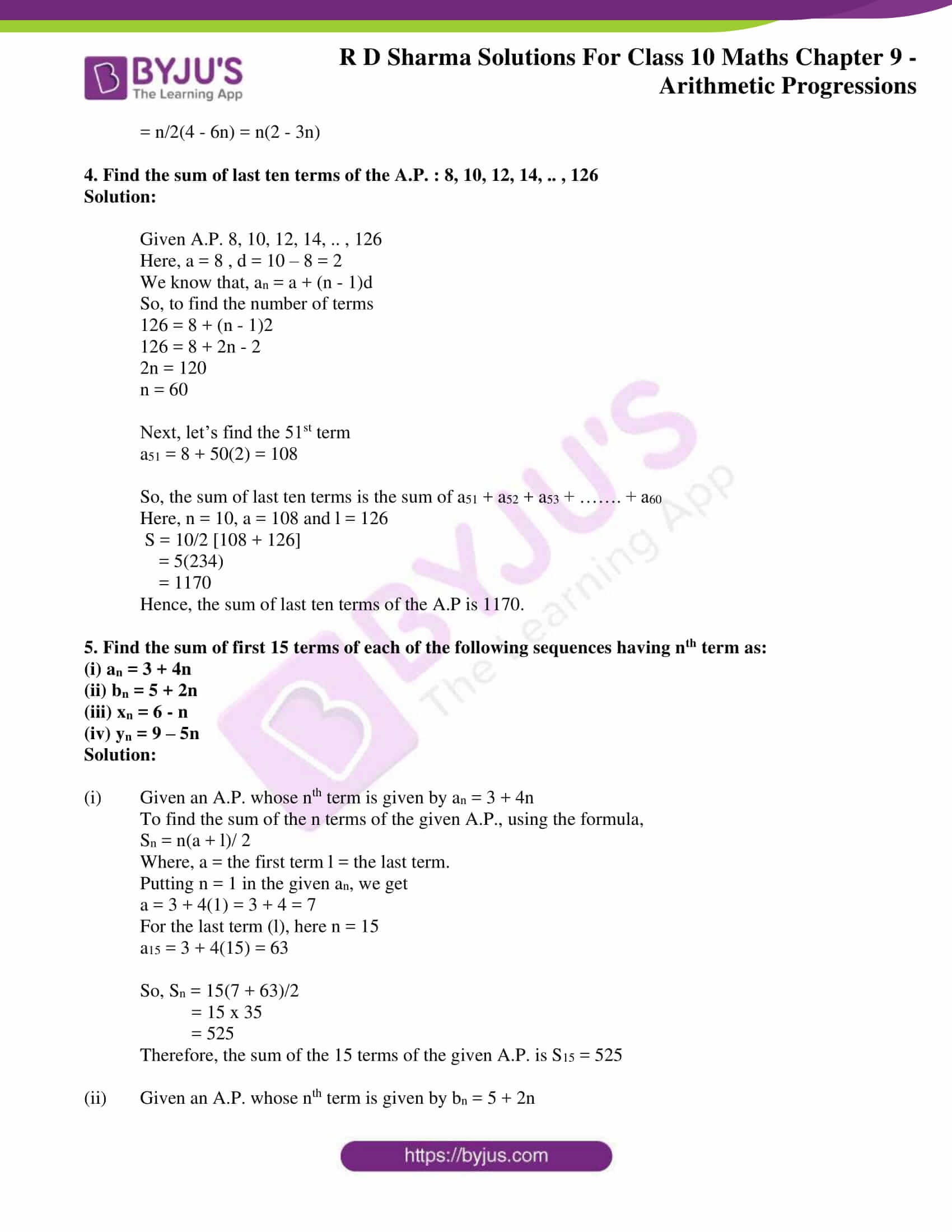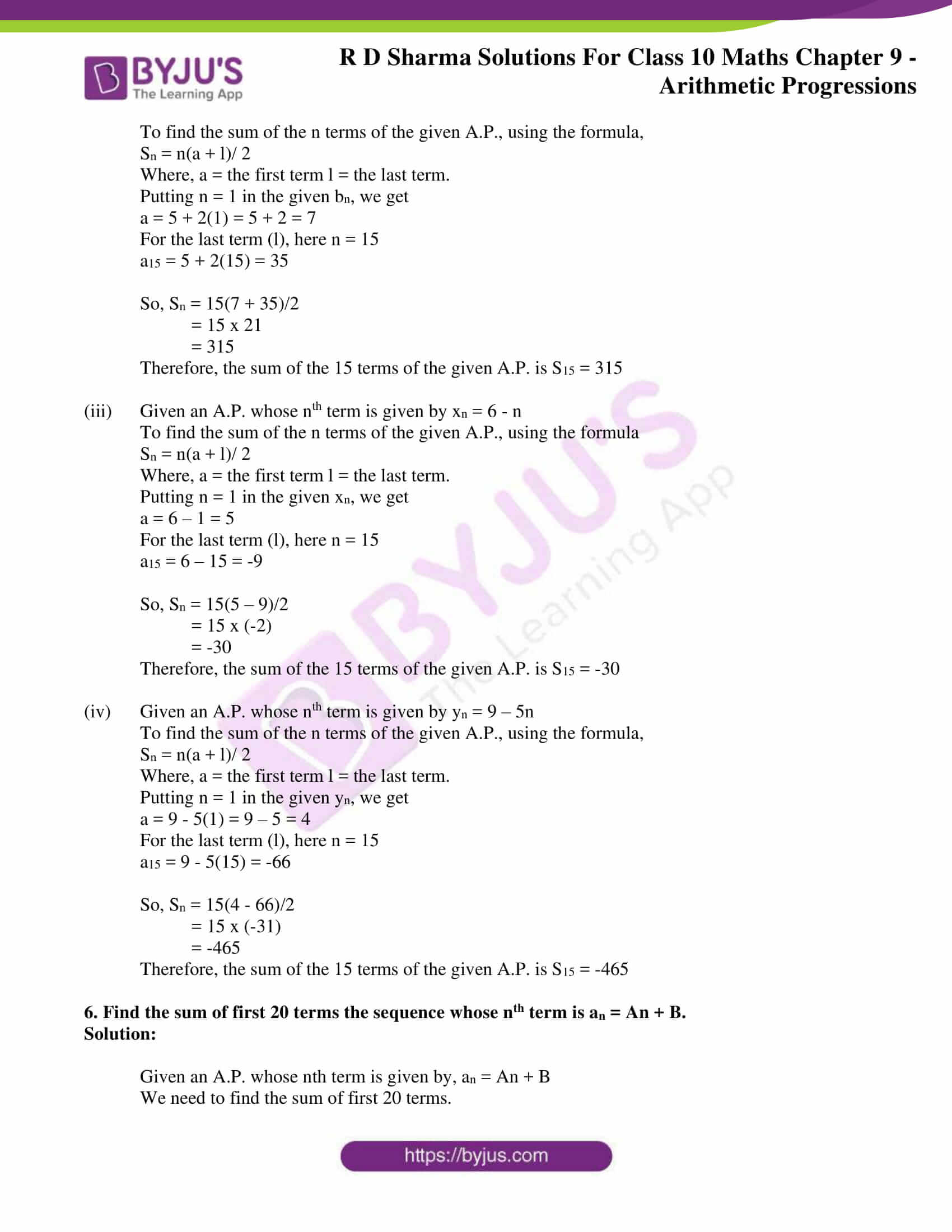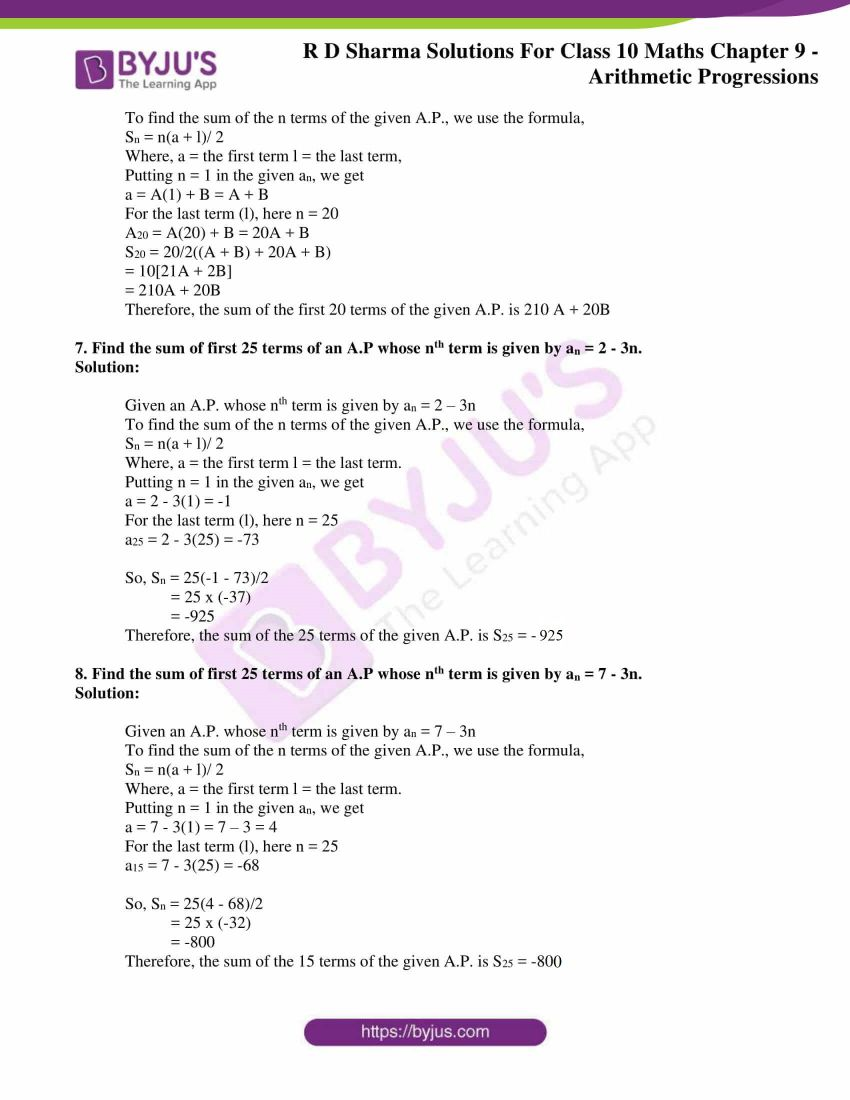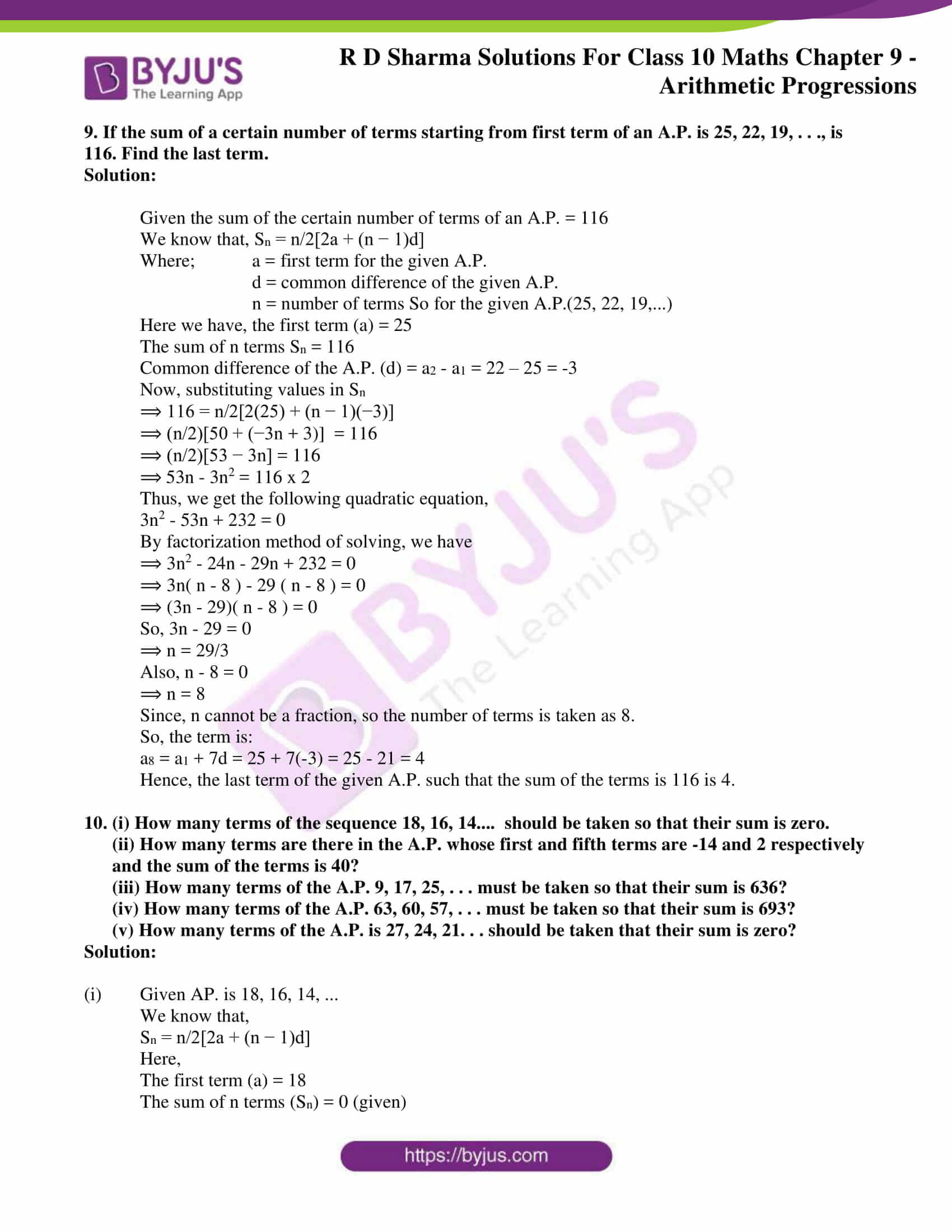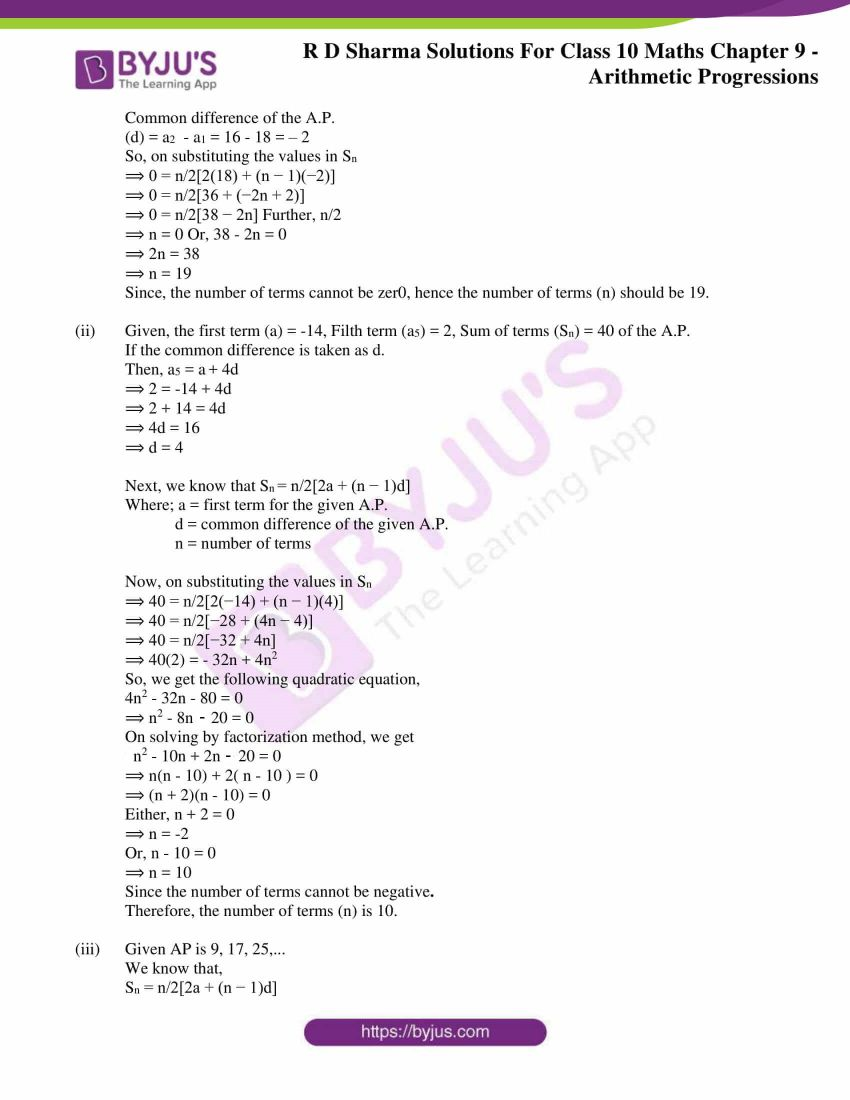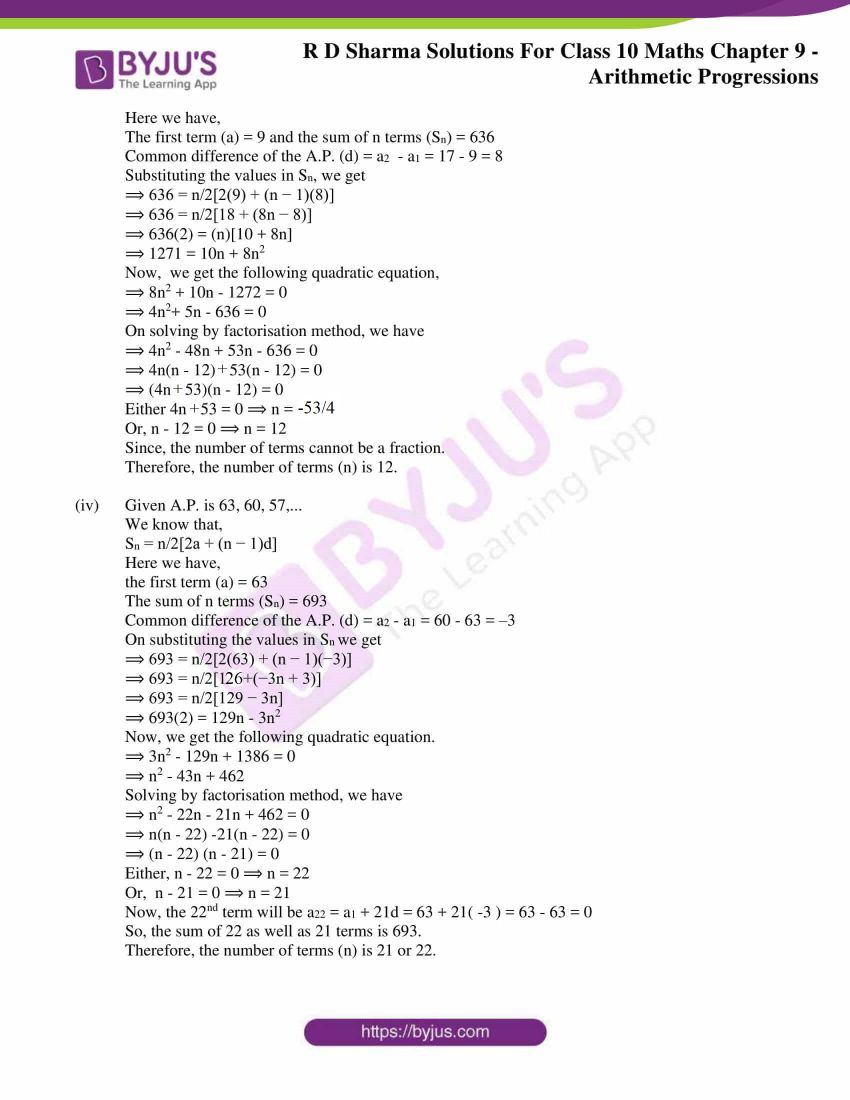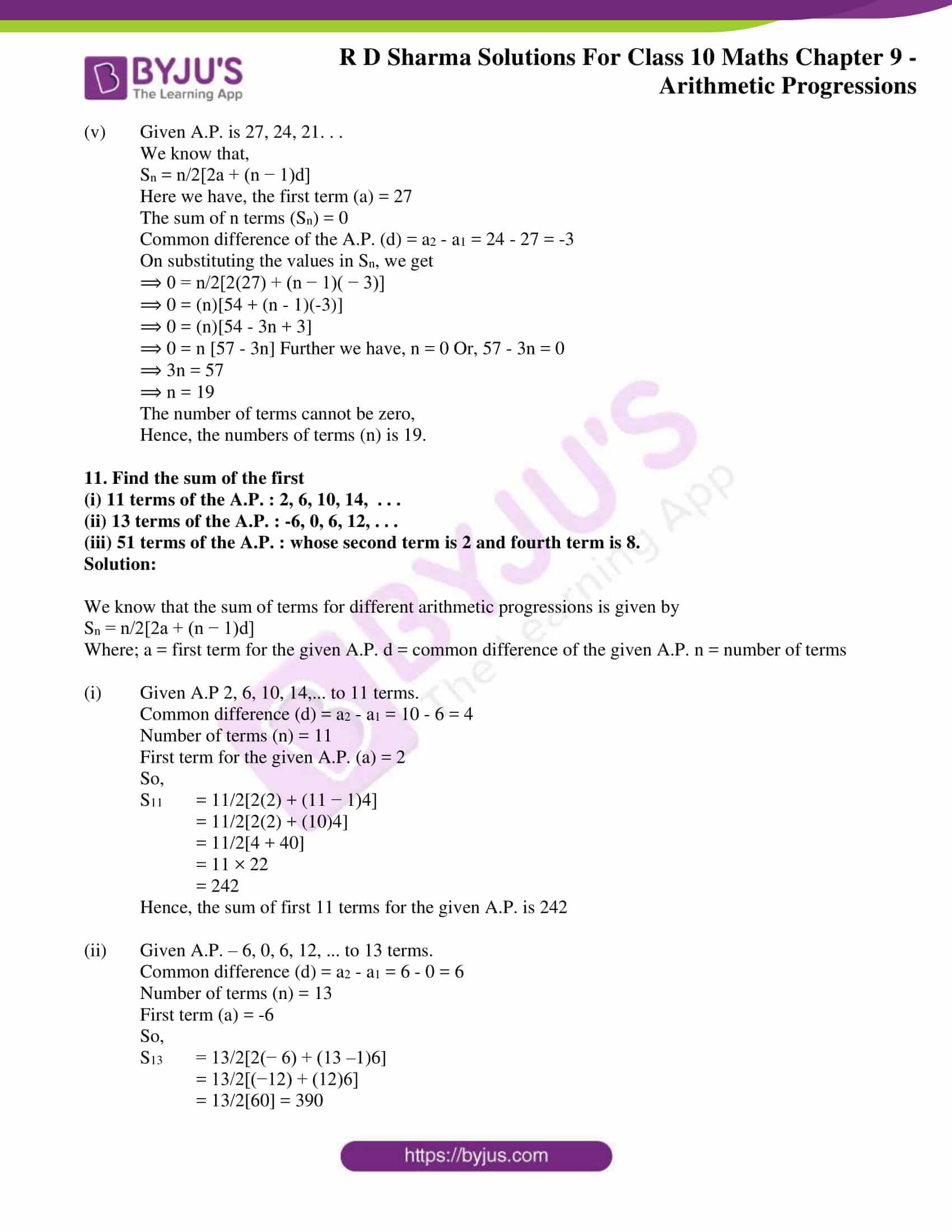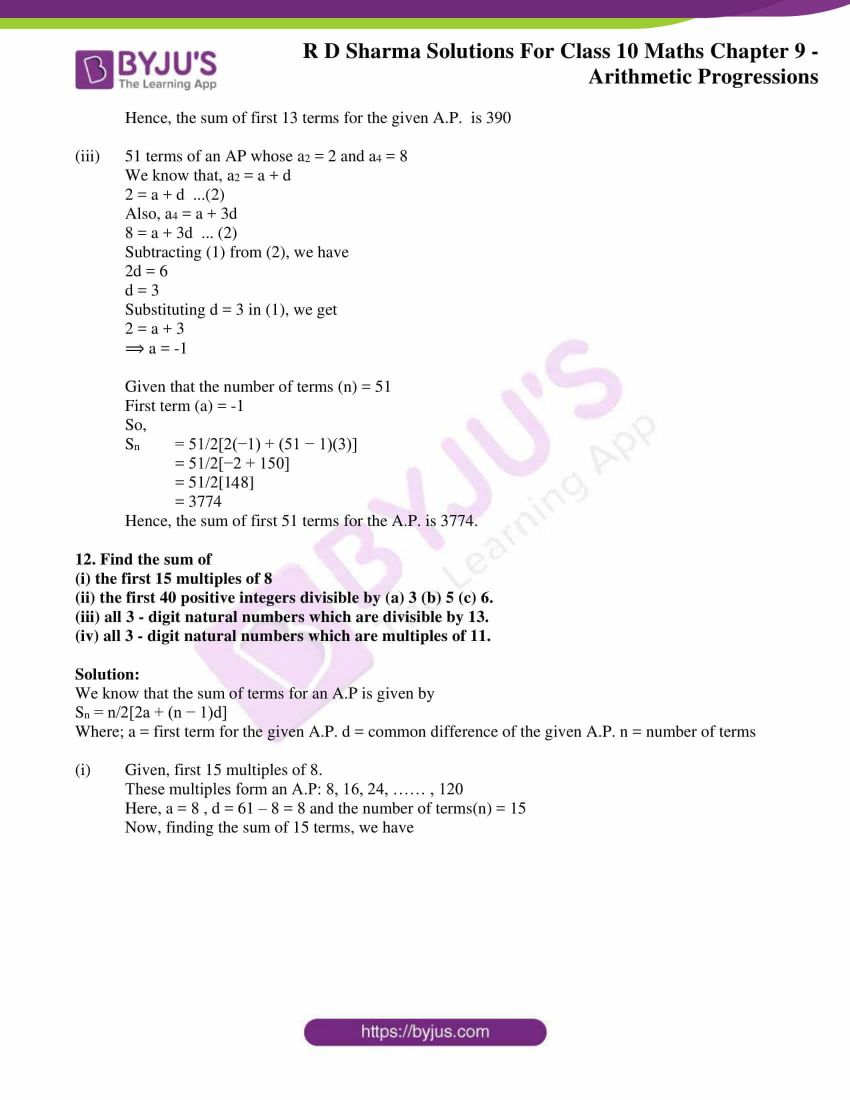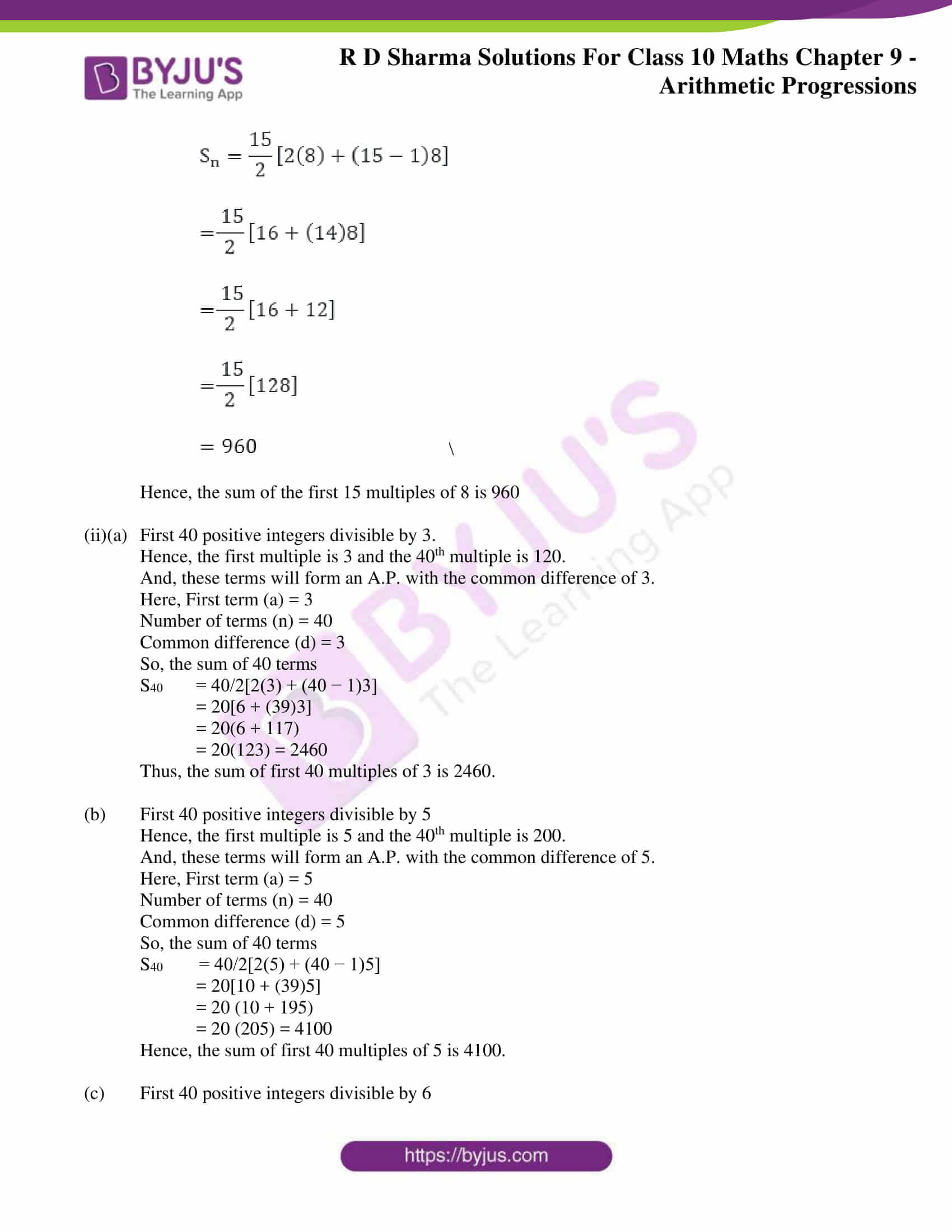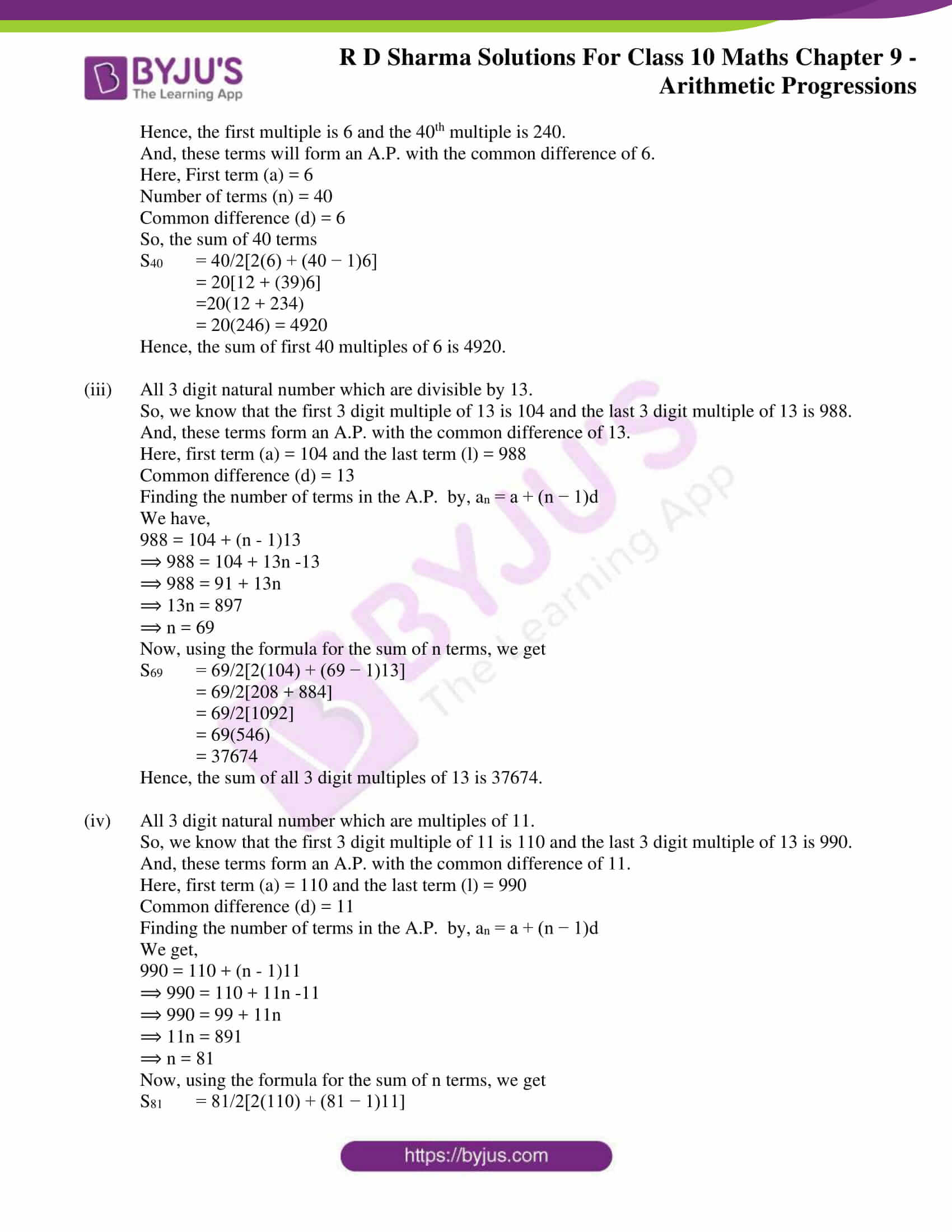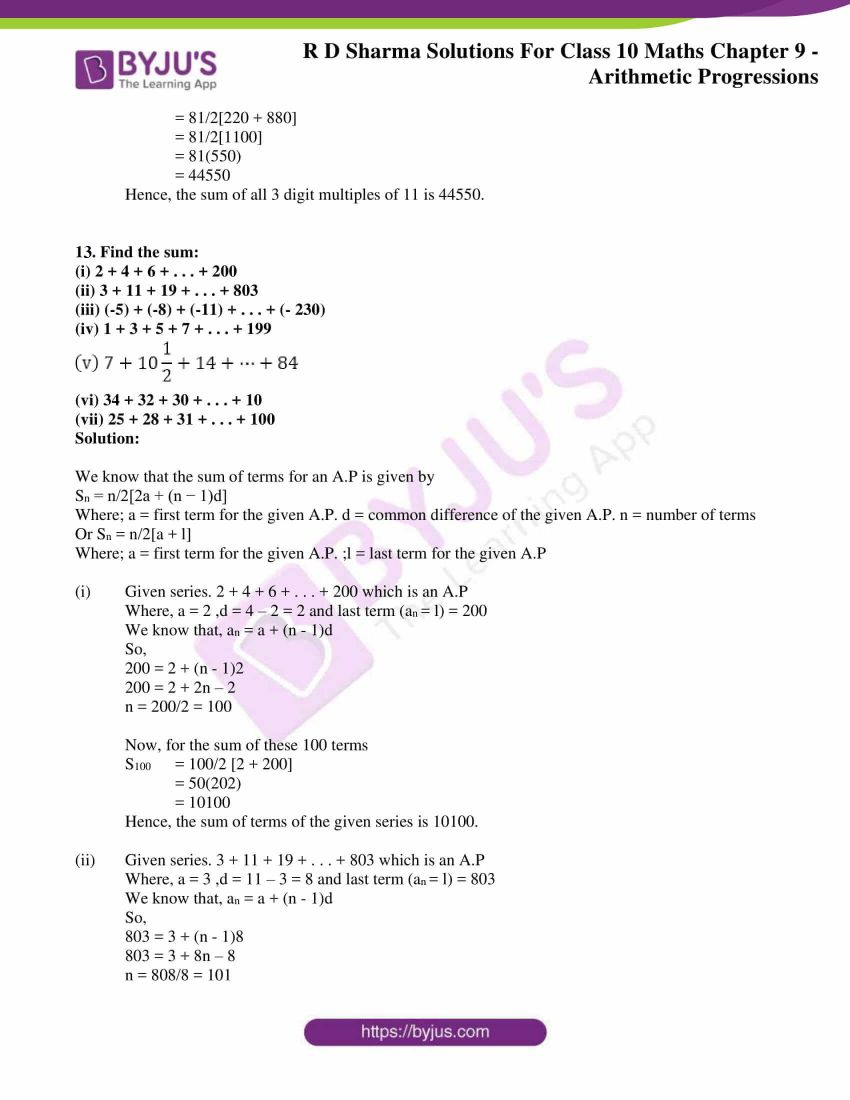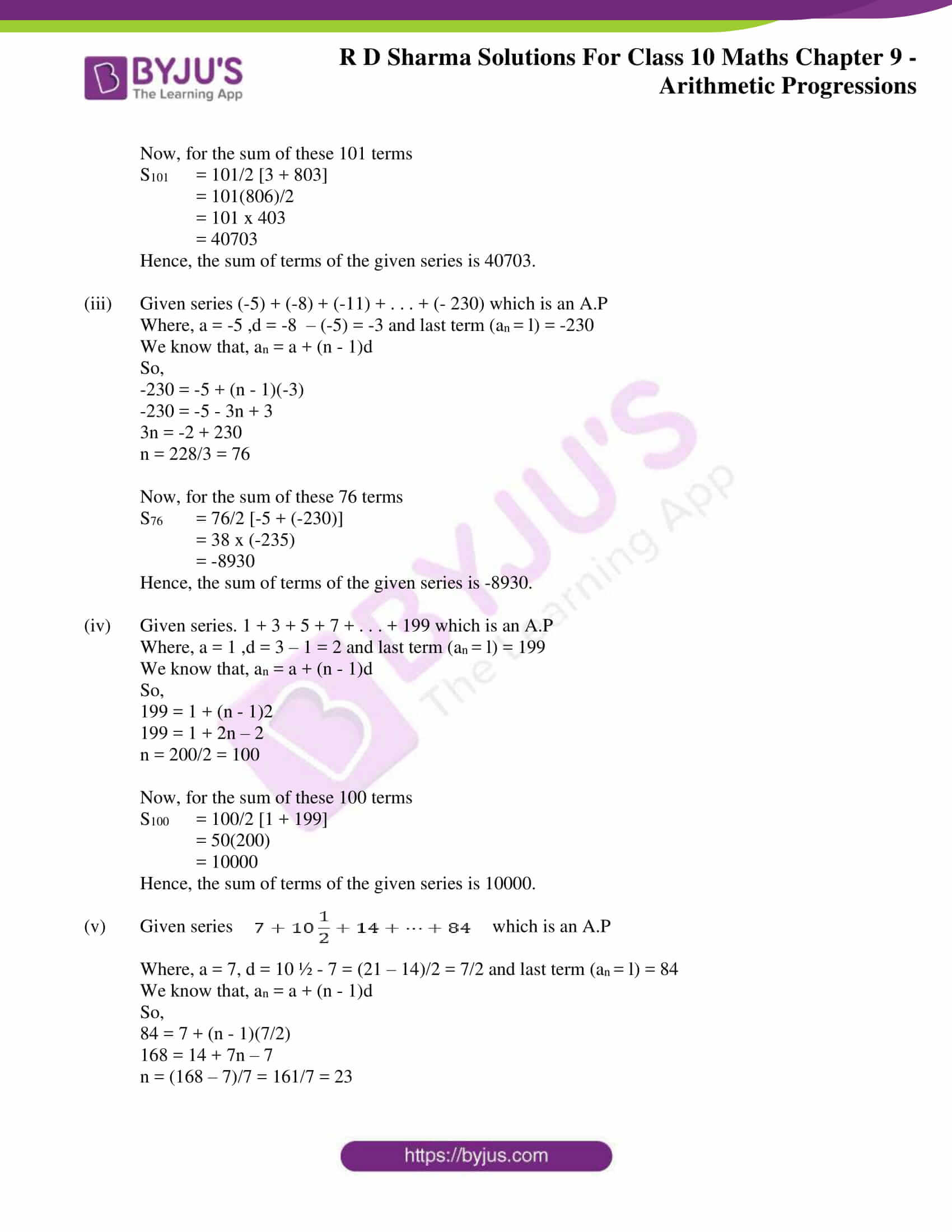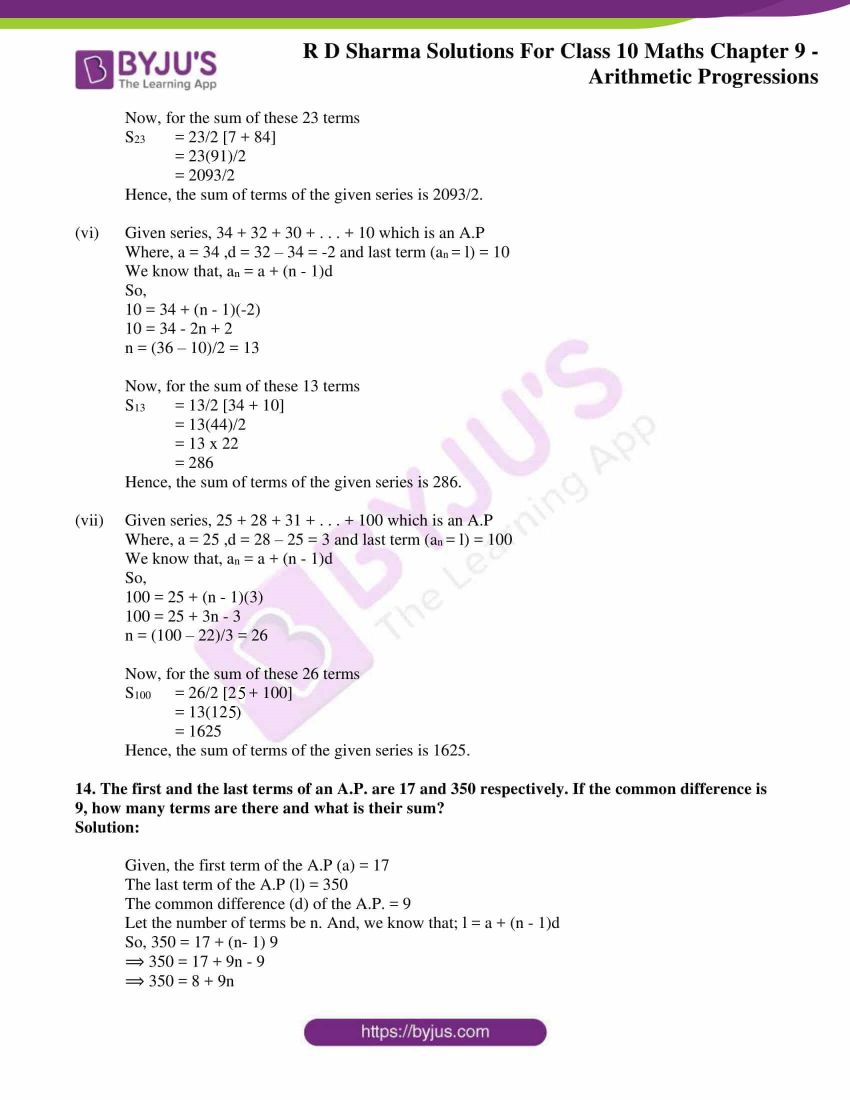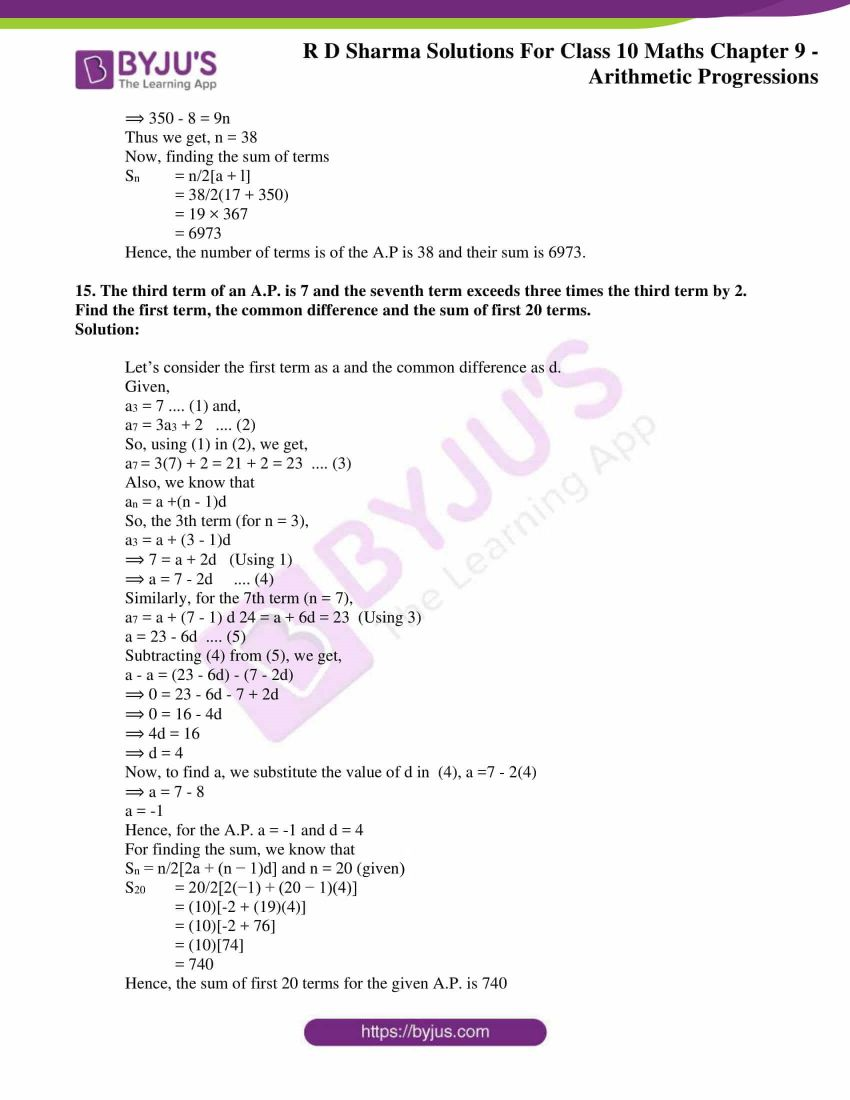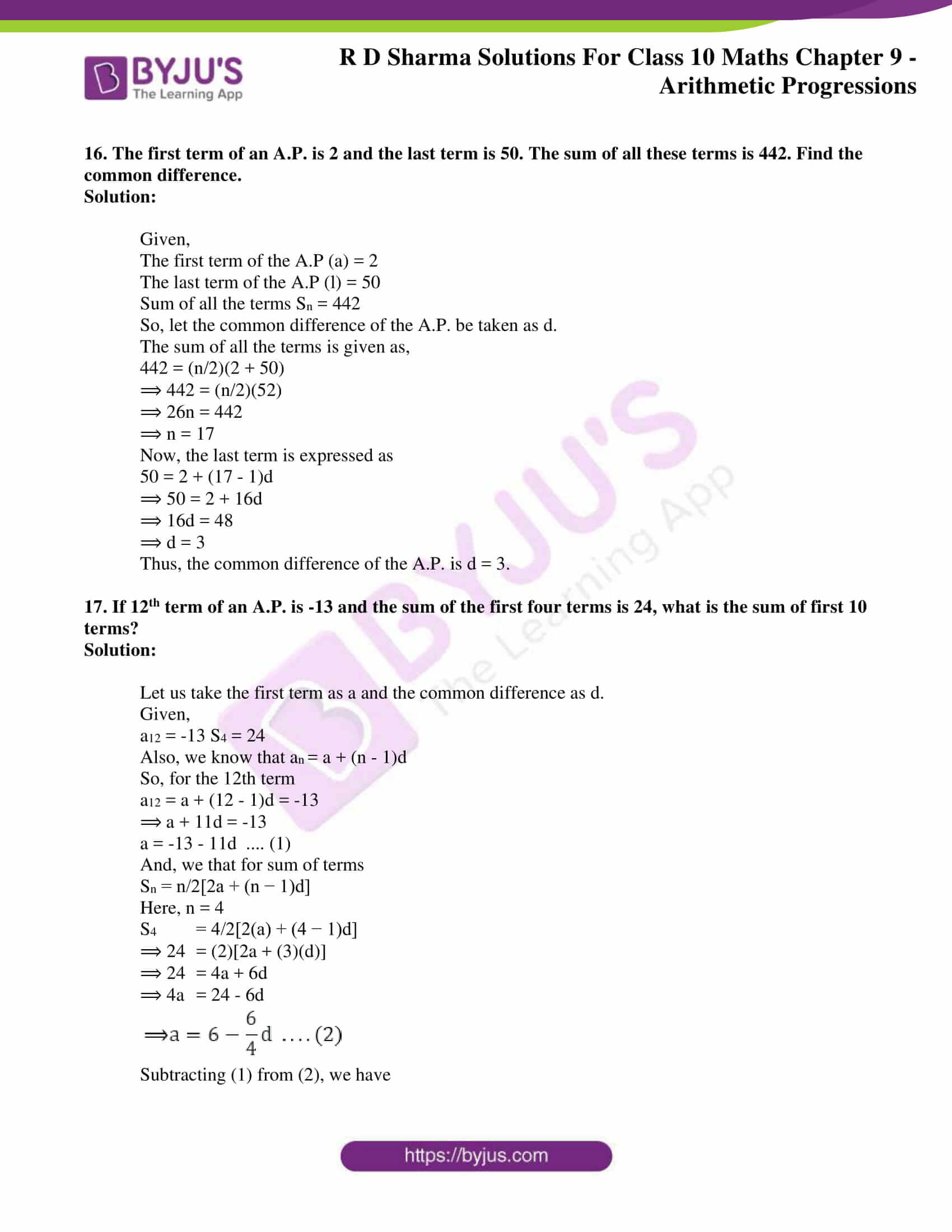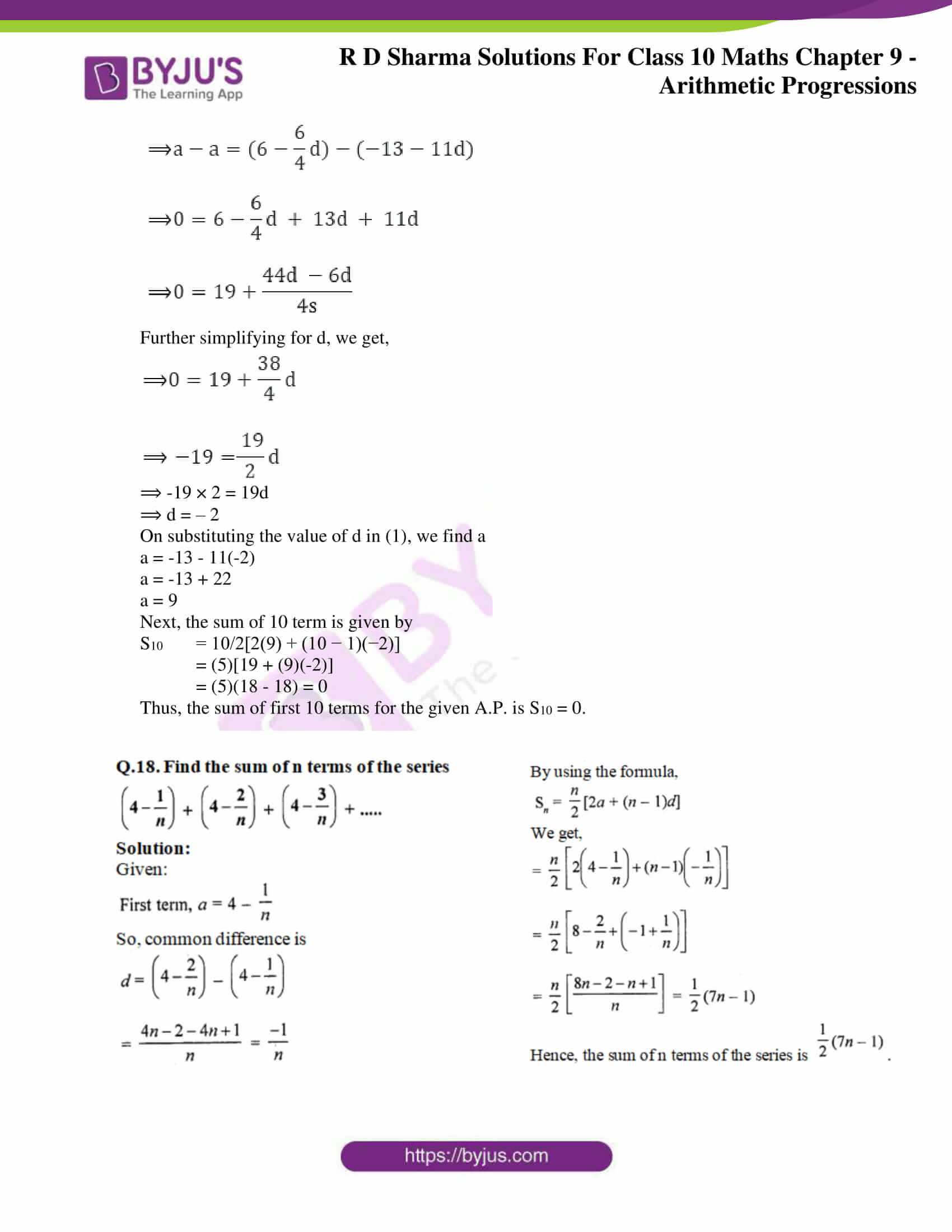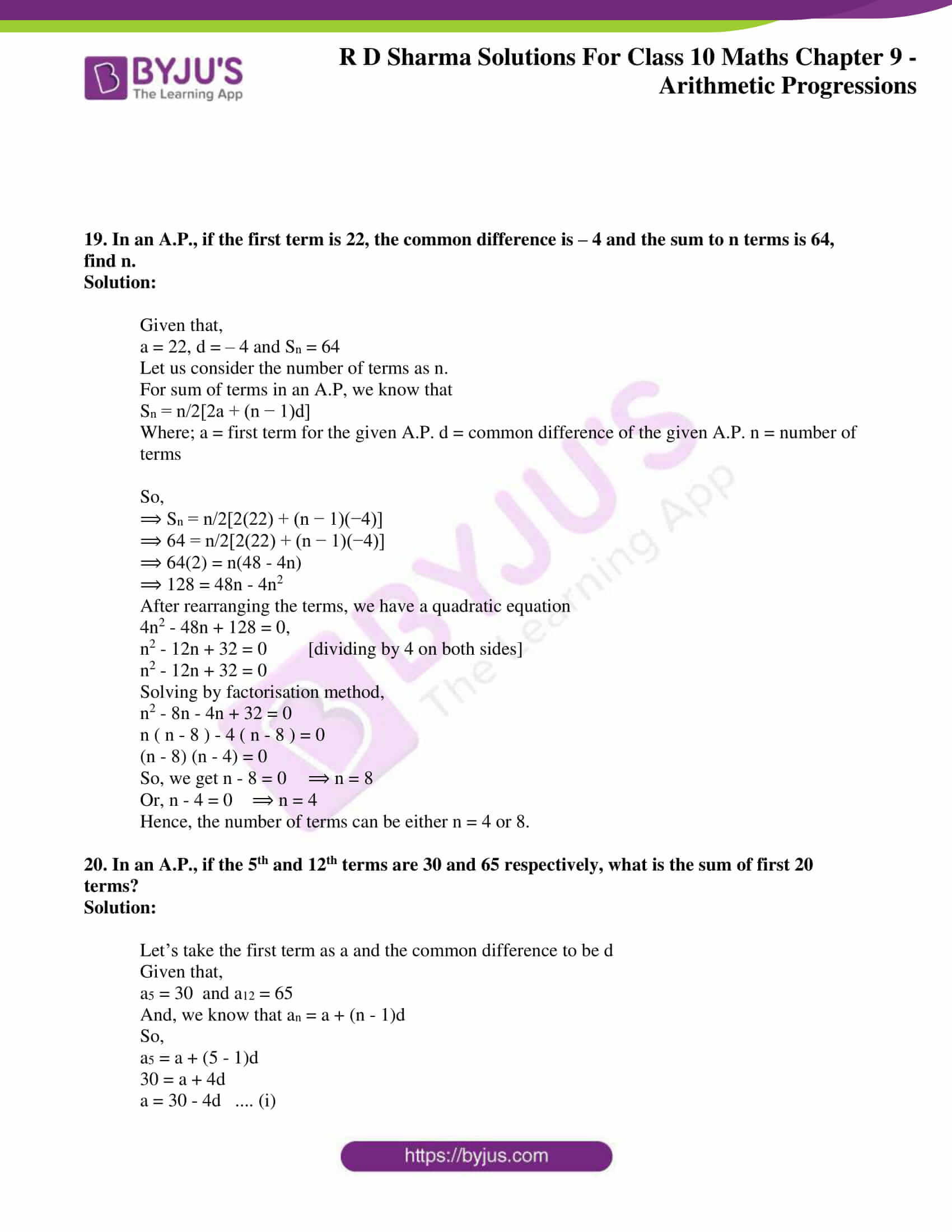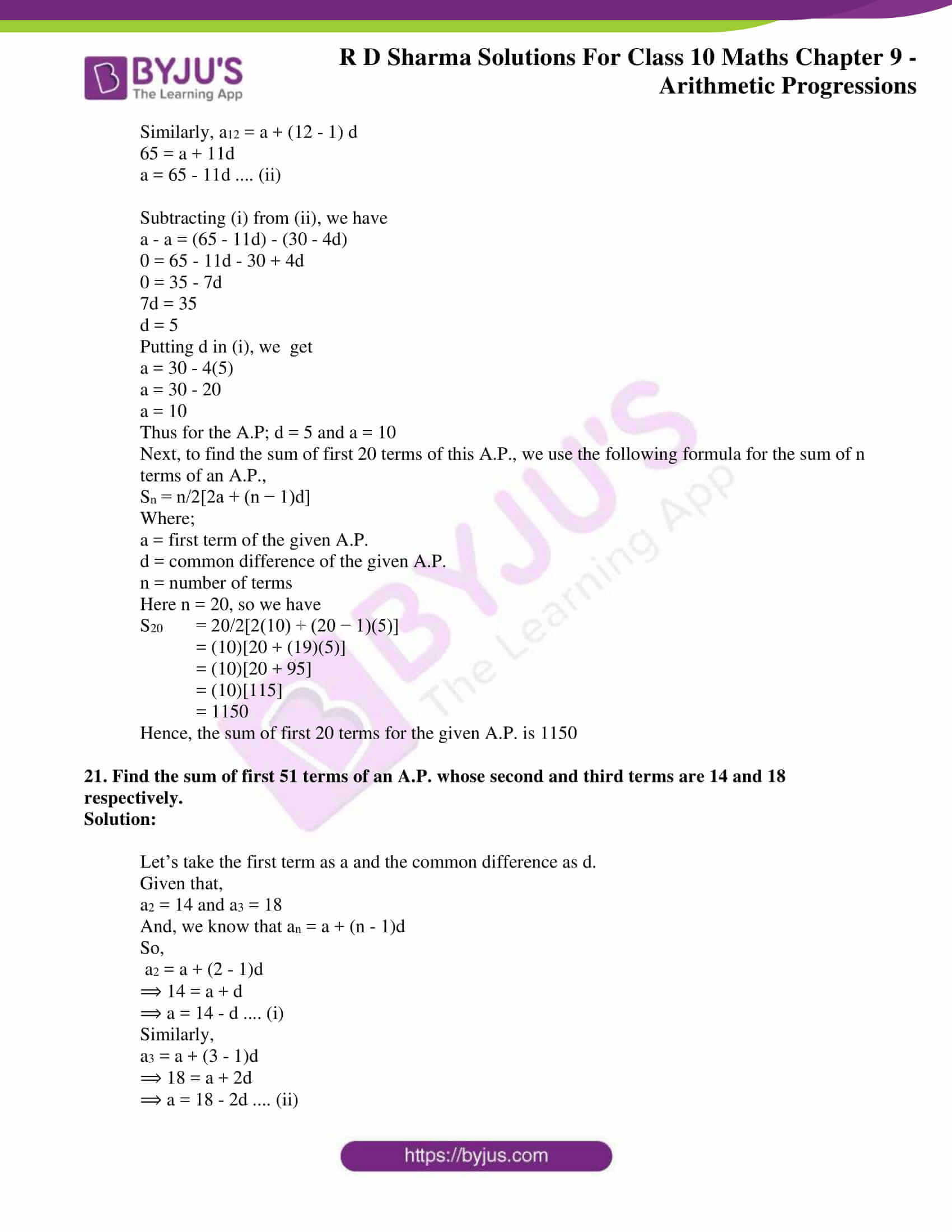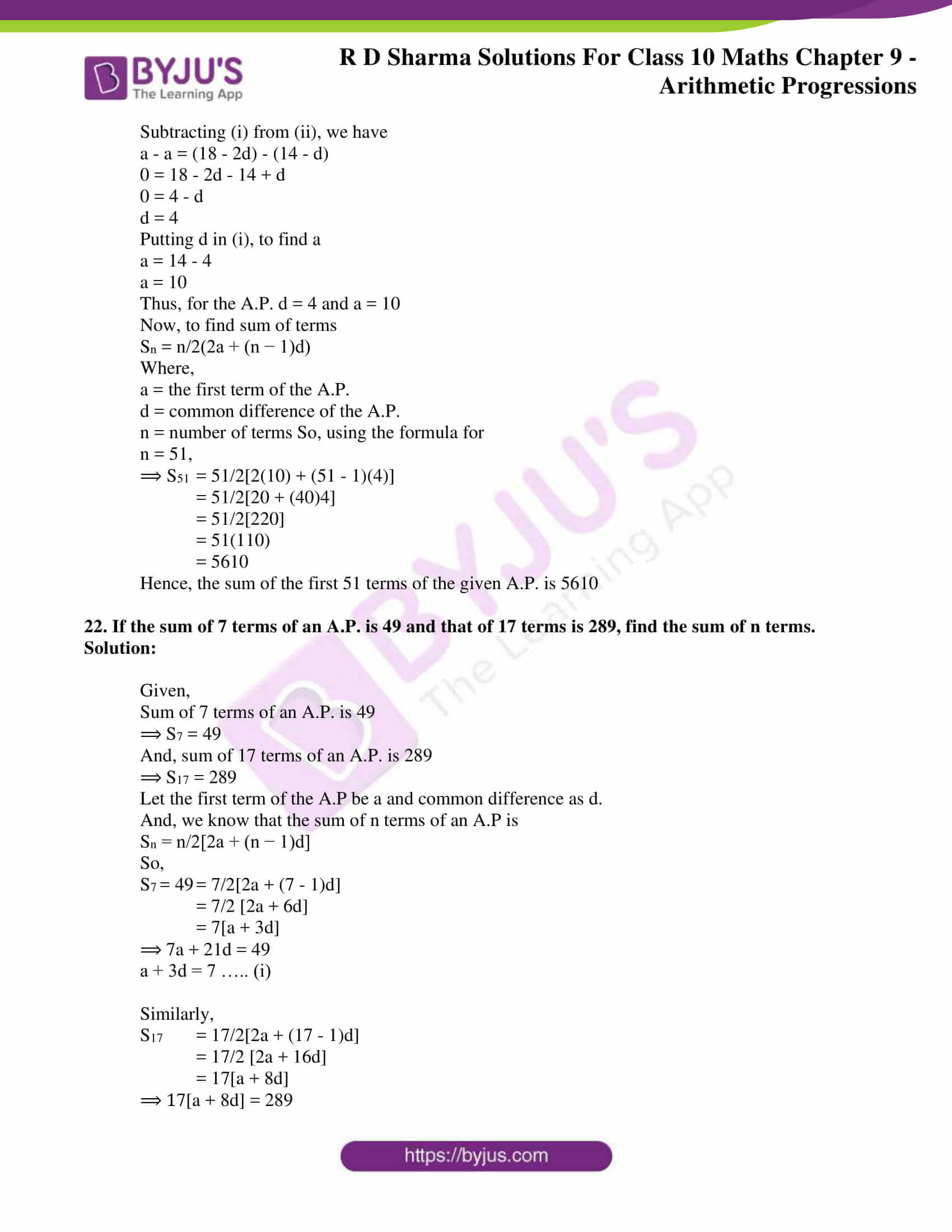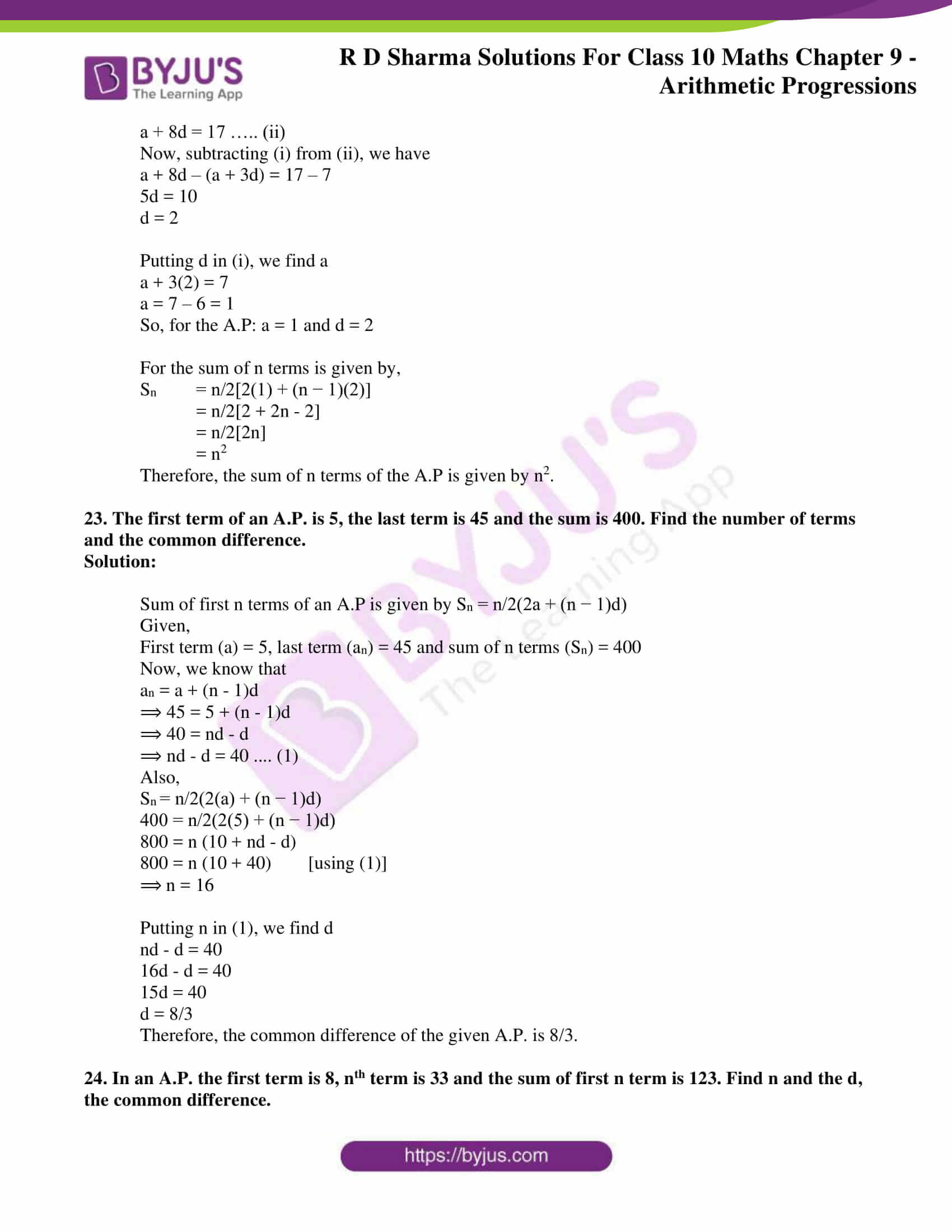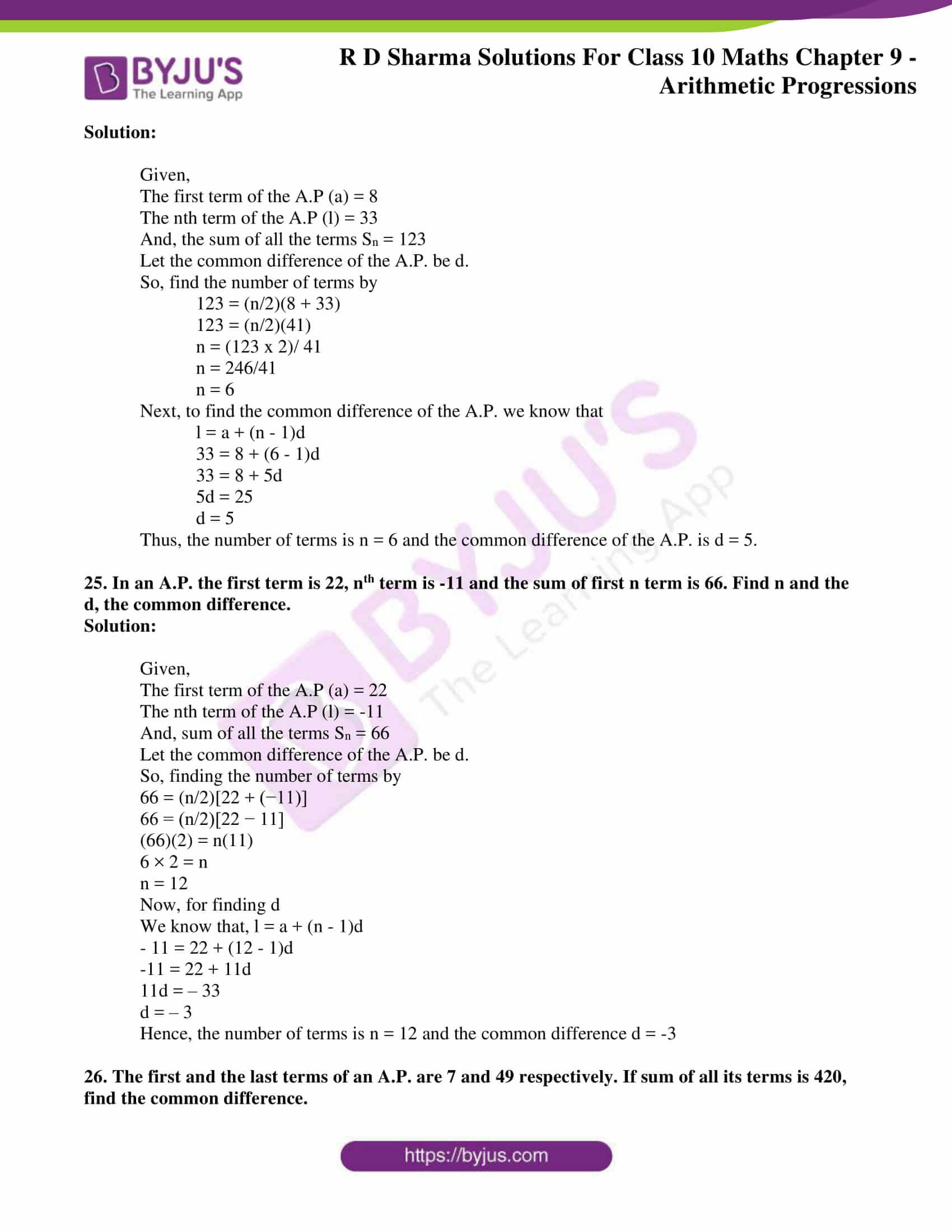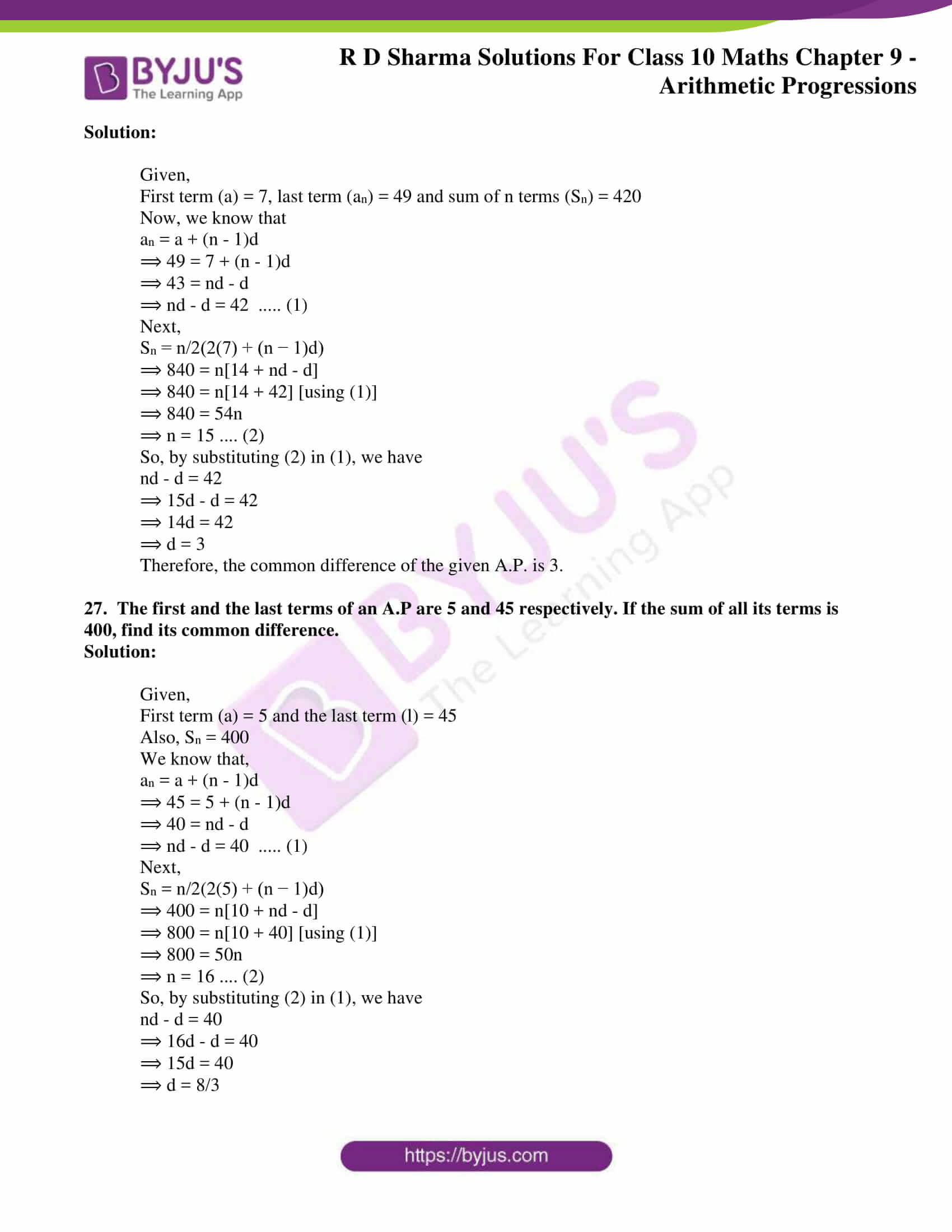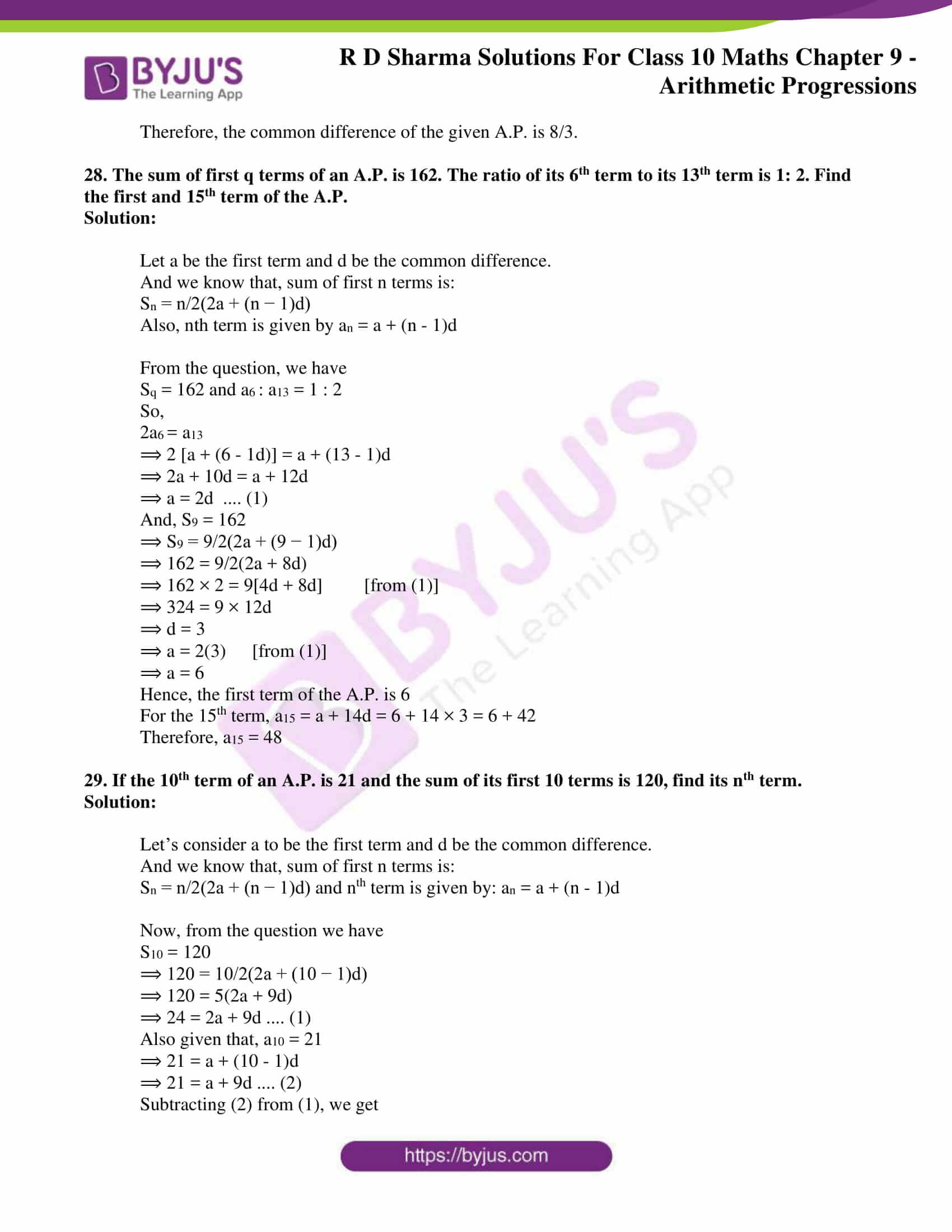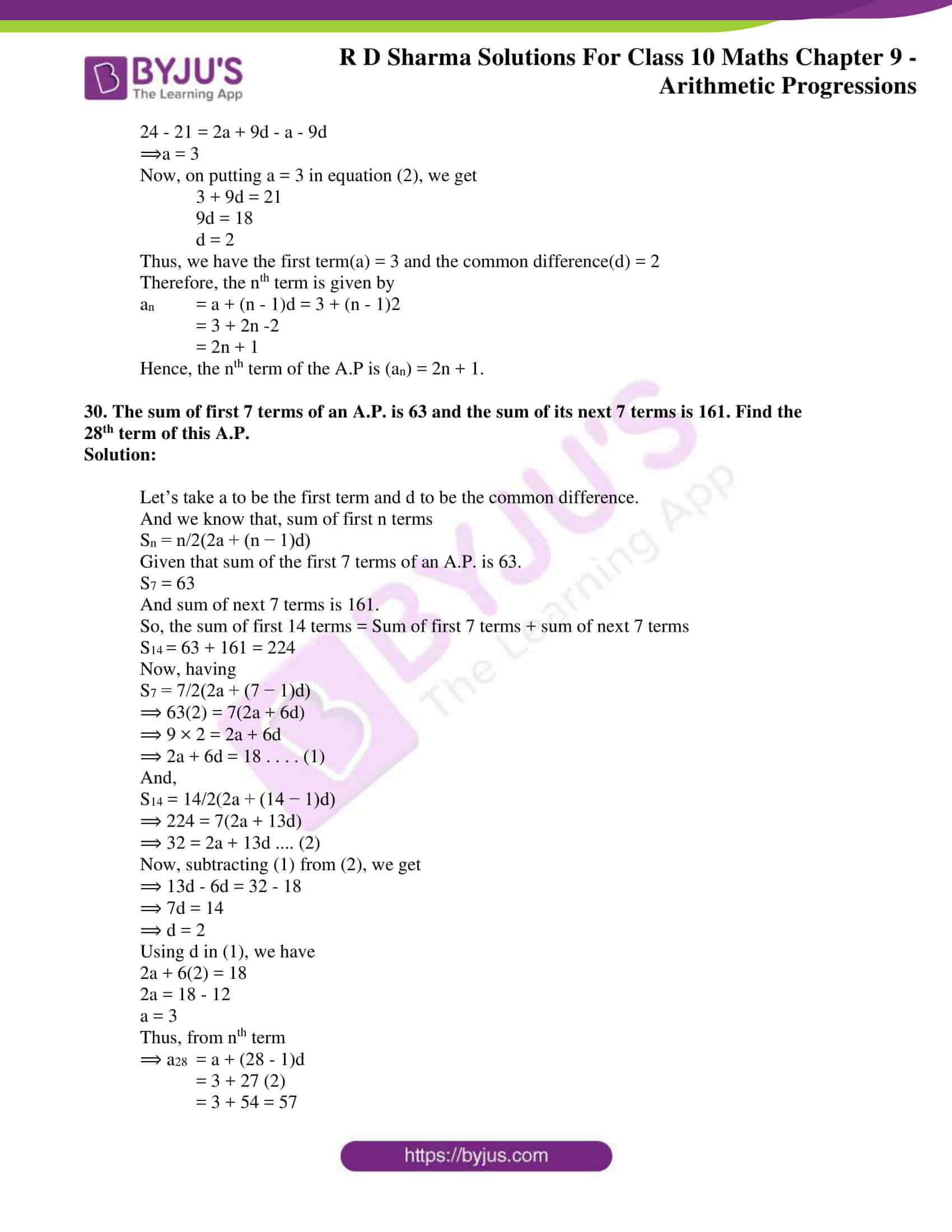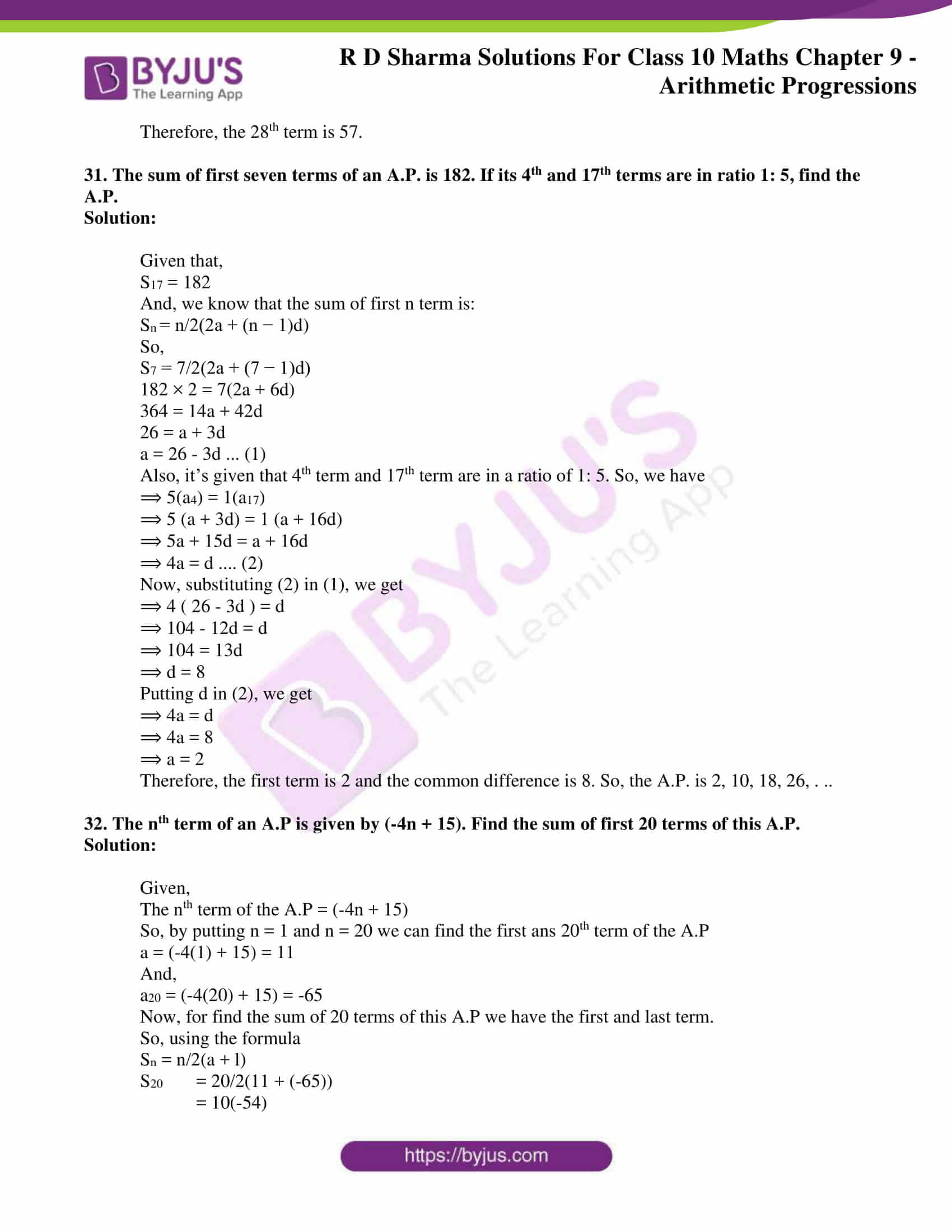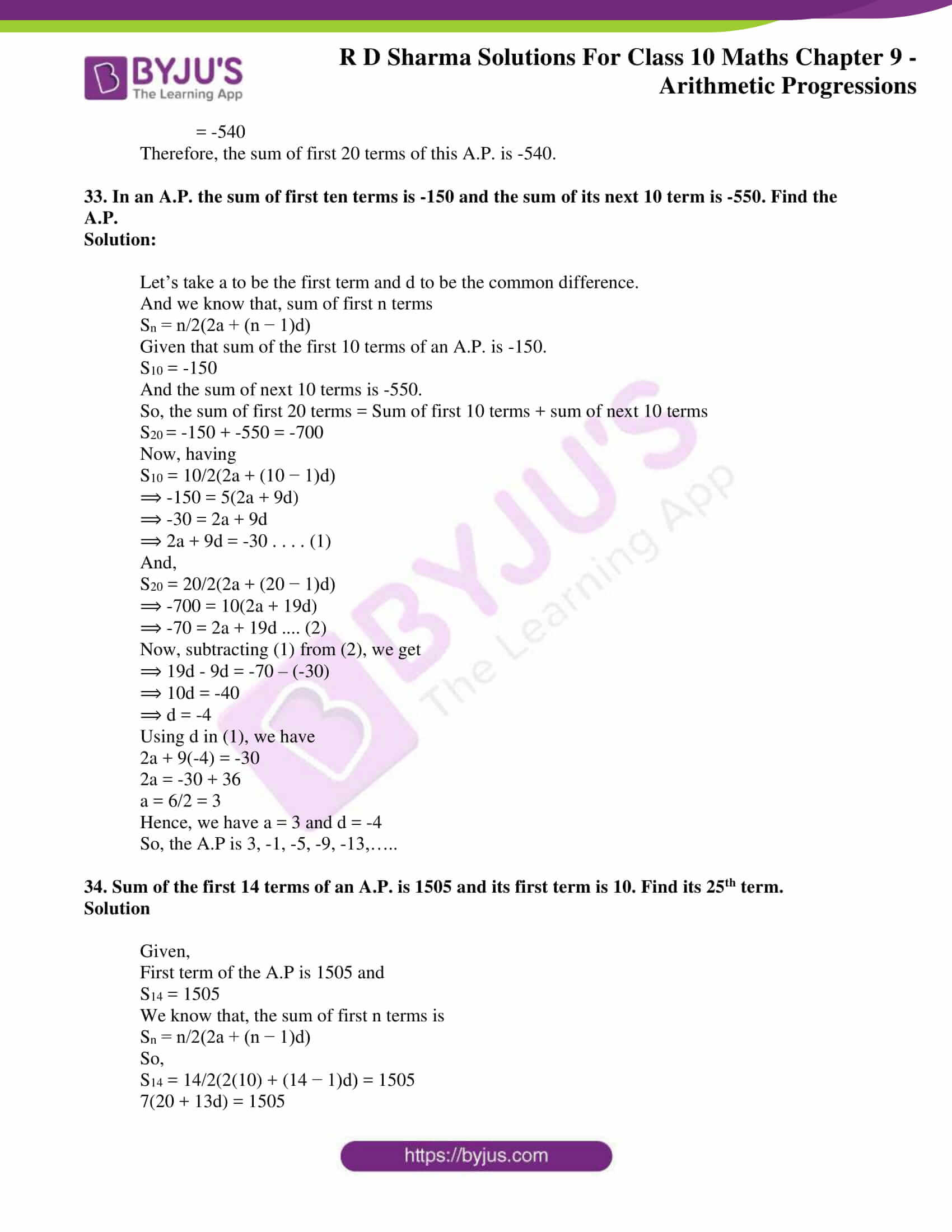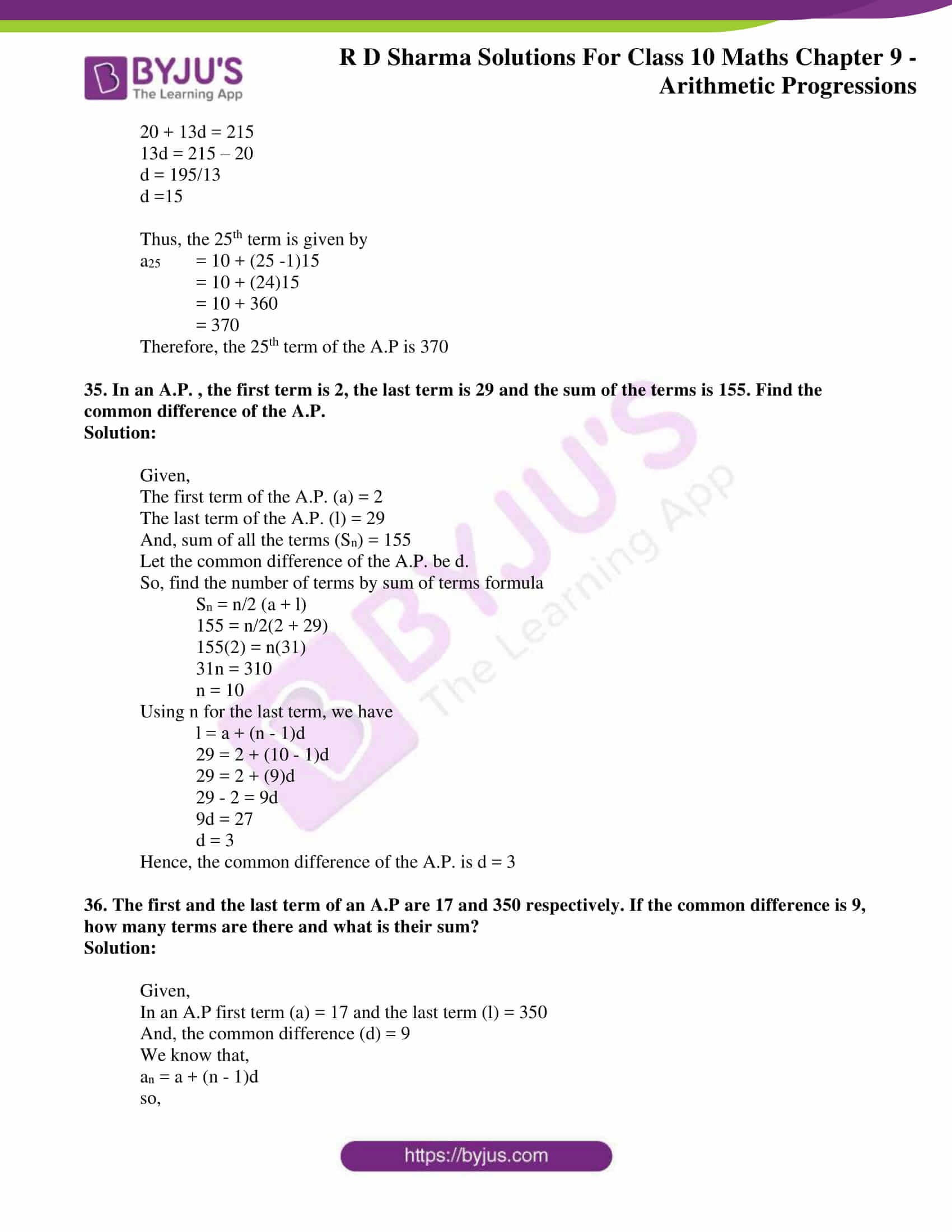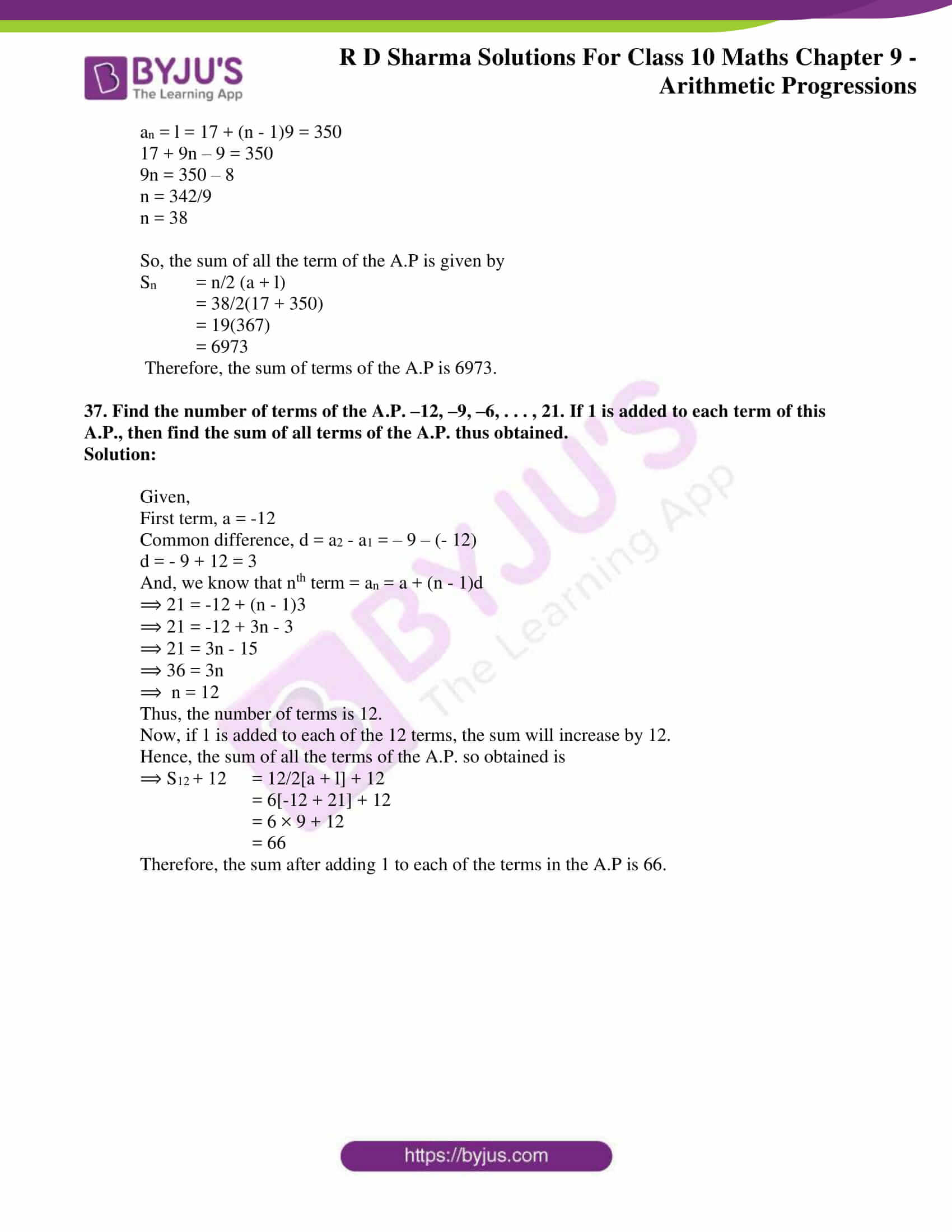### RD Sharma Solutions for Class 10 Maths Chapter 9 Exercise 9.1 Page No: 9.5

1. Write the first terms of each of the following sequences whose nth term are:

(i) an = 3n + 2

(ii) an = (n – 2)/3

(iii) an = 3n

(iv) an = (3n – 2)/ 5

(v) an = (-1)n . 2n

(vi) an = n(n – 2)/2

(vii) an = n2 – n + 1

(viii) an = n2 – n + 1

(ix) an = (2n – 3)/ 6

Solutions:

(i) an = 3n + 2

Given sequence whose an = 3n + 2

To get the first five terms of given sequence, put n = 1, 2, 3, 4, 5 and we get

a1 = (3 × 1) + 2 = 3 + 2 = 5

a2 = (3 × 2) + 2 = 6 + 2 = 8

a3 = (3 × 3) + 2 = 9 + 2 = 11

a4 = (3 × 4) + 2 = 12 + 2 = 14

a5 = (3 × 5) + 2 = 15 + 2 = 17

∴ the required first five terms of the sequence whose nth term, an = 3n + 2 are 5, 8, 11, 14, 17.

(ii) an = (n – 2)/3

Given sequence whose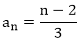On putting n = 1, 2, 3, 4, 5 then can get the first five terms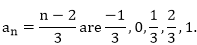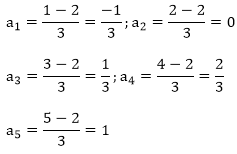∴ the required first five terms of the sequence whose nth term,

(iii) an = 3n

Given sequence whose an = 3n

To get the first five terms of given sequence, put n = 1, 2, 3, 4, 5 in the above

a1 = 31 = 3;

a2 = 32 = 9;

a3 = 27;

a4 = 34 = 81;

a5 = 35 = 243.

∴ the required first five terms of the sequence whose nth term, an = 3n are 3, 9, 27, 81, 243.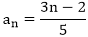(iv) an = (3n – 2)/ 5

Given sequence whose

To get the first five terms of the sequence, put n = 1, 2, 3, 4, 5 in the above

And, we get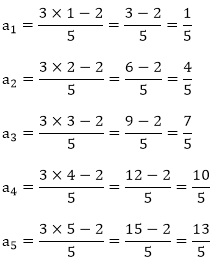∴ the required first five terms of the sequence are 1/5, 4/5, 7/5, 10/5, 13/5

(v) an = (-1)n2n

Given sequence whose an = (-1)n2n

To get first five terms of the sequence, put n = 1, 2, 3, 4, 5 in the above.

a1 = (-1)1.21 = (-1).2 = -2

a2 = (-1)2.22 = (-1).4 = 4

a3 = (-1)3.23 = (-1).8 = -8

a4 = (-1)4.24 = (-1).16 = 16

a5 = (-1)5.25 = (-1).32 = -32

∴ the first five terms of the sequence are – 2, 4, – 8, 16, – 32.

(vi) an = n(n – 2)/2

The given sequence is,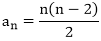To get the first five terms of the sequence, put n = 1, 2, 3, 4, 5.

And, we get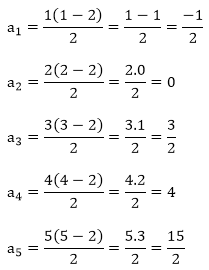∴ the required first five terms are -1/2, 0, 3/2, 4, 15/2

(vii) an = n2 – n + 1

The given sequence whose, an = n2 – n + 1

To get the first five terms of given sequence, put n = 1, 2, 3, 4, 5.

And, we get

a1 = 12 – 1 + 1 = 1

a2 = 22 – 2 + 1 = 3

a3 = 32 – 3 + 1 = 7

a4 = 42 – 4 + 1 = 13

a5 = 52 – 5 + 1 = 21

∴ the required first five terms of the sequence are 1, 3, 7, 13, 21.

(viii) an = 2n2 – 3n + 1

The given sequence whose an = 2n2 – 3n + 1

To get the first five terms of the sequence, put n = 1, 2, 3, 4, 5.

And, we get

a1 = 2.12 – 3.1 + 1 = 2 – 3 + 1 = 0

a2 = 2.22 – 3.2 + 1 = 8 – 6 + 1 = 3

a3 = 2.32 – 3.3 + 1 = 18 – 9 + 1 = 10

a4 = 2.42 – 3.4 + 1 = 32 – 12 + 1 = 21

a5 = 2.52 – 3.5 + 1 = 50 – 15 + 1 = 36

∴ the required first five terms of the sequence are 0, 3, 10, 21, 36.

(ix) an = (2n – 3)/ 6

Given sequence whose,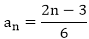To get the first five terms of the sequence we put n = 1, 2, 3, 4, 5.

And, we get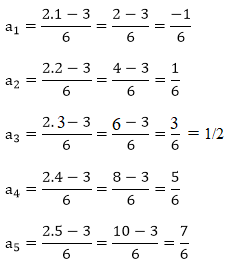∴ the required first five terms of the sequence are -1/6, 1/6, 1/2, 5/6 and 7/6

### RD Sharma Solutions for Class 10 Maths Chapter 9 Exercise 9.2 Page No: 9.8

1. Show that the sequence defined by an = 5n – 7 is an A.P., find its common difference.

Solution:

Given, an = 5n – 7

Now putting n = 1, 2, 3, 4 we get,

a1 = 5(1) – 7 = 5 – 7 = -2

a2 = 5(2) – 7 = 10 – 7 = 3

a3 = 5(3) – 7 = 15 – 7 = 8

a4 = 5(4) – 7 = 20 – 7 = 13

We can see that,

a2 – a1 = 3 – (-2) = 5

a3 – a2 = 8 – (3) = 5

a4 – a3 = 13 – (8) = 5

Since the difference between the terms is common, we can conclude that the given sequence defined by an = 5n – 7 is an A.P with common difference 5.

2. Show that the sequence defined by an = 3n2 – 5 is not an A.P.

Solution:

Given, an = 3n2 – 5

Now putting n = 1, 2, 3, 4 we get,

a1 = 3(1)2 – 5= 3 – 5 = -2

a2 = 3(2)2 – 5 = 12 – 5 = 7

a3 = 3(3)2 – 5 = 27 – 5 = 22

a4 = 3(4)2 – 5 = 48 – 5 = 43

We can see that,

a2 – a1 = 7 – (-2) = 9

a3 – a2 = 22 – 7 = 15

a4 – a3 = 43 – 22 = 21

Since the difference between the terms is not common and varying, we can conclude that the given sequence defined by an = 3n2 – 5 is not an A.P.

3. The general term of a sequence is given by an = -4n + 15. Is the sequence an A.P.? If so, find its 15th term and the common difference.

Solution:

Given, an = -4n + 15

Now putting n = 1, 2, 3, 4 we get,

a1 = -4(1) + 15 = -4 + 15 = 11

a2 = -4(2) + 15 = -8 + 15 = 7

a3 = -4(3) + 15 = -12 + 15 = 3

a4 = -4(4) + 15 = -16 + 15 = -1

We can see that,

a2 – a1 = 7 – (11) = -4

a3 – a2 = 3 – 7 = -4

a4 – a3 = -1 – 3 = -4

Since the difference between the terms is common, we can conclude that the given sequence defined by an = -4n + 15 is an A.P with common difference of -4.

Hence, the 15th term will be

a15 = -4(15) + 15 = -60 + 15 = -45

### RD Sharma Solutions for Class 10 Maths Chapter 9 Exercise 9.3 Page No: 9.11

1. For the following arithmetic progressions write the first term a and the common difference d:

(i) – 5, -1, 3, 7,…

(ii) 1/5, 3/5, 5/5, 7/5,…

(iii) 0.3, 0.55, 0.80, 1.05,…

(iv) -1.1, – 3.1, – 5.1, – 7.1,…

Solution:

We know that if a is the first term and d is the common difference, the arithmetic progression is a, a + d, a + 2d + a + 3d,….

(i) – 5, –1, 3, 7,…

Given arithmetic series is – 5, –1, 3, 7,…

c a, a + d, a + 2d + a + 3d,….

Thus, by comparing these two we get, a = – 5, a + d = 1, a + 2d = 3, a + 3d = 7

First term (a) = – 5

By subtracting second and first term, we get

(a + d) – (a) = d

-1 – (- 5) = d

4 = d

⇒ Common difference (d) = 4.

(ii) 1/5, 3/5, 5/5, 7/5, ………….

Given arithmetic series is 1/5, 3/5, 5/5, 7/5, ……………

It is seen that, it’s of the form of 1/5, 2/5, 5/5, 7/5, ……….. a, a + d, a + 2d, a + 3d,

Thus, by comparing these two, we get

a = 1/5, a + d = 3/5, a + 2d = 5/5, a + 3d = 7/5

First term (a) = 1/5

By subtracting first term from second term, we get

d = (a + d)-(a)

d = 3/5 – 1/5

d = 2/5

⇒ common difference (d) = 2/5

(iii) 0.3, 0.55, 0.80, 1.05, …………

Given arithmetic series 0.3, 0.55, 0.80, 1.05, ……….

It is seen that, it’s of the form of a, a + d, a + 2d, a + 3d,

Thus, by comparing we get,

a = 0.3, a + d = 0.55, a + 2d = 0.80, a + 3d = 1.05

First term (a) = 0.3.

By subtracting first term from second term. We get

d = (a + d) – (a)

d = 0.55 – 0.3

d = 0.25

⇒ Common difference (d) = 0.25

(iv) –1.1, – 3.1, – 5.1, –7.1, ……..

General series is –1.1, – 3.1, – 5.1, –7.1, ……..

It is seen that, it’s of the form of a, a + d, a + 2d, a + 3d, ………..

Thus, by comparing these two, we get

a = –1.1, a + d = –3.1, a + 2d = –5.1, a + 3d = –7.1

First term (a) = –1.1

Common difference (d) = (a + d) – (a)

= -3.1 – ( – 1.1)

⇒ Common difference (d) = – 2

2. Write the arithmetic progression when first term a and common difference d are as follows:

(i) a = 4, d = – 3

(ii) a = –1, d = 1/2

(iii) a = –1.5, d = – 0.5

Solution:

We know that, if first term (a) = a and common difference = d, then the arithmetic series is: a, a + d, a + 2d, a + 3d,

(i) a = 4, d = -3

Given, first term (a) = 4

Common difference (d) = -3

Then arithmetic progression is: a, a + d, a + 2d, a + 3d, ……

⇒ 4, 4 – 3, 4 + 2(-3), 4 + 3(-3), ……

⇒ 4, 1, – 2, – 5, – 8 ……..

(ii) a = -1, d = 1/2

Given, first term (a) = -1

Common difference (d) = 1/2

Then arithmetic progression is: a, a + d, a + 2d, a + 3d,

⇒ -1, -1 + 1/2, -1 + 2½, -1 + 3½, …

⇒ -1, -1/2, 0, 1/2

(iii) a = –1.5, d = – 0.5

Given First term (a) = –1.5

Common difference (d) = – 0.5

Then arithmetic progression is; a, a + d, a + 2d, a + 3d, ……

⇒ -1.5, -1.5 + (-0.5), –1.5 + 2(– 0.5), –1.5 + 3(– 0.5)

⇒ – 1.5, – 2, – 2.5, – 3, …….

3. In which of the following situations, the sequence of numbers formed will form an A.P.?
(i) The cost of digging a well for the first metre is Rs 150 and rises by Rs 20 for each succeeding metre.
(ii) The amount of air present in the cylinder when a vacuum pump removes each time 1/4 of their remaining in the cylinder.

(iii) Divya deposited Rs 1000 at compound interest at the rate of 10% per annum. The amount at the end of first year, second year, third year, …, and so on.

Solution:

(i) Given,

Cost of digging a well for the first meter (c1) = Rs.150.

And, the cost rises by Rs.20 for each succeeding meter

Then,

Cost of digging for the second meter (c2) = Rs.150 + Rs 20 = Rs 170

Cost of digging for the third meter (c3) = Rs.170 + Rs 20 = Rs 210

Hence, its clearly seen that the costs of digging a well for different lengths are 150, 170, 190, 210, ….

Evidently, this series is in A∙P.

With first term (a) = 150, common difference (d) = 20

(ii) Given,

Let the initial volume of air in a cylinder be V liters each time 3th/4 of air in a remaining i.e

1 -1/4

First time, the air in cylinder is V.

Second time, the air in cylinder is 3/4 V.

Third time, the air in cylinder is (3/4)2 V.

Thus, series is V, 3/4 V, (3/4)V,(3/4)V, ….

Hence, the above series is not a A.P.

(iii) Given,

Divya deposited Rs 1000 at compound interest of 10% p.a

So, the amount at the end of first year is = 1000 + 0.1(1000) = Rs 1100

And, the amount at the end of second year is = 1100 + 0.1(1100) = Rs 1210

And, the amount at the end of third year is = 1210 + 0.1(1210) = Rs 1331

Cleary, these amounts 1100, 1210 and 1331 are not in an A.P since the difference between them is not the same.

### RD Sharma Solutions for Class 10 Maths Chapter 9 Exercise 9.4 Page No: 9.24

1. Find:

(i) 10th tent of the AP 1, 4, 7, 10….

(ii) 18th term of the AP √2, 3√2, 5√2, …….

(iii) nth term of the AP 13, 8, 3, -2, ……….

(iv) 10th term of the AP -40, -15, 10, 35, ………….

(v) 8th term of the AP 11, 104, 91, 78, ……………

(vi) 11th tenor of the AP 10.0, 10.5, 11.0, 11.2, …………..

(vii) 9th term of the AP 3/4, 5/4, 7/4 + 9/4, ………..

Solution:

(i) Given A.P. is 1, 4, 7, 10, ……….

First term (a) = 1

Common difference (d) = Second term – First term

= 4 – 1 = 3.

We know that, nth term in an A.P = a + (n – 1)d

Then, 10th term in the A.P is 1 + (10 – 1)3

= 1 + 9×3

= 1 + 27

= 28

∴ 10th term of A. P. is 28

(ii) Given A.P. is √2, 3√2, 5√2, …….

First term (a) = √2

Common difference = Second term – First term

= 3√2 – √2

⇒ d = 2√2

We know that, nth term in an A. P. = a + (n – 1)d

Then, 18th term of A. P. = √2 + (18 – 1)2√2

= √2 + 17.2√2

= √2 (1+34)

= 35√2

∴ 18th term of A. P. is 35√2

(iii) Given A. P. is 13, 8, 3, – 2,  …………

First term (a) = 13

Common difference (d) = Second term first term

= 8 – 13 = – 5

We know that, nth term of an A.P. an = a +(n – 1)d

= 13 + (n – 1) – 5

= 13 – 5n + 5

∴ nth term of the A.P is an = 18 – 5n

(iv) Given A. P. is – 40, -15, 10, 35, ……….

First term (a) = -40

Common difference (d) = Second term – fast term

= -15 – (- 40)

= 40 – 15

= 25

We know that, nth term of an A.P. an = a + (n – 1)d

Then, 10th term of A. P. a10 = -40 + (10 – 1)25

= – 40 + 9.25

= – 40 + 225

= 185

∴ 10th term of the A. P. is 185

(v) Given sequence is 117, 104, 91, 78, ………….

First term (a) = 117

Common difference (d) = Second term – first term

= 104 – 117

= – 13

We know that, nth term = a + (n – 1)d

Then, 8th term = a + (8 – 1)d

= 117 + 7(-13)

= 117 – 91

= 26

∴ 8th term of the A. P. is 26

(vi) Given A. P is 10.0, 10.5, 11.0, 11.5,

First term (a) = 10.0

Common difference (d) = Second term – first term

= 10.5 – 10.0 = 0.5

We know that, nth term an = a + (n – 1)d

Then, 11th term a11 = 10.0 + (11 – 1)0.5

= 10.0 + 10 x 0.5

= 10.0 + 5

=15.0

∴ 11th term of the A. P. is 15.0

(vii) Given A. P is 3/4,  5/4, 7/4, 9/4, …………

First term (a) = 3/4

Common difference (d) = Second term – first term

= 5/4 – 3/4

= 2/4

We know that, nth term an = a + (n – 1)d

Then, 9th term a9 = a + (9 – 1)d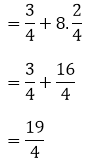∴ 9th term of the A. P. is 19/4.

2.(i) Which term of the AP 3, 8, 13, …. is 248?

(ii) Which term of the AP 84, 80, 76, … is 0?

(iii) Which term of the AP 4. 9, 14, …. is 254?

(iv) Which term of the AP 21. 42, 63, 84, … is 420?

(v) Which term of the AP 121, 117. 113, … is its first negative term?

Solution:

(i) Given A.P. is 3, 8, 13, ………..

First term (a) = 3

Common difference (d) = Second term – first term

= 8 – 3

= 5

We know that, nth term (an) = a + (n – 1)d

And, given nth term an = 248

248 = 3+(n – 1)5

248 = -2 + 5n

5n = 250

n =250/5 = 50

∴ 50th term in the A.P is 248.

(ii) Given A. P is 84, 80, 76, …………

First term (a) = 84

Common difference (d) = a2 – a

= 80 – 84

= – 4

We know that, nth term (an) = a +(n – 1)d

And, given nth term is 0

0 = 84 + (n – 1) – 4

84 = +4(n –  1)

n – 1 = 84/4 = 21

n = 21 + 1 = 22

∴ 22nd term in the A.P is 0.

(iii) Given A. P 4, 9, 14, …………

First term (a) = 4

Common difference (d) = a2 – a1

= 9 – 4

= 5

We know that, nth term (an) = a + (n – 1)d

And, given nth term is 254

4 + (n – 1)5 = 254

(n – 1)∙5 = 250

n – 1 = 250/5 = 50

n = 51

∴  51th term in the A.P is 254.

(iv) Given A. P 21, 42, 63, 84, ………

a = 21, d = a2 – a1

= 42 – 21

= 21

We know that, nth term (an) = a +(n – 1)d

And, given nth term = 420

21 + (n – 1)21 = 420

(n – 1)21 = 399

n – 1 = 399/21 = 19

n = 20

∴ 20th term is 420.

(v) Given A.P is 121, 117, 113, ………..

Fiat term (a) = 121

Common difference (d) = 117 – 121

= – 4

We know that, nth term an = a + (n – 1)d

And, for some nth term is negative i.e., an < 0

121 + (n – 1) – 4 < 0

121 + 4 – 4n < 0

125 – 4n < 0

4n > 125

n > 125/4

n > 31.25

The integer which comes after 31.25 is 32.

∴ 32nd term in the A.P will be the first negative term.

3.(i) Is 68 a term of the A.P. 7, 10, 13,… ?

(ii) Is 302 a term of the A.P. 3, 8, 13, …. ?

(iii) Is -150 a term of the A.P. 11, 8, 5, 2, … ?

Solutions:

(i) Given, A.P. 7, 10, 13,…

Here, a = 7 and d = a2 – a1 = 10 – 7 = 3

We know that, nth term an = a + (n – 1)d

Required to check nth term an = 68

a + (n – 1)d = 68

7 + (n – 1)3 = 68

7 + 3n – 3 = 68

3n + 4 = 68

3n = 64

⇒ n = 64/3, which is not a whole number.

Therefore, 68 is not a term in the A.P.

(ii) Given, A.P. 3, 8, 13,…

Here, a = 3 and d = a2 – a1 = 8 – 3 = 5

We know that, nth term an = a + (n – 1)d

Required to check nth term an = 302

a + (n – 1)d = 302

3 + (n – 1)5 = 302

3 + 5n – 5 = 302

5n – 2 = 302

5n = 304

⇒ n = 304/5, which is not a whole number.

Therefore, 302 is not a term in the A.P.

(iii) Given, A.P. 11, 8, 5, 2, …

Here, a = 11 and d = a2 – a1 = 8 – 11 = -3

We know that, nth term an = a + (n – 1)d

Required to check nth term an = -150

a + (n – 1)d = -150

11 + (n – 1)(-3) = -150

11 – 3n + 3 = -150

3n = 150 + 14

3n = 164

⇒ n = 164/3, which is not a whole number.

Therefore, -150 is not a term in the A.P.

4. How many terms are there in the A.P.?

(i) 7, 10, 13, ….., 43

(ii) -1, -5/6, -2/3, -1/2, … , 10/3

(iii) 7, 13, 19, …, 205

(iv) 18, 15½, 13, …., -47

Solution:

(i) Given, A.P. 7, 10, 13, ….., 43

Here, a = 7 and d = a2 – a1 = 10 – 7 = 3

We know that, nth term an = a + (n – 1)d

And, given nth term an = 43

a + (n – 1)d = 43

7 + (n – 1)(3) = 43

7 + 3n – 3 = 43

3n = 43 – 4

3n = 39

⇒ n = 13

Therefore, there are 13 terms in the given A.P.

(ii) Given, A.P. -1, -5/6, -2/3, -1/2, … , 10/3

Here, a = -1 and d = a2 – a1 = -5/6 – (-1) = 1/6

We know that, nth term an = a + (n – 1)d

And, given nth term an = 10/3

a + (n – 1)d = 10/3

-1 + (n – 1)(1/6) = 10/3

-1 + n/6 – 1/6 = 10/3

n/6 = 10/3 + 1 + 1/6

n/6 = (20 + 6 + 1)/6

n = (20 + 6 + 1)

⇒ n = 27

Therefore, there are 27 terms in the given A.P.

(iii) Given, A.P. 7, 13, 19, …, 205

Here, a = 7 and d = a2 – a1 = 13 – 7 = 6

We know that, nth term an = a + (n – 1)d

And, given nth term an = 205

a + (n – 1)d = 205

7 + (n – 1)(6) = 205

7 + 6n – 6 = 205

6n = 205 – 1

n = 204/6

⇒ n = 34

Therefore, there are 34 terms in the given A.P.

(iv) Given, A.P. 18, 15½, 13, …., -47

Here, a = 7 and d = a2 – a1 = 15½ – 18 = 5/2

We know that, nth term an = a + (n – 1)d

And, given nth term an = -47

a + (n – 1)d = 43

18 + (n – 1)(-5/2) = -47

18 – 5n/2 + 5/2 = -47

36 – 5n + 5 = -94

5n = 94 + 36 + 5

5n = 135

⇒ n = 27

Therefore, there are 27 terms in the given A.P.

5. The first term of an A.P. is 5, the common difference is 3 and the last term is 80; find the number of terms.

Solution:

Given,

a = 5 and d = 3

We know that, nth term an = a + (n – 1)d

So, for the given A.P. an = 5 + (n – 1)3 = 3n + 2

Also given, last term = 80

⇒ 3n + 2 = 80

3n = 78

n = 78/3 = 26

Therefore, there are 26 terms in the A.P.

6. The 6th and 17th terms of an A.P. are 19 and 41 respectively, find the 40th term.

Solution:

Given,

a6 = 19 and a17 = 41

We know that, nth term an = a + (n – 1)d

So,

a6 = a + (6-1)d

⇒ a + 5d = 19 …… (i)

Similarity,

a17 = a + (17 – 1)d

⇒ a + 16d = 41 …… (ii)

Solving (i) and (ii),

(ii) – (i) ⇒

a + 16d – (a + 5d) = 41 – 19

11d = 22

⇒ d = 2

Using d in (i), we get

a + 5(2) = 19

a = 19 – 10 = 9

Now, the 40th term is given by a40 = 9 + (40 – 1)2 = 9 + 78 = 87

Therefore the 40th term is 87.

7. If 9th term of an A.P. is zero, prove its 29th term is double the 19th term.

Solution:

Given,

a9 = 0

We know that, nth term an = a + (n – 1)d

So, a + (9 – 1)d = 0 ⇒ a + 8d = 0 ……(i)

Now,

29th term is given by a29 = a + (29 – 1)d

⇒ a29 = a + 28d

And, a29 = (a + 8d) + 20d [using (i)]

⇒ a29 = 20d ….. (ii)

Similarly, 19th term is given by a19 = a + (19 – 1)d

⇒ a19 = a + 18d

And, a19 = (a + 8d) + 10d [using (i)]

⇒ a19 = 10d …..(iii)

On comparing (ii) and (iii), it’s clearly seen that

a29 = 2(a19)

Therefore, 29th term is double the 19th term.

8. If 10 times the 10th term of an A.P. is equal to 15 times the 15th term, show that 25th term of the A.P. is zero.

Solution:

Given,

10 times the 10th term of an A.P. is equal to 15 times the 15th term.

We know that, nth term an = a + (n – 1)d

⇒ 10(a10) = 15(a15)

10(a + (10 – 1)d) = 15(a + (15 – 1)d)

10(a + 9d) = 15(a + 14d)

10a + 90d = 15a + 210d

5a + 120d = 0

5(a + 24d) = 0

a + 24d = 0

a + (25 – 1)d = 0

⇒ a25 = 0

Therefore, the 25th term of the A.P. is zero.

9. The 10th and 18th terms of an A.P. are 41 and 73 respectively. Find 26th term.

Solution:

Given,

A10 = 41 and a18 = 73

We know that, nth term an = a + (n – 1)d

So,

a10 = a + (10 – 1)d

⇒ a + 9d = 41 …… (i)

Similarity,

a18 = a + (18 – 1)d

⇒ a + 17d = 73 …… (ii)

Solving (i) and (ii),

(ii) – (i) ⇒

a + 17d – (a + 9d) = 73 – 41

8d = 32

⇒ d = 4

Using d in (i), we get

a + 9(4) = 41

a = 41 – 36 = 5

Now, the 26th term is given by a26 = 5 + (26 – 1)4 = 5 + 100 = 105

Therefore the 26th term is 105.

10. In a certain A.P. the 24th term is twice the 10th term. Prove that the 72nd term is twice the 34th term.

Solution:

Given,

24th term is twice the 10th term.

We know that, nth term an = a + (n – 1)d

⇒ a24 = 2(a10)

a + (24 – 1)d = 2(a + (10 – 1)d)

a + 23d = 2(a + 9d)

a + 23d = 2a + 18d

a = 5d …. (1)

Now, the 72nd term can be expressed as

a72 = a + (72 – 1)d

= a + 71d

= a + 5d + 66d

= a + a + 66d [using (1)]

= 2(a + 33d)

= 2(a + (34 – 1)d)

= 2(a34)

⇒ a72 = 2(a34)

Hence, the 72nd term is twice the 34th term of the given A.P.

11. The 26th, 11th and the last term of an A.P. are 0, 3 and -1/5, respectively. Find the common difference and the number of terms.

Solution:

Given,

a26 = 0 , a11 = 3 and an (last term) = -1/5 of an A.P.

We know that, nth term an = a + (n – 1)d

Then,

a26 = a + (26 – 1)d

⇒ a + 25d = 0 …..(1)

And,

a11 = a + (11 – 1)d

⇒ a + 10d = 3 …… (2)

Solving (1) and (2),

(1) – (2) ⇒

a + 25d – (a + 10d) = 0 – 3

15d = -3

⇒ d = -1/5

Using d in (1), we get

a + 25(-1/5) = 0

a = 5

Now, given that the last term an = -1/5

⇒ 5 + (n – 1)(-1/5) = -1/5

5 + -n/5 + 1/5 = -1/5

25 – n + 1 = -1

n = 27

Therefore, the A.P has 27 terms and its common difference is -1/5.

12. If the nth term of the A.P. 9, 7, 5, …. is same as the nth term of the A.P. 15, 12, 9, … find n.

Solution:

Given,

A.P1 = 9, 7, 5, …. and A.P2 = 15, 12, 9, …

And, we know that, nth term an = a + (n – 1)d

For A.P1,

a = 9, d = Second term – first term = 9 – 7 = -2

And, its nth term an = 9 + (n – 1)(-2) = 9 – 2n + 2

an = 11 – 2n …..(i)

Similarly, for A.P2

a = 15, d = Second term – first term = 12 – 15 = -3

And, its nth term an = 15 + (n – 1)(-3) = 15 – 3n + 3

an = 18 – 3n …..(ii)

According to the question, its given that

nth term of the A.P1 = nth term of the A.P2

⇒ 11 – 2n = 18 – 3n

n = 7

Therefore, the 7th term of the both the A.Ps are equal.

13. Find the 12th term from the end of the following arithmetic progressions:

(i) 3, 5, 7, 9, …. 201

(ii) 3,8,13, … ,253

(iii) 1, 4, 7, 10, … ,88

Solution:

In order the find the 12th term for the end of an A.P. which has n terms, its done by simply finding the ((n -12) + 1)th of the A.P

And we know, nth term an = a + (n – 1)d

(i) Given A.P = 3, 5, 7, 9, …. 201

Here, a = 3 and d = (5 – 3) = 2

Now, find the number of terms when the last term is known i.e, 201

an = 3 + (n – 1)2 = 201

3 + 2n – 2 = 201

2n = 200

n = 100

Hence, the A.P has 100 terms.

So, the 12th term from the end is same as (100 – 12 + 1)th of the A.P which is the 89th term.

⇒ a89 = 3 + (89 – 1)2

= 3 + 88(2)

= 3 + 176

= 179

Therefore, the 12th term from the end of the A.P is 179.

(ii) Given A.P = 3,8,13, … ,253

Here, a = 3 and d = (8 – 3) = 5

Now, find the number of terms when the last term is known i.e, 253

an = 3 + (n – 1)5 = 253

3 + 5n – 5 = 253

5n = 253 + 2 = 255

n = 255/5

n = 51

Hence, the A.P has 51 terms.

So, the 12th term from the end is same as (51 – 12 + 1)th of the A.P which is the 40th term.

⇒ a40 = 3 + (40 – 1)5

= 3 + 39(5)

= 3 + 195

= 198

Therefore, the 12th term from the end of the A.P is 198.

(iii) Given A.P = 1, 4, 7, 10, … ,88

Here, a = 1 and d = (4 – 1) = 3

Now, find the number of terms when the last term is known i.e, 88

an = 1 + (n – 1)3 = 88

1 + 3n – 3 = 88

3n = 90

n = 30

Hence, the A.P has 30 terms.

So, the 12th term from the end is same as (30 – 12 + 1)th of the A.P which is the 19th term.

⇒ a89 = 1 + (19 – 1)3

= 1 + 18(3)

= 1 + 54

= 55

Therefore, the 12th term from the end of the A.P is 55.

14. The 4th term of an A.P. is three times the first and the 7th term exceeds twice the third term by 1. Find the first term and the common difference.

Solution:

Let’s consider the first term and the common difference of the A.P to be a and d respectively.

Then, we know that an = a + (n – 1)d

Given conditions,

4th term of an A.P. is three times the first

Expressing this by equation we have,

⇒ a4 = 3(a)

a + (4 – 1)d = 3a

3d = 2a ⇒ a = 3d/2…….(i)

And,

7th term exceeds twice the third term by 1

⇒ a7 = 2(a3) + 1

a + (7 – 1)d = 2(a + (3–1)d) + 1

a + 6d = 2a + 4d + 1

a – 2d +1 = 0 ….. (ii)

Using (i) in (ii), we have

3d/2 – 2d + 1 = 0

3d – 4d + 2 = 0

d = 2

So, putting d = 2 in (i), we get a

⇒ a = 3

Therefore, the first term is 3 and the common difference is 2.

15. Find the second term and the nth term of an A.P. whose 6th term is 12 and the 8th term is 22.

Solution:

Given, in an A.P

a6 = 12 and a8 = 22

We know that an = a + (n – 1)d

So,

a6 = a + (6-1)d = a + 5d = 12 …. (i)

And,

a8 = a + (8-1)d = a + 7d = 22 ……. (ii)

Solving (i) and (ii), we have

(ii) – (i) ⇒

a + 7d – (a + 5d) = 22 – 12

2d = 10

d = 5

Putting d in (i) we get,

a + 5(5) = 12

a = 12 – 25

a = -13

Thus, for the A.P: a = -13 and d = 5

So, the nth term is given by an = a + (n-1)d

an = -13 + (n-1)5 = -13 + 5n – 5

⇒ an = 5n – 18

Hence, the second term is given by a2 = 5(2) – 18 = 10 – 18 = -8

16. How many numbers of two digit are divisible by 3?

Solution:

The first 2 digit number divisible by 3 is 12. And, the last 2 digit number divisible by 3 is 99.

So, this forms an A.P.

12, 15, 18, 21, …. , 99

Where, a = 12 and d = 3

Finding the number of terms in this A.P

⇒ 99 = 12 + (n-1)3

99 = 12 + 3n – 3

90 = 3n

n = 90/3 = 30

Therefore, there are 30 two digit numbers divisible by 3.

17. An A.P. consists of 60 terms. If the first and the last terms be 7 and 125 respectively, find 32nd term.

Solution:

Given, an A.P of 60 terms

And, a = 7 and a60 = 125

We know that an = a + (n – 1)d

⇒ a60 = 7 + (60 – 1)d = 125

7 + 59d = 125

59d = 118

d = 2

So, the 32nd term is given by

a32 = 7 + (32 -1)2 = 7 + 62 = 69

⇒ a32 = 69

18. The sum of 4th and 8th terms of an A.P. is 24 and the sum of the 6th and 10th terms is 34. Find the first term and the common difference of the A.P.

Solution:

Given, in an A.P

The sum of 4th and 8th terms of an A.P. is 24

⇒ a4 + a8 = 24

And, we know that an = a + (n – 1)d

[a + (4-1)d] + [a + (8-1)d] = 24

2a + 10d = 24

a + 5d = 12 …. (i)

Also given that,

the sum of the 6th and 10th terms is 34

⇒ a6 + a10 = 34

[a + 5d] + [a + 9d] = 34

2a + 14d = 34

a + 7d = 17 …… (ii)

Subtracting (i) form (ii), we have

a + 7d – (a + 5d) = 17 – 12

2d = 5

d = 5/2

Using d in (i) we get,

a + 5(5/2) = 12

a = 12 – 25/2

a = -1/2

Therefore, the first term is -1/2 and the common difference is 5/2.

19. The first term of an A.P. is 5 and its 100th term is -292. Find the 50th term of this A.P.

Solution:

Given, an A.P whose

a = 5 and a100 = -292

We know that an = a + (n – 1)d

a100 = 5 + 99d = -292

99d = -297

d = -3

Hence, the 50th term is

a50 = a + 49d = 5 + 49(-3) = 5 – 147 = -142

20. Find a30 – a20 for the A.P.

(i) -9, -14, -19, -24 (ii) a, a+d, a+2d, a+3d, ……

Solution:

We know that an = a + (n – 1)d

So, a30 – a20 = (a + 29d) – (a + 19) =10d

(i) Given A.P. -9, -14, -19, -24

Here, a = -9 and d = -14 – (-9) = = -14 + 9 = -5

So, a30 – a20 = 10d

= 10(-5)

= -50

(ii) Given A.P. a, a+d, a+2d, a+3d, ……

So, a30 – a20 = (a + 29d) – (a + 19d)

=10d

21. Write the expression an – ak for the A.P. a, a+d, a+2d, …..

Hence, find the common difference of the A.P. for which

(i) 11th term is 5 and 13th term is 79.

(ii) a10 – a5 = 200

(iii) 20th term is 10 more than the 18th term.

Solution:

Given A.P. a, a+d, a+2d, …..

So, an = a + (n-1)d = a + nd –d

And, ak = a + (k-1)d = a + kd – d

an – ak = (a + nd – d) – (a + kd – d)

= (n – k)d

(i) Given 11th term is 5 and 13th term is 79,

Here n = 13 and k = 11,

a13 – a11 = (13 – 11)d = 2d

⇒ 79 – 5 = 2d

d = 74/2 = 37

(ii) Given, a10 – a5 = 200

⇒ (10 – 5)d = 200

5d = 200

d = 40

(iii) Given, 20th term is 10 more than the 18th term.

⇒ a20 – a18 = 10

(20 – 18)d = 10

2d = 10

d = 5

22. Find n if the given value of x is the nth term of the given A.P.

(i) 25, 50, 75, 100, ; x = 1000 (ii) -1, -3, -5, -7, …; x = -151

(iii) 5½, 11, 16½, 22, ….; x = 550 (iv) 1, 21/11, 31/11, 41/11, …; x = 171/11

Solution:

(i) Given A.P. 25, 50, 75, 100, …… ,1000

Here, a = 25 d = 50 – 25 = 25

Last term (nth term) = 1000

We know that an = a + (n – 1)d

⇒ 1000 = 25 + (n-1)25

1000 = 25 + 25n – 25

n = 1000/25

n = 40

(ii) Given A.P. -1, -3, -5, -7, …., -151

Here, a = -1 d = -3 – (-1) = -2

Last term (nth term) = -151

We know that an = a + (n – 1)d

⇒ -151 = -1 + (n-1)(-2)

-151 = -1 – 2n + 2

n = 152/2

n = 76

(iii) Given A.P. 5½, 11, 16½, 22, … , 550

Here, a = 5½ d = 11 – (5½) = 5½ = 11/2

Last term (nth term) = 550

We know that an = a + (n – 1)d

⇒ 550 = 5½ + (n-1)(11/2)

550 x 2 = 11+ 11n – 11

1100 = 11n

n = 100

(iv) Given A.P. 1, 21/11, 31/11, 41/11, 171/11

Here, a = 1 d = 21/11 – 1 = 10/11

Last term (nth term) = 171/11

We know that an = a + (n – 1)d

⇒ 171/11 = 1 + (n-1)10/11

171 = 11 + 10n – 10

n = 170/10

n = 17

23. The eighth term of an A.P is half of its second term and the eleventh term exceeds one third of its fourth term by 1. Find the 15th term.

Solution:

Given, an A.P in which,

a8 = 1/2(a2)

a11 = 1/3(a4) + 1

We know that an = a + (n – 1)d

⇒ a8 = 1/2(a2)

a + 7d = 1/2(a + d)

2a + 14d = a + d

a + 13d = 0 …… (i)

And, a11 = 1/3(a4) + 1

a + 10d = 1/3(a + 3d) + 1

3a + 30d = a + 3d + 3

2a + 27d = 3 …… (ii)

Solving (i) and (ii), by (ii) – 2x(i) ⇒

2a + 27d – 2(a + 13d) = 3 – 0

d = 3

Putting d in (i) we get,

a + 13(3) = 0

a = -39

Thus, the 15th term a15 = -39 + 14(3) = -39 + 42 = 3

24. Find the arithmetic progression whose third term is 16 and the seventh term exceeds its fifth term by 12.

Solution:

Given, in an A.P

a3 = 16 and a7 = a5 + 12

We know that an = a + (n – 1)d

⇒ a + 2d = 16…… (i)

And,

a + 6d = a + 4d + 12

2d = 12

⇒ d = 6

Using d in (i), we have

a + 2(6) = 16

a = 16 – 12 = 4

Hence, the A.P is 4, 10, 16, 22, …….

25. The 7th term of an A.P. is 32 and its 13th term is 62. Find the A.P.

Solution:

Given,

a7 = 32 and a13 = 62

From an – ak = (a + nd – d) – (a + kd – d)

= (n – k)d

a13 – a7 = (13 – 7)d = 62 – 32 = 30

6d = 30

d = 5

Now,

a7 = a + (7 – 1)5 = 32

a + 30 = 32

a = 2

Hence, the A.P is 2, 7, 12, 17, ……

26. Which term of the A.P. 3, 10, 17, …. will be 84 more than its 13th term ?

Solution:

Given, A.P. 3, 10, 17, ….

Here, a = 3 and d = 10 – 3 = 7

According the question,

an = a13 + 84

Using an = a + (n – 1)d,

3 + (n – 1)7 = 3 + (13 – 1)7 + 84

3 + 7n – 7 = 3 + 84 + 84

7n = 168 + 7

n = 175/7

n = 25

Therefore, it the 25th term which is 84 more than its 13th term.

27. Two arithmetic progressions have the same common difference. The difference between their 100th terms is 100, what is the difference between their 1000th terms?

Solution:

Let the two A.Ps be A.P1 and A.P2

For A.P1 the first term = a and the common difference = d

And for A.P2 the first term = b and the common difference = d

So, from the question we have

a100 – b100 = 100

(a + 99d) – (b + 99d) = 100

a – b = 100

Now, the difference between their 1000th terms is,

(a + 999d) – (b + 999d) = a – b = 100

Therefore, the difference between their 1000th terms is also 100.

### RD Sharma Solutions for Class 10 Maths Chapter 9 Exercise 9.5 Page No: 9.30

1. Find the value of x for which (8x + 4), (6x – 2) and (2x + 7) are in A.P.
Solution:

Given,

(8x + 4), (6x – 2) and (2x + 7) are in A.P.

So, the common difference between the consecutive terms should be the same.

(6x – 2) – (8x + 4) = (2x + 7) – (6x – 2)

⇒ 6x – 2 – 8x – 4 = 2x + 7 – 6x + 2

⇒ -2x – 6 = -4x + 9

⇒ -2x + 4x = 9 + 6

⇒ 2x = 15

Therefore, x = 15/2

2. If x + 1, 3x and 4x + 2 are in A.P., find the value of x.
Solution:

Given,

x + 1, 3x and 4x + 2 are in A.P.

So, the common difference between the consecutive terms should be the same.

3x – x – 1 = 4x + 2 – 3x

⇒ 2x – 1 = x + 2

⇒ 2x – x = 2 + 1

⇒ x = 3

Therefore, x = 3

3. Show that (a – b)², (a² + b²) and (a + b)² are in A.P.
Solution:

If (a – b)², (a² + b²) and (a + b)² have to be in A.P. then,

It should satisfy the condition,

2b = a + c [for a, b, c are in A.P]

Thus,

2 (a² + b²) = (a – b)² + (a + b)²

2 (a² + b²) = a² + b² – 2ab + a² + b² + 2ab

2 (a² + b²) = 2a² + 2b² = 2 (a² + b²)

LHS = RHS

Hence proved.

4. The sum of three terms of an A.P. is 21 and the product of the first and the third terms exceeds the second term by 6, find three terms.
Solution:

Let’s consider the three terms of the A.P. to be a – d, a, a + d

so, the sum of three terms = 21

⇒ a – d + a + a + d = 21

⇒ 3a = 21

⇒ a = 7

And, product of the first and 3rd = 2nd term + 6

⇒ (a – d) (a + d) = a + 6

a² – d² = a + 6

⇒ (7 )² – d² = 7 + 6

⇒ 49 – d² = 13

⇒ d² = 49 – 13 = 36

⇒ d² = (6)²

⇒ d = 6

Hence, the terms are 7 – 6, 7, 7 + 6 ⇒ 1, 7, 13

5. Three numbers are in A.P. If the sum of these numbers be 27 and the product 648, find the numbers.
Solution:

Let the three numbers of the A.P. be a – d, a, a + d

From the question,

Sum of these numbers = 27

a – d + a + a + d = 27

⇒ 3a = 27

a = 27/3 = 9

Now, product of these numbers = 648

(a – d)(a)(a + d) = 648

a(a2 – d2) = 648

a2 – 648/a = d2

92 – (648/9) = d2

93 – 648 = 9d2

729 – 648 = 9d2

81 = 9d2

d2 = 9

d = 3 or -3

Hence, the terms are 9-3, 9 and 9+3 ⇒ 6, 9, 12 or 12, 9, 6 (for d = -3)

6. Find the four numbers in A.P., whose sum is 50 and in which the greatest number is 4 times the least.
Solution:

Let’s consider the four terms of the A.P. to be (a – 3d), (a – d), (a + d) and (a + 3d).

From the question,

Sum of these terms = 50

⇒ (a – 3d) + (a – d) + (a + d) + (a + 3d) = 50

⇒ a – 3d + a – d + a + d + a – 3d= 50

⇒ 4a = 50

⇒ a = 50/4 = 25/2

And, also given that the greatest number = 4 x least number

⇒ a + 3d = 4 (a – 3d)

⇒ a + 3d = 4a – 12d

⇒ 4a – a = 3d + 12d

⇒3a = 15d

⇒a = 5d

Using the value of a in the above equation, we have

⇒25/2 = 5d

⇒ d = 5/2

So, the terms will be:

(a – 3d) = (25/2 – 3(5/2)), (a – d) = (25/2 – 5/2), (25/2 + 5/2) and (25/2 + 3(5/2)).

⇒ 5, 10, 15, 20

### RD Sharma Solutions for Class 10 Maths Chapter 9 Exercise 9.6 Page No: 9.50

1. Find the sum of the following arithmetic progressions:

(i) 50, 46, 42, … to 10 terms

(ii) 1, 3, 5, 7, … to 12 terms

(iii) 3, 9/2, 6, 15/2, … to 25 terms

(iv) 41, 36, 31, … to 12 terms

(v) a + b, a – b, a – 3b, … to 22 terms

(vi) (x – y)2, (x2 + y2), (x + y)2, to 22 tams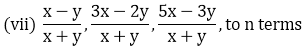(viii) – 26, – 24, – 22, …. to 36 terms

Solution:

In an A.P if the first term = a, common difference = d, and if there are n terms.

Then, sum of n terms is given by: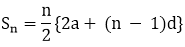(i) Given A.P.is 50, 46, 42 to 10 term.

First term (a) = 50

Common difference (d) = 46 – 50 = – 4

nth term (n) = 10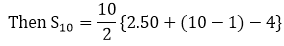= 5{100 – 9.4}

= 5{100 – 36}

= 5 × 64

∴ S10 = 320

(ii) Given A.P is, 1, 3, 5, 7, …..to 12 terms.

First term (a) = 1

Common difference (d) = 3 – 1 = 2

nth term (n) = 12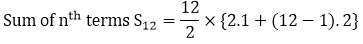= 6 × {2 + 22} = 6.24

∴ S12 = 144

(iii) Given A.P. is 3, 9/2, 6, 15/2, … to 25 terms

First term (a) = 3

Common difference (d) = 9/2 – 3 = 3/2

Sum of n terms Sn, given n = 25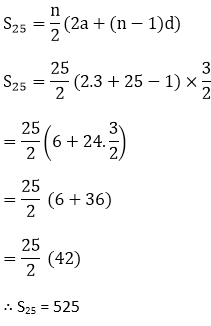(iv) Given expression is 41, 36, 31, ….. to 12 terms.

First term (a) = 41

Common difference (d) = 36 – 41 = -5

Sum of n terms Sn, given n = 12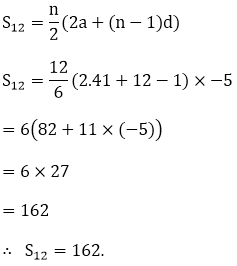(v) a + b, a – b, a – 3b, ….. to 22 terms

First term (a) = a + b

Common difference (d) = a – b – a – b = -2b

Sum of n terms Sn = n/2{2a(n – 1). d}

Here n = 22

S22 = 22/2{2.(a + b) + (22 – 1). -2b}

= 11{2(a + b) – 22b)

= 11{2a – 20b}

= 22a – 440b

∴S22 = 22a – 440b

(vi) (x – y)2,(x2 + y2), (x + y)2,… to n terms

First term (a) = (x – y)2

Common difference (d) = x2 + y2 – (x – y)2

= x2 + y2 – (x2 + y2 – 2xy)

= x2 + y2 – x2 + y2 + 2xy

= 2xy

Sum of nth terms Sn = n/2{2a + (n – 1). d}

= n/2{2(x – y)2 + (n – 1). 2xy}

= n{(x – y)2 + (n – 1)xy}

∴ Sn = n{(x — y)2 + (n — 1). xy)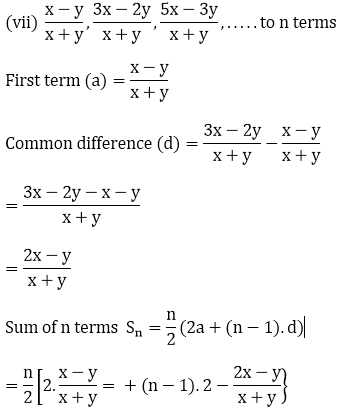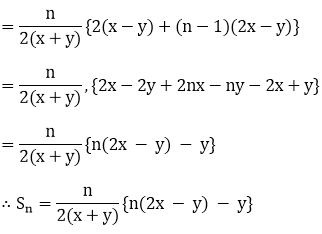(viii) Given expression -26, – 24. -22, to 36 terms

First term (a) = -26

Common difference (d) = -24 – (-26)

= -24 + 26 = 2

Sum of n terms, Sn = n/2{2a + (n – 1)d) for n = 36

Sn = 36/2{2(-26) + (36 – 1)2}

= 18[-52 + 70]

= 18×18

= 324

∴ Sn = 324

2. Find the sum to n terms of the A.P. 5, 2, –1, – 4, –7, …

Solution:

Given AP is 5, 2, -1, -4, -7, …..

Here, a = 5, d = 2 – 5 = -3

We know that,

Sn = n/2{2a + (n – 1)d}

= n/2{2.5 + (n – 1) – 3}

= n/2{10 – 3(n – 1)}

= n/2{13 – 3n)

∴ Sn = n/2(13 – 3n)

3. Find the sum of n terms of an A.P. whose the terms is given by an = 5 – 6n.

Solution:

Given nth term of the A.P as an = 5 – 6n

Put n = 1, we get

a1 = 5 – 6.1 = -1

So, first term (a) = -1

Last term (an) = 5 – 6n = 1

Then, Sn = n/2(-1 + 5 – 6n)

= n/2(4 – 6n) = n(2 – 3n)

4. Find the sum of last ten terms of the A.P. : 8, 10, 12, 14, .. , 126

Solution:

Given A.P. 8, 10, 12, 14, .. , 126

Here, a = 8 , d = 10 – 8 = 2

We know that, an = a + (n – 1)d

So, to find the number of terms

126 = 8 + (n – 1)2

126 = 8 + 2n – 2

2n = 120

n = 60

Next, let’s find the 51st term

a51 = 8 + 50(2) = 108

So, the sum of last ten terms is the sum of a51 + a52 + a53 + ……. + a60

Here, n = 10, a = 108 and l = 126

S = 10/2 [108 + 126]

= 5(234)

= 1170

Hence, the sum of last ten terms of the A.P is 1170.

5. Find the sum of first 15 terms of each of the following sequences having nth term as:

(i) an = 3 + 4n

(ii) bn = 5 + 2n

(iii) xn = 6 – n

(iv) yn = 9 – 5n

Solution:

(i) Given an A.P. whose nth term is given by an = 3 + 4n

To find the sum of the n terms of the given A.P., using the formula,

Sn = n(a + l)/ 2

Where, a = the first term l = the last term.

Putting n = 1 in the given an, we get

a = 3 + 4(1) = 3 + 4 = 7

For the last term (l), here n = 15

a15 = 3 + 4(15) = 63

So, Sn = 15(7 + 63)/2

= 15 x 35

= 525

Therefore, the sum of the 15 terms of the given A.P. is S15 = 525

(ii) Given an A.P. whose nth term is given by bn = 5 + 2n

To find the sum of the n terms of the given A.P., using the formula,

Sn = n(a + l)/ 2

Where, a = the first term l = the last term.

Putting n = 1 in the given bn, we get

a = 5 + 2(1) = 5 + 2 = 7

For the last term (l), here n = 15

a15 = 5 + 2(15) = 35

So, Sn = 15(7 + 35)/2

= 15 x 21

= 315

Therefore, the sum of the 15 terms of the given A.P. is S15 = 315

(iii) Given an A.P. whose nth term is given by xn = 6 – n

To find the sum of the n terms of the given A.P., using the formula

Sn = n(a + l)/ 2

Where, a = the first term l = the last term.

Putting n = 1 in the given xn, we get

a = 6 – 1 = 5

For the last term (l), here n = 15

a15 = 6 – 15 = -9

So, Sn = 15(5 – 9)/2

= 15 x (-2)

= -30

Therefore, the sum of the 15 terms of the given A.P. is S15 = -30

(iv) Given an A.P. whose nth term is given by yn = 9 – 5n

To find the sum of the n terms of the given A.P., using the formula,

Sn = n(a + l)/ 2

Where, a = the first term l = the last term.

Putting n = 1 in the given yn, we get

a = 9 – 5(1) = 9 – 5 = 4

For the last term (l), here n = 15

a15 = 9 – 5(15) = -66

So, Sn = 15(4 – 66)/2

= 15 x (-31)

= -465

Therefore, the sum of the 15 terms of the given A.P. is S15 = -465

6. Find the sum of first 20 terms the sequence whose nth term is an = An + B.

Solution:

Given an A.P. whose nth term is given by, an = An + B

We need to find the sum of first 20 terms.

To find the sum of the n terms of the given A.P., we use the formula,

Sn = n(a + l)/ 2

Where, a = the first term l = the last term,

Putting n = 1 in the given an, we get

a = A(1) + B = A + B

For the last term (l), here n = 20

A20 = A(20) + B = 20A + B

S20 = 20/2((A + B) + 20A + B)

= 10[21A + 2B]

= 210A + 20B

Therefore, the sum of the first 20 terms of the given A.P. is 210 A + 20B

7. Find the sum of first 25 terms of an A.P whose nth term is given by an = 2 – 3n.

Solution:

Given an A.P. whose nth term is given by an = 2 – 3n

To find the sum of the n terms of the given A.P., we use the formula,

Sn = n(a + l)/ 2

Where, a = the first term l = the last term.

Putting n = 1 in the given an, we get

a = 2 – 3(1) = -1

For the last term (l), here n = 25

a25 = 2 – 3(25) = -73

So, Sn = 25(-1 – 73)/2

= 25 x (-37)

= -925

Therefore, the sum of the 25 terms of the given A.P. is S25 = -925

8. Find the sum of first 25 terms of an A.P whose nth term is given by an = 7 – 3n.

Solution:

Given an A.P. whose nth term is given by an = 7 – 3n

To find the sum of the n terms of the given A.P., we use the formula,

Sn = n(a + l)/ 2

Where, a = the first term l = the last term.

Putting n = 1 in the given an, we get

a = 7 – 3(1) = 7 – 3 = 4

For the last term (l), here n = 25

a15 = 7 – 3(25) = -68

So, Sn = 25(4 – 68)/2

= 25 x (-32)

= -800

Therefore, the sum of the 15 terms of the given A.P. is S25 = -800

9. If the sum of a certain number of terms starting from first term of an A.P. is 25, 22, 19, . . ., is 116. Find the last term.

Solution:

Given the sum of the certain number of terms of an A.P. = 116

We know that, Sn = n/2[2a + (n − 1)d]

Where; a = first term for the given A.P.

d = common difference of the given A.P.

n = number of terms So for the given A.P.(25, 22, 19,…)

Here we have, the first term (a) = 25

The sum of n terms Sn = 116

Common difference of the A.P. (d) = a2 – a1 = 22 – 25 = -3

Now, substituting values in Sn

⟹ 116 = n/2[2(25) + (n − 1)(−3)]

⟹ (n/2)[50 + (−3n + 3)]  = 116

⟹ (n/2)[53 − 3n] = 116

⟹ 53n – 3n2 = 116 x 2

Thus, we get the following quadratic equation,

3n2 – 53n + 232 = 0

By factorization method of solving, we have

⟹ 3n2 – 24n – 29n + 232 = 0

⟹ 3n( n – 8 ) – 29 ( n – 8 ) = 0

⟹ (3n – 29)( n – 8 ) = 0

So, 3n – 29 = 0

⟹ n = 29/3

Also, n – 8 = 0

⟹ n = 8

Since, n cannot be a fraction, so the number of terms is taken as 8.

So, the term is:

a8 = a1 + 7d = 25 + 7(-3) = 25 – 21 = 4

Hence, the last term of the given A.P. such that the sum of the terms is 116 is 4.

10. (i) How many terms of the sequence 18, 16, 14….  should be taken so that their sum is zero.

(ii) How many terms are there in the A.P. whose first and fifth terms are -14 and 2 respectively and the sum of the terms is 40?

(iii) How many terms of the A.P. 9, 17, 25, . . . must be taken so that their sum is 636?

(iv) How many terms of the A.P. 63, 60, 57, . . . must be taken so that their sum is 693?

(v) How many terms of the A.P. is 27, 24, 21. . . should be taken that their sum is zero?

Solution:

(i) Given AP. is 18, 16, 14, …

We know that,

Sn = n/2[2a + (n − 1)d]

Here,

The first term (a) = 18

The sum of n terms (Sn) = 0 (given)

Common difference of the A.P.

(d) = a2  – a1 = 16 – 18 = – 2

So, on substituting the values in Sn

⟹ 0 = n/2[2(18) + (n − 1)(−2)]

⟹ 0 = n/2[36 + (−2n + 2)]

⟹ 0 = n/2[38 − 2n] Further, n/2

⟹ n = 0 Or, 38 – 2n = 0

⟹ 2n = 38

⟹ n = 19

Since, the number of terms cannot be zer0, hence the number of terms (n) should be 19.

(ii) Given, the first term (a) = -14, Filth term (a5) = 2, Sum of terms (Sn) = 40 of the A.P.

If the common difference is taken as d.

Then, a5 = a + 4d

⟹ 2 = -14 + 4d

⟹ 2 + 14 = 4d

⟹ 4d = 16

⟹ d = 4

Next, we know that S= n/2[2a + (n − 1)d]

Where; a = first term for the given A.P.

d = common difference of the given A.P.

n = number of terms

Now, on substituting the values in Sn

⟹ 40 = n/2[2(−14) + (n − 1)(4)]

⟹ 40 = n/2[−28 + (4n − 4)]

⟹ 40 = n/2[−32 + 4n]

⟹ 40(2) = – 32n + 4n2

So, we get the following quadratic equation,

4n2 – 32n – 80 = 0

⟹ n2 – 8n – 20 = 0

On solving by factorization method, we get

n2 – 10n + 2n – 20 = 0

⟹ n(n – 10) + 2( n – 10 ) = 0

⟹ (n + 2)(n – 10) = 0

Either, n + 2 = 0

⟹ n = -2

Or, n – 10 = 0

⟹ n = 10

Since the number of terms cannot be negative.

Therefore, the number of terms (n) is 10.

(iii) Given AP is 9, 17, 25,…

We know that,

Sn = n/2[2a + (n − 1)d]

Here we have,

The first term (a) = 9 and the sum of n terms (Sn) = 636

Common difference of the A.P. (d) = a2  – a1 = 17 – 9 = 8

Substituting the values in Sn, we get

⟹ 636 = n/2[2(9) + (n − 1)(8)]

⟹ 636 = n/2[18 + (8n − 8)]

⟹ 636(2) = (n)[10 + 8n]

⟹ 1271 = 10n + 8n2

Now, we get the following quadratic equation,

⟹ 8n2 + 10n – 1272 = 0

⟹ 4n2+ 5n – 636 = 0

On solving by factorisation method, we have

⟹ 4n2 – 48n + 53n – 636 = 0

⟹ 4n(n – 12) + 53(n – 12) = 0

⟹ (4n + 53)(n – 12) = 0

Either 4n + 53 = 0 ⟹ n = -53/4

Or, n – 12 = 0 ⟹ n = 12

Since, the number of terms cannot be a fraction.

Therefore, the number of terms (n) is 12.

(iv) Given A.P. is 63, 60, 57,…

We know that,

Sn = n/2[2a + (n − 1)d]

Here we have,

the first term (a) = 63

The sum of n terms (Sn) = 693

Common difference of the A.P. (d) = a2 – a1 = 60 – 63 = –3

On substituting the values in Sn we get

⟹ 693 = n/2[2(63) + (n − 1)(−3)]

⟹ 693 = n/2[126+(−3n + 3)]

⟹ 693 = n/2[129 − 3n]

⟹ 693(2) = 129n – 3n2

Now, we get the following quadratic equation.

⟹ 3n2 – 129n + 1386 = 0

⟹ n2 – 43n + 462

Solving by factorisation method, we have

⟹ n2 – 22n – 21n + 462 = 0

⟹ n(n – 22) -21(n – 22) = 0

⟹ (n – 22) (n – 21) = 0

Either, n – 22 = 0 ⟹ n = 22

Or,  n – 21 = 0 ⟹ n = 21

Now, the 22nd term will be a22 = a1 + 21d = 63 + 21( -3 ) = 63 – 63 = 0

So, the sum of 22 as well as 21 terms is 693.

Therefore, the number of terms (n) is 21 or 22.

(v) Given A.P. is 27, 24, 21. . .

We know that,

Sn = n/2[2a + (n − 1)d]

Here we have, the first term (a) = 27

The sum of n terms (Sn) = 0

Common difference of the A.P. (d) = a2 – a1 = 24 – 27 = -3

On substituting the values in Sn, we get

⟹ 0 = n/2[2(27) + (n − 1)( − 3)]

⟹ 0 = (n)[54 + (n – 1)(-3)]

⟹ 0 = (n)[54 – 3n + 3]

⟹ 0 = n [57 – 3n] Further we have, n = 0 Or, 57 – 3n = 0

⟹ 3n = 57

⟹ n = 19

The number of terms cannot be zero,

Hence, the numbers of terms (n) is 19.

11. Find the sum of the first

(i) 11 terms of the A.P. : 2, 6, 10, 14,  . . .

(ii) 13 terms of the A.P. : -6, 0, 6, 12, . . .

(iii) 51 terms of the A.P. : whose second term is 2 and fourth term is 8.

Solution:

We know that the sum of terms for different arithmetic progressions is given by

Sn = n/2[2a + (n − 1)d]

Where; a = first term for the given A.P. d = common difference of the given A.P. n = number of terms

(i) Given A.P 2, 6, 10, 14,… to 11 terms.

Common difference (d) = a2 – a1 = 10 – 6 = 4

Number of terms (n) = 11

First term for the given A.P. (a) = 2

So,

S11  = 11/2[2(2) + (11 − 1)4]

= 11/2[2(2) + (10)4]

= 11/2[4 + 40]

= 11 × 22

= 242

Hence, the sum of first 11 terms for the given A.P. is 242

(ii) Given A.P. – 6, 0, 6, 12, … to 13 terms.

Common difference (d) = a2 – a1 = 6 – 0 = 6

Number of terms (n) = 13

First term (a) = -6

So,

S13  = 13/2[2(− 6) + (13 –1)6]

= 13/2[(−12) + (12)6]

= 13/2 = 390

Hence, the sum of first 13 terms for the given A.P. is 390

(iii) 51 terms of an AP whose a2 = 2 and a4 = 8

We know that, a2 = a + d

2 = a + d  …(2)

Also, a4 = a + 3d

8 = a + 3d  … (2)

Subtracting (1) from (2), we have

2d = 6

d = 3

Substituting d = 3 in (1), we get

2 = a + 3

⟹ a = -1

Given that the number of terms (n) = 51

First term (a) = -1

So,

Sn  = 51/2[2(−1) + (51 − 1)(3)]

= 51/2[−2 + 150]

= 51/2

= 3774

Hence, the sum of first 51 terms for the A.P. is 3774.

12. Find the sum of

(i) the first 15 multiples of 8

(ii) the first 40 positive integers divisible by (a) 3 (b) 5 (c) 6.

(iii) all 3 – digit natural numbers which are divisible by 13.

(iv) all 3 – digit natural numbers which are multiples of 11.

Solution:

We know that the sum of terms for an A.P is given by

Sn = n/2[2a + (n − 1)d]

Where; a = first term for the given A.P. d = common difference of the given A.P. n = number of terms

(i) Given, first 15 multiples of 8.

These multiples form an A.P: 8, 16, 24, …… , 120

Here, a = 8 , d = 61 – 8 = 8 and the number of terms(n) = 15

Now, finding the sum of 15 terms, we have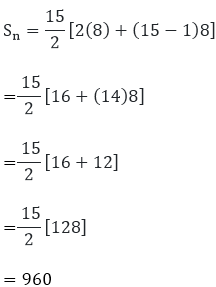\

Hence, the sum of the first 15 multiples of 8 is 960

(ii)(a) First 40 positive integers divisible by 3.

Hence, the first multiple is 3 and the 40th multiple is 120.

And, these terms will form an A.P. with the common difference of 3.

Here, First term (a) = 3

Number of terms (n) = 40

Common difference (d) = 3

So, the sum of 40 terms

S40  = 40/2[2(3) + (40 − 1)3]

= 20[6 + (39)3]

= 20(6 + 117)

= 20(123) = 2460

Thus, the sum of first 40 multiples of 3 is 2460.

(b) First 40 positive integers divisible by 5

Hence, the first multiple is 5 and the 40th multiple is 200.

And, these terms will form an A.P. with the common difference of 5.

Here, First term (a) = 5

Number of terms (n) = 40

Common difference (d) = 5

So, the sum of 40 terms

S40  = 40/2[2(5) + (40 − 1)5]

= 20[10 + (39)5]

= 20 (10 + 195)

= 20 (205) = 4100

Hence, the sum of first 40 multiples of 5 is 4100.

(c) First 40 positive integers divisible by 6

Hence, the first multiple is 6 and the 40th multiple is 240.

And, these terms will form an A.P. with the common difference of 6.

Here, First term (a) = 6

Number of terms (n) = 40

Common difference (d) = 6

So, the sum of 40 terms

S40  = 40/2[2(6) + (40 − 1)6]

= 20[12 + (39)6]

=20(12 + 234)

= 20(246) = 4920

Hence, the sum of first 40 multiples of 6 is 4920.

(iii) All 3 digit natural number which are divisible by 13.

So, we know that the first 3 digit multiple of 13 is 104 and the last 3 digit multiple of 13 is 988.

And, these terms form an A.P. with the common difference of 13.

Here, first term (a) = 104 and the last term (l) = 988

Common difference (d) = 13

Finding the number of terms in the A.P. by, an = a + (n − 1)d

We have,

988 = 104 + (n – 1)13

⟹ 988 = 104 + 13n -13

⟹ 988 = 91 + 13n

⟹ 13n = 897

⟹ n = 69

Now, using the formula for the sum of n terms, we get

S69  = 69/2[2(104) + (69 − 1)13]

= 69/2[208 + 884]

= 69/2

= 69(546)

= 37674

Hence, the sum of all 3 digit multiples of 13 is 37674.

(iv) All 3 digit natural number which are multiples of 11.

So, we know that the first 3 digit multiple of 11 is 110 and the last 3 digit multiple of 13 is 990.

And, these terms form an A.P. with the common difference of 11.

Here, first term (a) = 110 and the last term (l) = 990

Common difference (d) = 11

Finding the number of terms in the A.P. by, an = a + (n − 1)d

We get,

990 = 110 + (n – 1)11

⟹ 990 = 110 + 11n -11

⟹ 990 = 99 + 11n

⟹ 11n = 891

⟹ n = 81

Now, using the formula for the sum of n terms, we get

S81  = 81/2[2(110) + (81 − 1)11]

= 81/2[220 + 880]

= 81/2

= 81(550)

= 44550

Hence, the sum of all 3 digit multiples of 11 is 44550.

13. Find the sum:

(i) 2 + 4 + 6 + . . . + 200

(ii) 3 + 11 + 19 + . . . + 803

(iii) (-5) + (-8) + (-11) + . . . + (- 230)

(iv) 1 + 3 + 5 + 7 + . . . + 199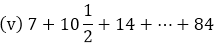(vi) 34 + 32 + 30 + . . . + 10

(vii) 25 + 28 + 31 + . . . + 100

Solution:

We know that the sum of terms for an A.P is given by

Sn = n/2[2a + (n − 1)d]

Where; a = first term for the given A.P. d = common difference of the given A.P. n = number of terms

Or Sn = n/2[a + l]

Where; a = first term for the given A.P. ;l = last term for the given A.P

(i) Given series. 2 + 4 + 6 + . . . + 200 which is an A.P

Where, a = 2 ,d = 4 – 2 = 2 and last term (an = l) = 200

We know that, an = a + (n – 1)d

So,

200 = 2 + (n – 1)2

200 = 2 + 2n – 2

n = 200/2 = 100

Now, for the sum of these 100 terms

S100 = 100/2 [2 + 200]

= 50(202)

= 10100

Hence, the sum of terms of the given series is 10100.

(ii) Given series. 3 + 11 + 19 + . . . + 803 which is an A.P

Where, a = 3 ,d = 11 – 3 = 8 and last term (an = l) = 803

We know that, an = a + (n – 1)d

So,

803 = 3 + (n – 1)8

803 = 3 + 8n – 8

n = 808/8 = 101

Now, for the sum of these 101 terms

S101 = 101/2 [3 + 803]

= 101(806)/2

= 101 x 403

= 40703

Hence, the sum of terms of the given series is 40703.

(iii) Given series (-5) + (-8) + (-11) + . . . + (- 230) which is an A.P

Where, a = -5 ,d = -8 – (-5) = -3 and last term (an = l) = -230

We know that, an = a + (n – 1)d

So,

-230 = -5 + (n – 1)(-3)

-230 = -5 – 3n + 3

3n = -2 + 230

n = 228/3 = 76

Now, for the sum of these 76 terms

S76 = 76/2 [-5 + (-230)]

= 38 x (-235)

= -8930

Hence, the sum of terms of the given series is -8930.

(iv) Given series. 1 + 3 + 5 + 7 + . . . + 199 which is an A.P

Where, a = 1 ,d = 3 – 1 = 2 and last term (an = l) = 199

We know that, an = a + (n – 1)d

So,

199 = 1 + (n – 1)2

199 = 1 + 2n – 2

n = 200/2 = 100

Now, for the sum of these 100 terms

S100 = 100/2 [1 + 199]

= 50(200)

= 10000

Hence, the sum of terms of the given series is 10000.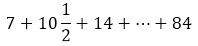(v) Given series which is an A.P

Where, a = 7, d = 10 ½ – 7 = (21 – 14)/2 = 7/2 and last term (an = l) = 84

We know that, an = a + (n – 1)d

So,

84 = 7 + (n – 1)(7/2)

168 = 14 + 7n – 7

n = (168 – 7)/7 = 161/7 = 23

Now, for the sum of these 23 terms

S23 = 23/2 [7 + 84]

= 23(91)/2

= 2093/2

Hence, the sum of terms of the given series is 2093/2.

(vi) Given series, 34 + 32 + 30 + . . . + 10 which is an A.P

Where, a = 34 ,d = 32 – 34 = -2 and last term (an = l) = 10

We know that, an = a + (n – 1)d

So,

10 = 34 + (n – 1)(-2)

10 = 34 – 2n + 2

n = (36 – 10)/2 = 13

Now, for the sum of these 13 terms

S13 = 13/2 [34 + 10]

= 13(44)/2

= 13 x 22

= 286

Hence, the sum of terms of the given series is 286.

(vii) Given series, 25 + 28 + 31 + . . . + 100 which is an A.P

Where, a = 25 ,d = 28 – 25 = 3 and last term (an = l) = 100

We know that, an = a + (n – 1)d

So,

100 = 25 + (n – 1)(3)

100 = 25 + 3n – 3

n = (100 – 22)/3 = 26

Now, for the sum of these 26 terms

S100 = 26/2 [25 + 100]

= 13(125)

= 1625

Hence, the sum of terms of the given series is 1625.

14. The first and the last terms of an A.P. are 17 and 350 respectively. If the common difference is 9, how many terms are there and what is their sum?

Solution:

Given, the first term of the A.P (a) = 17

The last term of the A.P (l) = 350

The common difference (d) of the A.P. = 9

Let the number of terms be n. And, we know that; l = a + (n – 1)d

So, 350 = 17 + (n- 1) 9

⟹ 350 = 17 + 9n – 9

⟹ 350 = 8 + 9n

⟹ 350 – 8 = 9n

Thus we get, n = 38

Now, finding the sum of terms

Sn  = n/2[a + l]

= 38/2(17 + 350)

= 19 × 367

= 6973

Hence, the number of terms is of the A.P is 38 and their sum is 6973.

15. The third term of an A.P. is 7 and the seventh term exceeds three times the third term by 2. Find the first term, the common difference and the sum of first 20 terms.

Solution:

Let’s consider the first term as a and the common difference as d.

Given,

a3 = 7 …. (1) and,

a7 = 3a3 + 2   …. (2)

So, using (1) in (2), we get,

a= 3(7) + 2 = 21 + 2 = 23  …. (3)

Also, we know that

an = a +(n – 1)d

So, the 3th term (for n = 3),

a3 = a + (3 – 1)d

⟹ 7 = a + 2d   (Using 1)

⟹ a = 7 – 2d     …. (4)

Similarly, for the 7th term (n = 7),

a7 = a + (7 – 1) d 24 = a + 6d = 23  (Using 3)

a = 23 – 6d  …. (5)

Subtracting (4) from (5), we get,

a – a = (23 – 6d) – (7 – 2d)

⟹ 0 = 23 – 6d – 7 + 2d

⟹ 0 = 16 – 4d

⟹ 4d = 16

⟹ d = 4

Now, to find a, we substitute the value of d in  (4), a =7 – 2(4)

⟹ a = 7 – 8

a = -1

Hence, for the A.P. a = -1 and d = 4

For finding the sum, we know that

Sn = n/2[2a + (n − 1)d] and n = 20 (given)

S20  = 20/2[2(−1) + (20 − 1)(4)]

= (10)[-2 + (19)(4)]

= (10)[-2 + 76]

= (10)

= 740

Hence, the sum of first 20 terms for the given A.P. is 740

16. The first term of an A.P. is 2 and the last term is 50. The sum of all these terms is 442. Find the common difference.

Solution:

Given,

The first term of the A.P (a) = 2

The last term of the A.P (l) = 50

Sum of all the terms Sn = 442

So, let the common difference of the A.P. be taken as d.

The sum of all the terms is given as,

442 = (n/2)(2 + 50)

⟹ 442 = (n/2)(52)

⟹ 26n = 442

⟹ n = 17

Now, the last term is expressed as

50 = 2 + (17 – 1)d

⟹ 50 = 2 + 16d

⟹ 16d = 48

⟹ d = 3

Thus, the common difference of the A.P. is d = 3.

17. If 12th term of an A.P. is -13 and the sum of the first four terms is 24, what is the sum of first 10 terms?

Solution:

Let us take the first term as a and the common difference as d.

Given,

a12 = -13 S4 = 24

Also, we know that a= a + (n – 1)d

So, for the 12th term

a12 = a + (12 – 1)d = -13

⟹ a + 11d = -13

a = -13 – 11d  …. (1)

And, we that for sum of terms

Sn = n/2[2a + (n − 1)d]

Here, n = 4

S4  = 4/2[2(a) + (4 − 1)d]

⟹ 24 = (2)[2a + (3)(d)]

⟹ 24 = 4a + 6d

⟹ 4a = 24 – 6d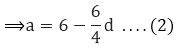Subtracting (1) from (2), we have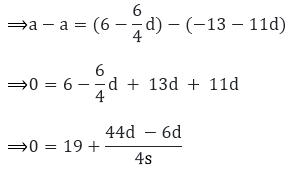Further simplifying for d, we get,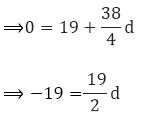⟹ -19 × 2 = 19d

⟹ d = – 2

On substituting the value of d in (1), we find a

a = -13 – 11(-2)

a = -13 + 22

a = 9

Next, the sum of 10 term is given by

S10  = 10/2[2(9) + (10 − 1)(−2)]

= (5)[19 + (9)(-2)]

= (5)(18 – 18) = 0

Thus, the sum of first 10 terms for the given A.P. is S10 = 0.

18.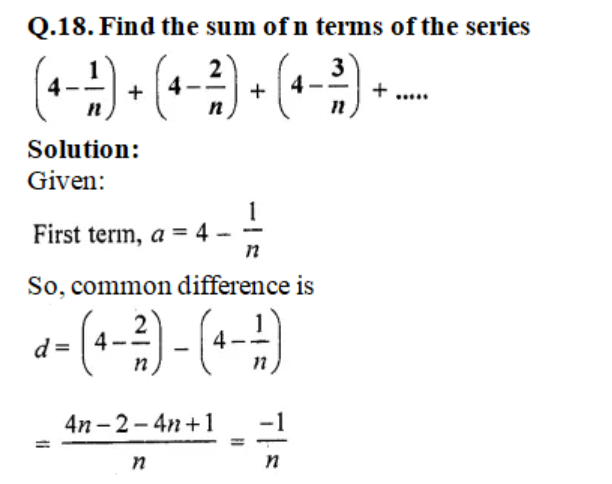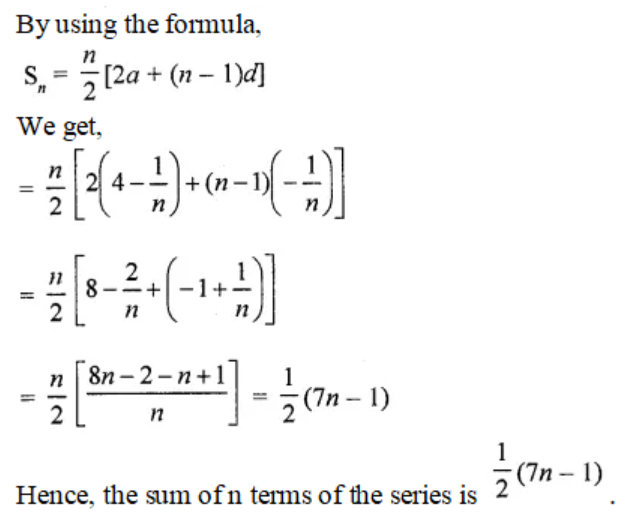19. In an A.P., if the first term is 22, the common difference is – 4 and the sum to n terms is 64, find n.

Solution:

Given that,

a = 22, d = – 4 and Sn = 64

Let us consider the number of terms as n.

For sum of terms in an A.P, we know that

Sn = n/2[2a + (n − 1)d]

Where; a = first term for the given A.P. d = common difference of the given A.P. n = number of terms

So,

⟹ Sn = n/2[2(22) + (n − 1)(−4)]

⟹ 64 = n/2[2(22) + (n − 1)(−4)]

⟹ 64(2) = n(48 – 4n)

⟹ 128 = 48n – 4n2

After rearranging the terms, we have a quadratic equation

4n2 – 48n + 128 = 0,

n2 – 12n + 32 = 0 [dividing by 4 on both sides]

n2 – 12n + 32 = 0

Solving by factorisation method,

n2 – 8n – 4n + 32 = 0

n ( n – 8 ) – 4 ( n – 8 ) = 0

(n – 8) (n – 4) = 0

So, we get n – 8 = 0 ⟹ n = 8

Or, n – 4 = 0 ⟹ n = 4

Hence, the number of terms can be either n = 4 or 8.

20. In an A.P., if the 5th and 12th terms are 30 and 65 respectively, what is the sum of first 20 terms?

Solution:

Let’s take the first term as a and the common difference to be d

Given that,

a5 = 30  and a12 = 65

And, we know that an = a + (n – 1)d

So,

a5 = a + (5 – 1)d

30 = a + 4d

a = 30 – 4d   …. (i)

Similarly, a12 = a + (12 – 1) d

65 = a + 11d

a = 65 – 11d …. (ii)

Subtracting (i) from (ii), we have

a – a = (65 – 11d) – (30 – 4d)

0 = 65 – 11d – 30 + 4d

0 = 35 – 7d

7d = 35

d = 5

Putting d in (i), we get

a = 30 – 4(5)

a = 30 – 20

a = 10

Thus for the A.P; d = 5 and a = 10

Next, to find the sum of first 20 terms of this A.P., we use the following formula for the sum of n terms of an A.P.,

Sn = n/2[2a + (n − 1)d]

Where;

a = first term of the given A.P.

d = common difference of the given A.P.

n = number of terms

Here n = 20, so we have

S20 = 20/2[2(10) + (20 − 1)(5)]

= (10)[20 + (19)(5)]

= (10)[20 + 95]

= (10)

= 1150

Hence, the sum of first 20 terms for the given A.P. is 1150

21. Find the sum of first 51 terms of an A.P. whose second and third terms are 14 and 18 respectively.

Solution:

Let’s take the first term as a and the common difference as d.

Given that,

a2 = 14 and a3 = 18

And, we know that an = a + (n – 1)d

So,

a2 = a + (2 – 1)d

⟹ 14 = a + d

⟹ a = 14 – d …. (i)

Similarly,

a3 = a + (3 – 1)d

⟹ 18 = a + 2d

⟹ a = 18 – 2d …. (ii)

Subtracting (i) from (ii), we have

a – a = (18 – 2d) – (14 – d)

0 = 18 – 2d – 14 + d

0 = 4 – d

d = 4

Putting d in (i), to find a

a = 14 – 4

a = 10

Thus, for the A.P. d = 4 and a = 10

Now, to find sum of terms

Sn = n/2(2a + (n − 1)d)

Where,

a = the first term of the A.P.

d = common difference of the A.P.

n = number of terms So, using the formula for

n = 51,

⟹ S51  = 51/2[2(10) + (51 – 1)(4)]

= 51/2[20 + (40)4]

= 51/2

= 51(110)

= 5610

Hence, the sum of the first 51 terms of the given A.P. is 5610

22. If the sum of 7 terms of an A.P. is 49 and that of 17 terms is 289, find the sum of n terms.

Solution:

Given,

Sum of 7 terms of an A.P. is 49

⟹ S7 = 49

And, sum of 17 terms of an A.P. is 289

⟹ S17 = 289

Let the first term of the A.P be a and common difference as d.

And, we know that the sum of n terms of an A.P is

Sn = n/2[2a + (n − 1)d]

So,

S7 = 49 = 7/2[2a + (7 – 1)d]

= 7/2 [2a + 6d]

= 7[a + 3d]

⟹ 7a + 21d = 49

a + 3d = 7 ….. (i)

Similarly,

S17 = 17/2[2a + (17 – 1)d]

= 17/2 [2a + 16d]

= 17[a + 8d]

⟹ 17[a + 8d] = 289

a + 8d = 17 ….. (ii)

Now, subtracting (i) from (ii), we have

a + 8d – (a + 3d) = 17 – 7

5d = 10

d = 2

Putting d in (i), we find a

a + 3(2) = 7

a = 7 – 6 = 1

So, for the A.P: a = 1 and d = 2

For the sum of n terms is given by,

Sn  = n/2[2(1) + (n − 1)(2)]

= n/2[2 + 2n – 2]

= n/2[2n]

= n2

Therefore, the sum of n terms of the A.P is given by n2.

23. The first term of an A.P. is 5, the last term is 45 and the sum is 400. Find the number of terms and the common difference.

Solution:

Sum of first n terms of an A.P is given by Sn = n/2(2a + (n − 1)d)

Given,

First term (a) = 5, last term (an) = 45 and sum of n terms (Sn) = 400

Now, we know that

an = a + (n – 1)d

⟹ 45 = 5 + (n – 1)d

⟹ 40 = nd – d

⟹ nd – d = 40 …. (1)

Also,

S= n/2(2(a) + (n − 1)d)

400 = n/2(2(5) + (n − 1)d)

800 = n (10 + nd – d)

800 = n (10 + 40) [using (1)]

⟹ n = 16

Putting n in (1), we find d

nd – d = 40

16d – d = 40

15d = 40

d = 8/3

Therefore, the common difference of the given A.P. is 8/3.

24. In an A.P. the first term is 8, nth term is 33 and the sum of first n term is 123. Find n and the d, the common difference.

Solution:

Given,

The first term of the A.P (a) = 8

The nth term of the A.P (l) = 33

And, the sum of all the terms Sn = 123

Let the common difference of the A.P. be d.

So, find the number of terms by

123 = (n/2)(8 + 33)

123 = (n/2)(41)

n = (123 x 2)/ 41

n = 246/41

n = 6

Next, to find the common difference of the A.P. we know that

l = a + (n – 1)d

33 = 8 + (6 – 1)d

33 = 8 + 5d

5d = 25

d = 5

Thus, the number of terms is n = 6 and the common difference of the A.P. is d = 5.

25. In an A.P. the first term is 22, nth term is -11 and the sum of first n term is 66. Find n and the d, the common difference.

Solution:

Given,

The first term of the A.P (a) = 22

The nth term of the A.P (l) = -11

And, sum of all the terms Sn = 66

Let the common difference of the A.P. be d.

So, finding the number of terms by

66 = (n/2)[22 + (−11)]

66 = (n/2)[22 − 11]

(66)(2) = n(11)

6 × 2 = n

n = 12

Now, for finding d

We know that, l = a + (n – 1)d

– 11 = 22 + (12 – 1)d

-11 = 22 + 11d

11d = – 33

d = – 3

Hence, the number of terms is n = 12 and the common difference d = -3

26. The first and the last terms of an A.P. are 7 and 49 respectively. If sum of all its terms is 420, find the common difference.

Solution:

Given,

First term (a) = 7, last term (an) = 49 and sum of n terms (Sn) = 420

Now, we know that

an = a + (n – 1)d

⟹ 49 = 7 + (n – 1)d

⟹ 43 = nd – d

⟹ nd – d = 42  ….. (1)

Next,

Sn = n/2(2(7) + (n − 1)d)

⟹ 840 = n[14 + nd – d]

⟹ 840 = n[14 + 42] [using (1)]

⟹ 840 = 54n

⟹ n = 15 …. (2)

So, by substituting (2) in (1), we have

nd – d = 42

⟹ 15d – d = 42

⟹ 14d = 42

⟹ d = 3

Therefore, the common difference of the given A.P. is 3.

27. The first and the last terms of an A.P are 5 and 45 respectively. If the sum of all its terms is 400, find its common difference.

Solution:

Given,

First term (a) = 5 and the last term (l) = 45

Also, Sn = 400

We know that,

an = a + (n – 1)d

⟹ 45 = 5 + (n – 1)d

⟹ 40 = nd – d

⟹ nd – d = 40  ….. (1)

Next,

Sn = n/2(2(5) + (n − 1)d)

⟹ 400 = n[10 + nd – d]

⟹ 800 = n[10 + 40] [using (1)]

⟹ 800 = 50n

⟹ n = 16 …. (2)

So, by substituting (2) in (1), we have

nd – d = 40

⟹ 16d – d = 40

⟹ 15d = 40

⟹ d = 8/3

Therefore, the common difference of the given A.P. is 8/3.

28. The sum of first q terms of an A.P. is 162. The ratio of its 6th term to its 13th term is 1: 2. Find the first and 15th term of the A.P.

Solution:

Let a be the first term and d be the common difference.

And we know that, sum of first n terms is:

Sn = n/2(2a + (n − 1)d)

Also, nth term is given by an = a + (n – 1)d

From the question, we have

Sq = 162 and a: a13 = 1 : 2

So,

2a= a13

⟹ 2 [a + (6 – 1d)] = a + (13 – 1)d

⟹ 2a + 10d = a + 12d

⟹ a = 2d  …. (1)

And, S9 = 162

⟹ S9 = 9/2(2a + (9 − 1)d)

⟹ 162 = 9/2(2a + 8d)

⟹ 162 × 2 = 9[4d + 8d]  [from (1)]

⟹ 324 = 9 × 12d

⟹ d = 3

⟹ a = 2(3) [from (1)]

⟹ a = 6

Hence, the first term of the A.P. is 6

For the 15th term, a15 = a + 14d = 6 + 14 × 3 = 6 + 42

Therefore, a15 = 48

29. If the 10th term of an A.P. is 21 and the sum of its first 10 terms is 120, find its nth term.

Solution:

Let’s consider a to be the first term and d be the common difference.

And we know that, sum of first n terms is:

Sn = n/2(2a + (n − 1)d) and nth term is given by: an = a + (n – 1)d

Now, from the question we have

S10 = 120

⟹ 120 = 10/2(2a + (10 − 1)d)

⟹ 120 = 5(2a + 9d)

⟹ 24 = 2a + 9d …. (1)

Also given that, a10 = 21

⟹ 21 = a + (10 – 1)d

⟹ 21 = a + 9d …. (2)

Subtracting (2) from (1), we get

24 – 21 = 2a + 9d – a – 9d

⟹a = 3

Now, on putting a = 3 in equation (2), we get

3 + 9d = 21

9d = 18

d = 2

Thus, we have the first term(a) = 3 and the common difference(d) = 2

Therefore, the nth term is given by

an  = a + (n – 1)d = 3 + (n – 1)2

= 3 + 2n -2

= 2n + 1

Hence, the nth term of the A.P is (an) = 2n + 1.

30. The sum of first 7 terms of an A.P. is 63 and the sum of its next 7 terms is 161. Find the 28th term of this A.P.

Solution:

Let’s take a to be the first term and d to be the common difference.

And we know that, sum of first n terms

Sn = n/2(2a + (n − 1)d)

Given that sum of the first 7 terms of an A.P. is 63.

S7 = 63

And sum of next 7 terms is 161.

So, the sum of first 14 terms = Sum of first 7 terms + sum of next 7 terms

S14 = 63 + 161 = 224

Now, having

S7 = 7/2(2a + (7 − 1)d)

⟹ 63(2) = 7(2a + 6d)

⟹ 9 × 2 = 2a + 6d

⟹ 2a + 6d = 18 . . . . (1)

And,

S14 = 14/2(2a + (14 − 1)d)

⟹ 224 = 7(2a + 13d)

⟹ 32 = 2a + 13d …. (2)

Now, subtracting (1) from (2), we get

⟹ 13d – 6d = 32 – 18

⟹ 7d = 14

⟹ d = 2

Using d in (1), we have

2a + 6(2) = 18

2a = 18 – 12

a = 3

Thus, from nth term

⟹ a28  = a + (28 – 1)d

= 3 + 27 (2)

= 3 + 54 = 57

Therefore, the 28th term is 57.

31. The sum of first seven terms of an A.P. is 182. If its 4th and 17th terms are in ratio 1: 5, find the A.P.

Solution:

Given that,

S17 = 182

And, we know that the sum of first n term is:

S= n/2(2a + (n − 1)d)

So,

S7 = 7/2(2a + (7 − 1)d)

182 × 2 = 7(2a + 6d)

364 = 14a + 42d

26 = a + 3d

a = 26 – 3d … (1)

Also, it’s given that 4th term and 17th term are in a ratio of 1: 5. So, we have

⟹ 5(a4) = 1(a17)

⟹ 5 (a + 3d) = 1 (a + 16d)

⟹ 5a + 15d = a + 16d

⟹ 4a = d …. (2)

Now, substituting (2) in (1), we get

⟹ 4 ( 26 – 3d ) = d

⟹ 104 – 12d = d

⟹ 104 = 13d

⟹ d = 8

Putting d in (2), we get

⟹ 4a = d

⟹ 4a = 8

⟹ a = 2

Therefore, the first term is 2 and the common difference is 8. So, the A.P. is 2, 10, 18, 26, . ..

32. The nth term of an A.P is given by (-4n + 15). Find the sum of first 20 terms of this A.P.

Solution:

Given,

The nth term of the A.P = (-4n + 15)

So, by putting n = 1 and n = 20 we can find the first ans 20th term of the A.P

a = (-4(1) + 15) = 11

And,

a20 = (-4(20) + 15) = -65

Now, for find the sum of 20 terms of this A.P we have the first and last term.

So, using the formula

Sn = n/2(a + l)

S20  = 20/2(11 + (-65))

= 10(-54)

= -540

Therefore, the sum of first 20 terms of this A.P. is -540.

33. In an A.P. the sum of first ten terms is -150 and the sum of its next 10 term is -550. Find the A.P.

Solution:

Let’s take a to be the first term and d to be the common difference.

And we know that, sum of first n terms

Sn = n/2(2a + (n − 1)d)

Given that sum of the first 10 terms of an A.P. is -150.

S10 = -150

And the sum of next 10 terms is -550.

So, the sum of first 20 terms = Sum of first 10 terms + sum of next 10 terms

S20 = -150 + -550 = -700

Now, having

S10 = 10/2(2a + (10 − 1)d)

⟹ -150 = 5(2a + 9d)

⟹ -30 = 2a + 9d

⟹ 2a + 9d = -30 . . . . (1)

And,

S20 = 20/2(2a + (20 − 1)d)

⟹ -700 = 10(2a + 19d)

⟹ -70 = 2a + 19d …. (2)

Now, subtracting (1) from (2), we get

⟹ 19d – 9d = -70 – (-30)

⟹ 10d = -40

⟹ d = -4

Using d in (1), we have

2a + 9(-4) = -30

2a = -30 + 36

a = 6/2 = 3

Hence, we have a = 3 and d = -4

So, the A.P is 3, -1, -5, -9, -13,…..

34. Sum of the first 14 terms of an A.P. is 1505 and its first term is 10. Find its 25th term.

Solution

Given,

First term of the A.P is 1505 and

S14 = 1505

We know that, the sum of first n terms is

Sn = n/2(2a + (n − 1)d)

So,

S14 = 14/2(2(10) + (14 − 1)d) = 1505

7(20 + 13d) = 1505

20 + 13d = 215

13d = 215 – 20

d = 195/13

d =15

Thus, the 25th term is given by

a25 = 10 + (25 -1)15

= 10 + (24)15

= 10 + 360

= 370

Therefore, the 25th term of the A.P is 370

35. In an A.P. , the first term is 2, the last term is 29 and the sum of the terms is 155. Find the common difference of the A.P.

Solution:

Given,

The first term of the A.P. (a) = 2

The last term of the A.P. (l) = 29

And, sum of all the terms (Sn) = 155

Let the common difference of the A.P. be d.

So, find the number of terms by sum of terms formula

Sn = n/2 (a + l)

155 = n/2(2 + 29)

155(2) = n(31)

31n = 310

n = 10

Using n for the last term, we have

l = a + (n – 1)d

29 = 2 + (10 – 1)d

29 = 2 + (9)d

29 – 2 = 9d

9d = 27

d = 3

Hence, the common difference of the A.P. is d = 3

36. The first and the last term of an A.P are 17 and 350 respectively. If the common difference is 9, how many terms are there and what is their sum?

Solution:

Given,

In an A.P first term (a) = 17 and the last term (l) = 350

And, the common difference (d) = 9

We know that,

an = a + (n – 1)d

so,

an = l = 17 + (n – 1)9 = 350

17 + 9n – 9 = 350

9n = 350 – 8

n = 342/9

n = 38

So, the sum of all the term of the A.P is given by

Sn = n/2 (a + l)

= 38/2(17 + 350)

= 19(367)

= 6973

Therefore, the sum of terms of the A.P is 6973.

37. Find the number of terms of the A.P. –12, –9, –6, . . . , 21. If 1 is added to each term of this A.P., then find the sum of all terms of the A.P. thus obtained.

Solution:

Given,

First term, a = -12

Common difference, d = a2 – a1 = – 9 – (- 12)

d = – 9 + 12 = 3

And, we know that nth term = an = a + (n – 1)d

⟹ 21 = -12 + (n – 1)3

⟹ 21 = -12 + 3n – 3

⟹ 21 = 3n – 15

⟹ 36 = 3n

⟹  n = 12

Thus, the number of terms is 12.

Now, if 1 is added to each of the 12 terms, the sum will increase by 12.

Hence, the sum of all the terms of the A.P. so obtained is

⟹ S12 + 12  = 12/2[a + l] + 12

= 6[-12 + 21] + 12

= 6 × 9 + 12

= 66

Therefore, the sum after adding 1 to each of the terms in the A.P is 66.

## Frequently Asked Questions on RD Sharma Solutions for Class 10 Maths Chapter 9

### Is RD Sharma Solutions for Class 10 Maths Chapter 9 important from exam point of view?

Yes, all the chapters of RD Sharma Solutions for Class 10 Maths Chapter 9 are important from an exam perspective. As this chapter explains arithmetic progression which is important for board exams as well as for higher studies. So studying these concepts makes learning easier for students. Most of the questions in exams come from this chapter.

### Give me a short summary on RD Sharma Solutions for Class 10 Maths Chapter 9?

RD Sharma Solutions for Class 10 Maths Chapter 9 has 6 exercises. The chapter deals with different topics related to the arithmetic progression that includes:
1. Understanding sequences
2. Arithmetic progressions
3. Describing the sequence by writing the algebraic formula for its terms
4. Find the sum of terms in an A.P.
5. Solving various word problems related to arithmetic progressions

### What are the key benefits of learning RD Sharma Solutions for Class 10 Maths Chapter 9?

The answers of RD Sharma Solutions for Class 10 Maths Chapter 9 have come in handy. This makes it easy to clear all the doubts related to arithmetic progression. Easily solve all the questions easily which have been given for assignments by the Class 10 students.